# RD Sharma Solutions for Class 8 Maths Chapter 1 - Rational Numbers

RD Sharma Solutions for Class 8 Maths Chapter 1- Rational Numbers are available here. Our expert faculty team has prepared solutions in order to help you with your exam preparation to obtain good marks in Maths. If you wish to secure an excellent score, solving RD Sharma Class 8 Solutions is an utmost necessity. This chapter mainly deals with problems based on rational numbers, whole numbers, natural numbers, representation of rational numbers on the number line. In order to help you understand and solve the problems, we, at BYJU’S, have prepared the RD Sharma Class 8 Chapter 1 where solutions are solved in detail. Download pdf of Class 8 Chapter 1, in their respective links.

## Download PDF of RD Sharma Solutions for Class 8 Maths Chapter 1 – Rational Numbers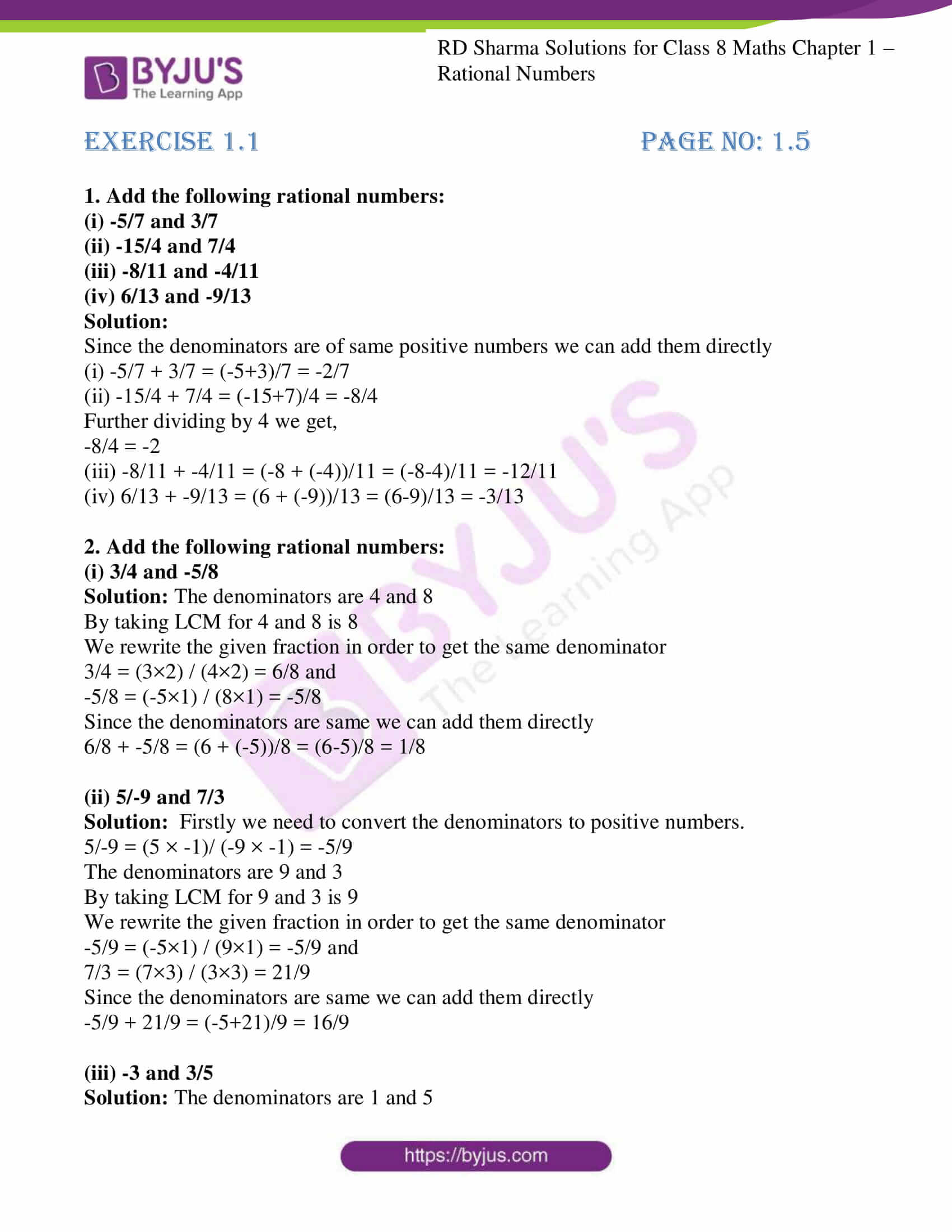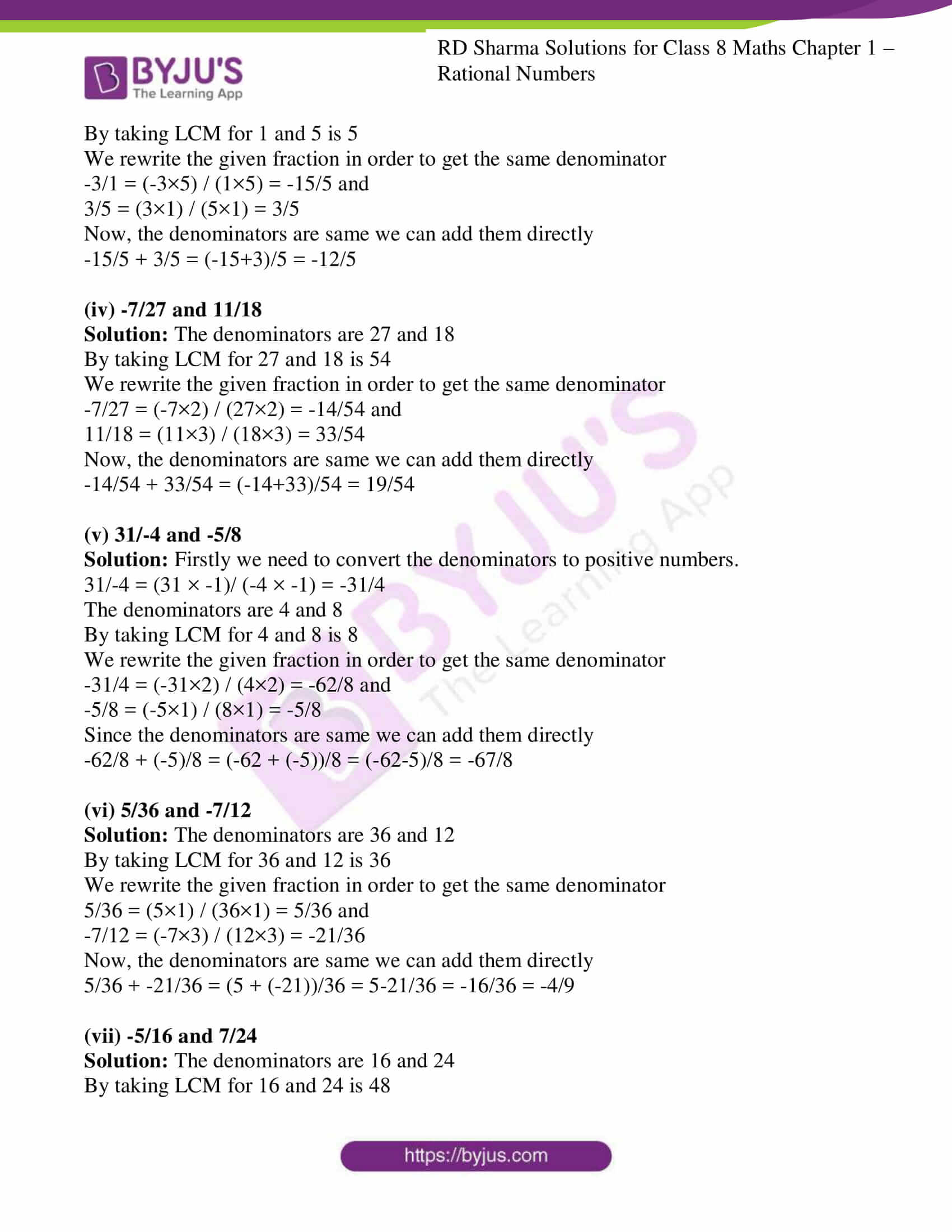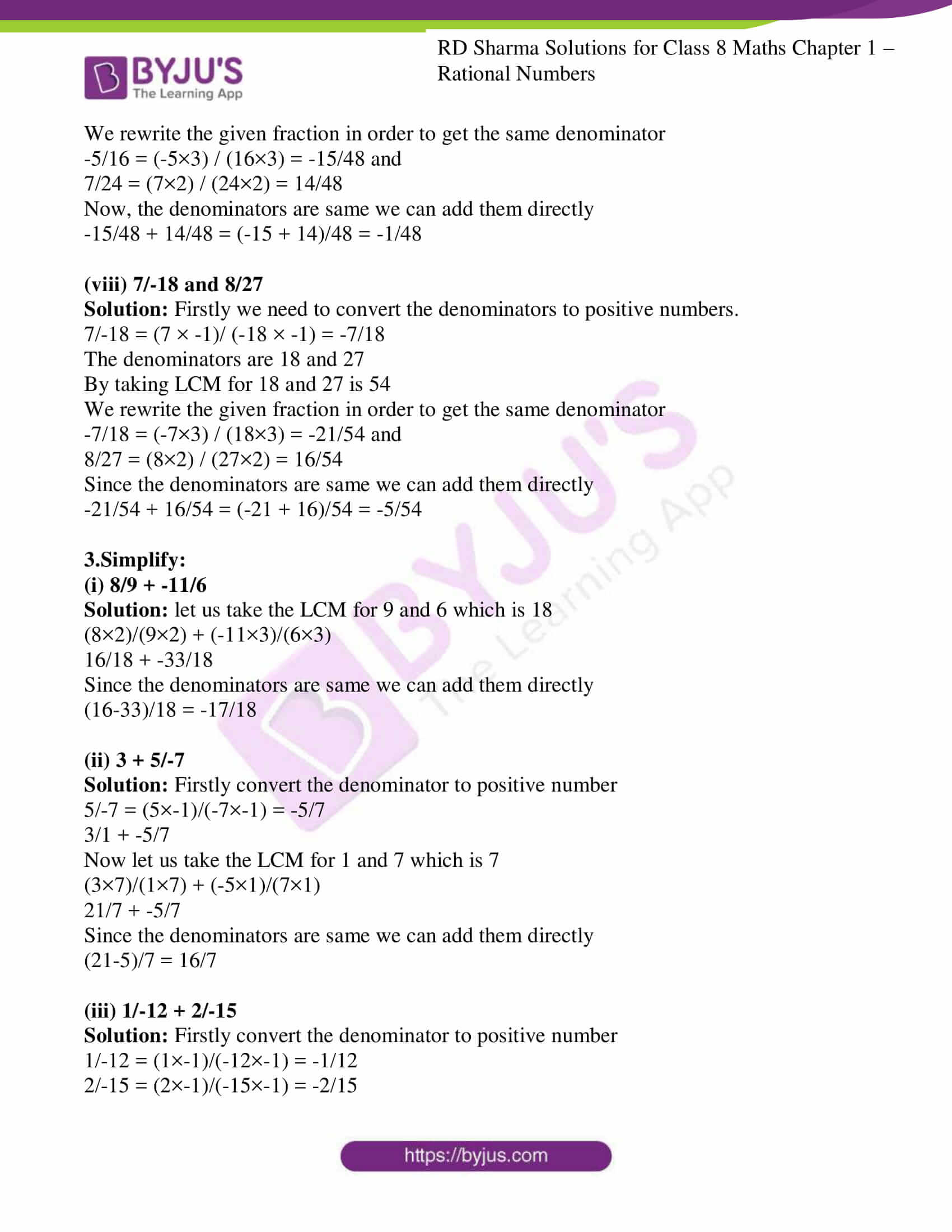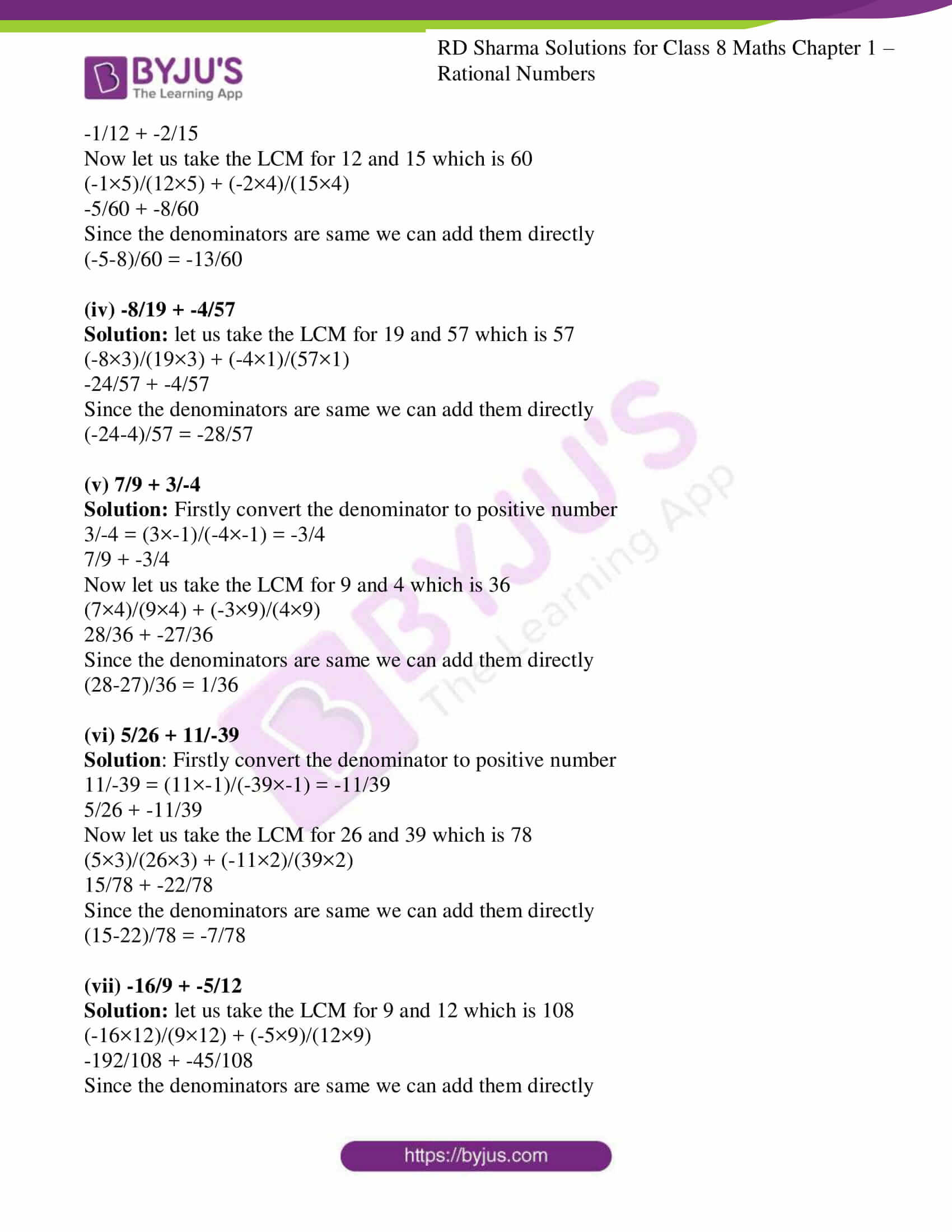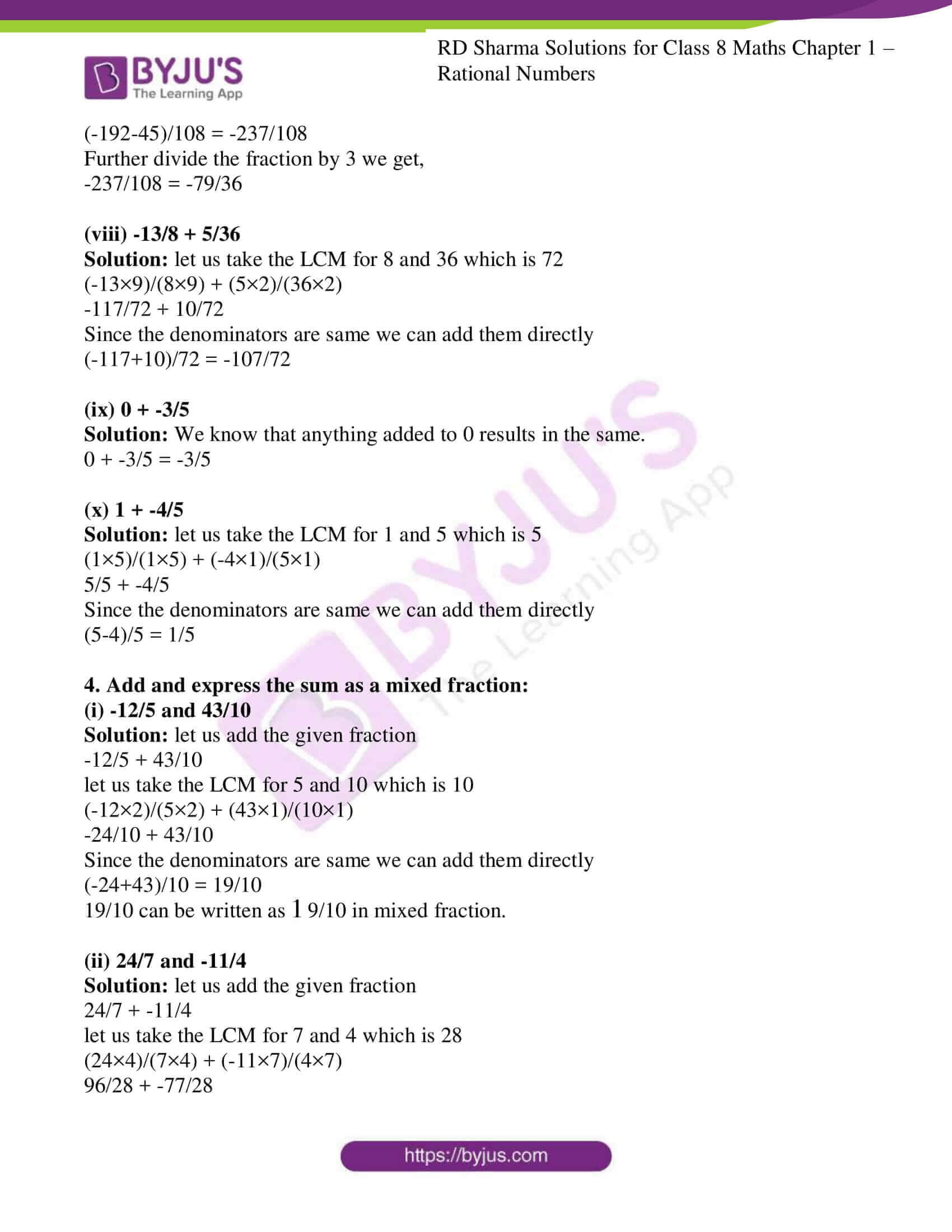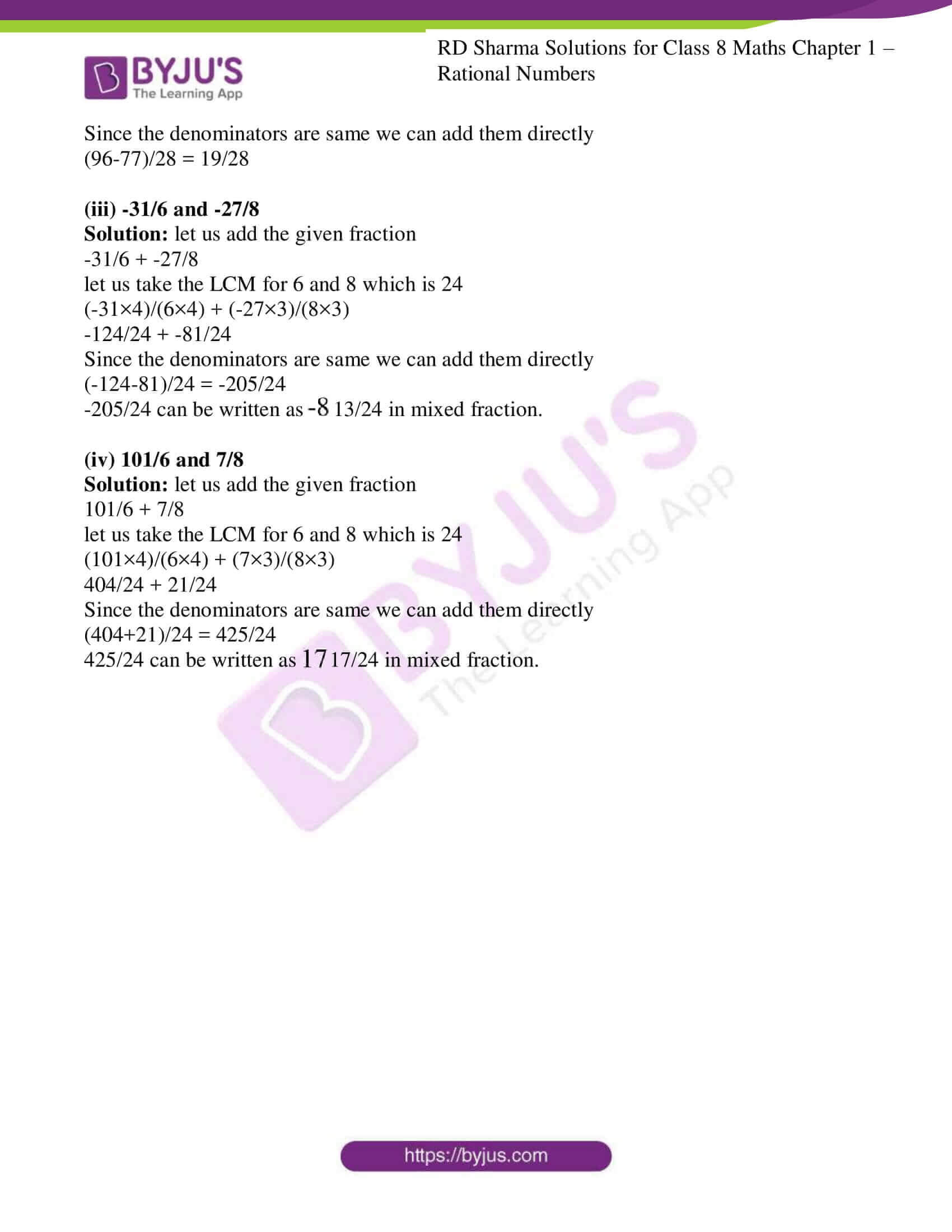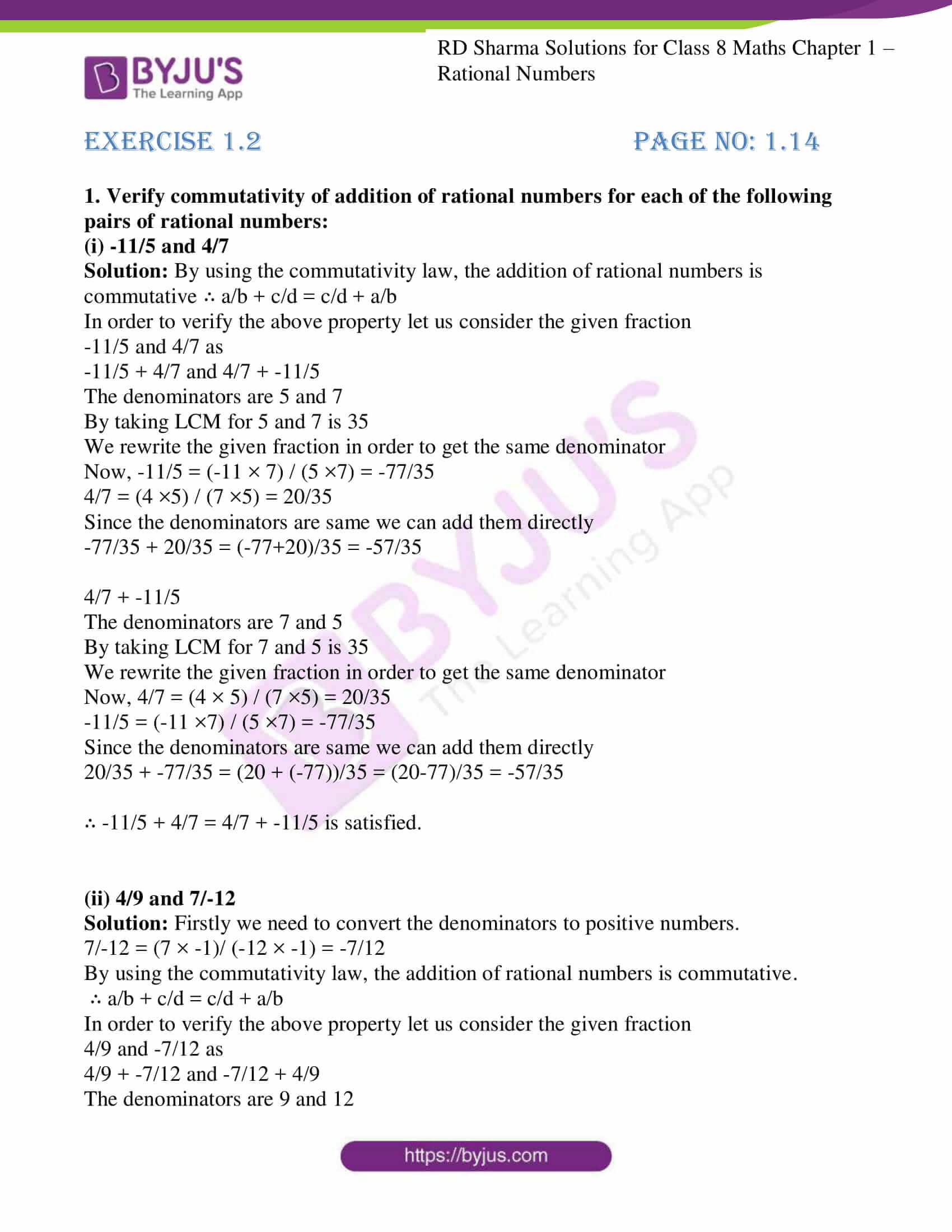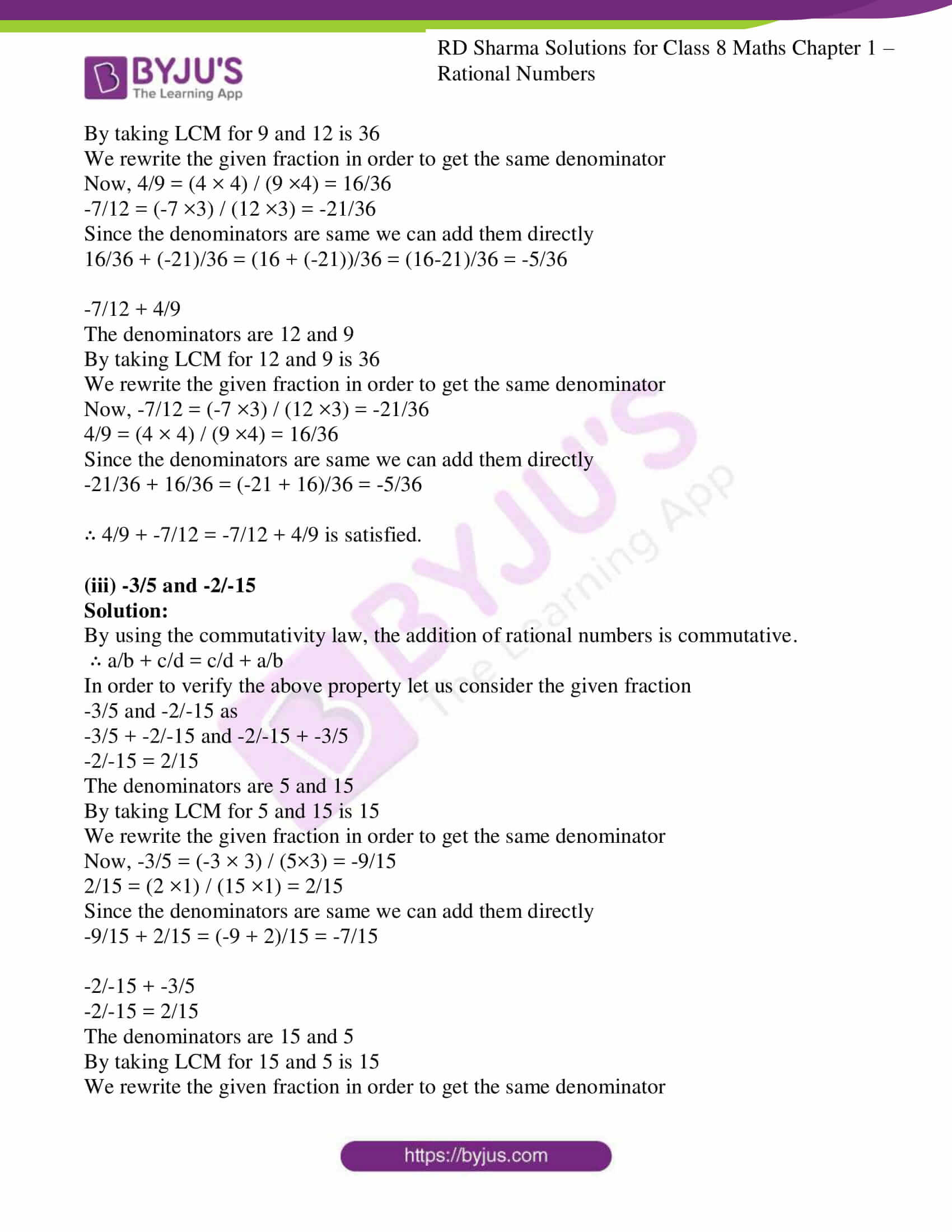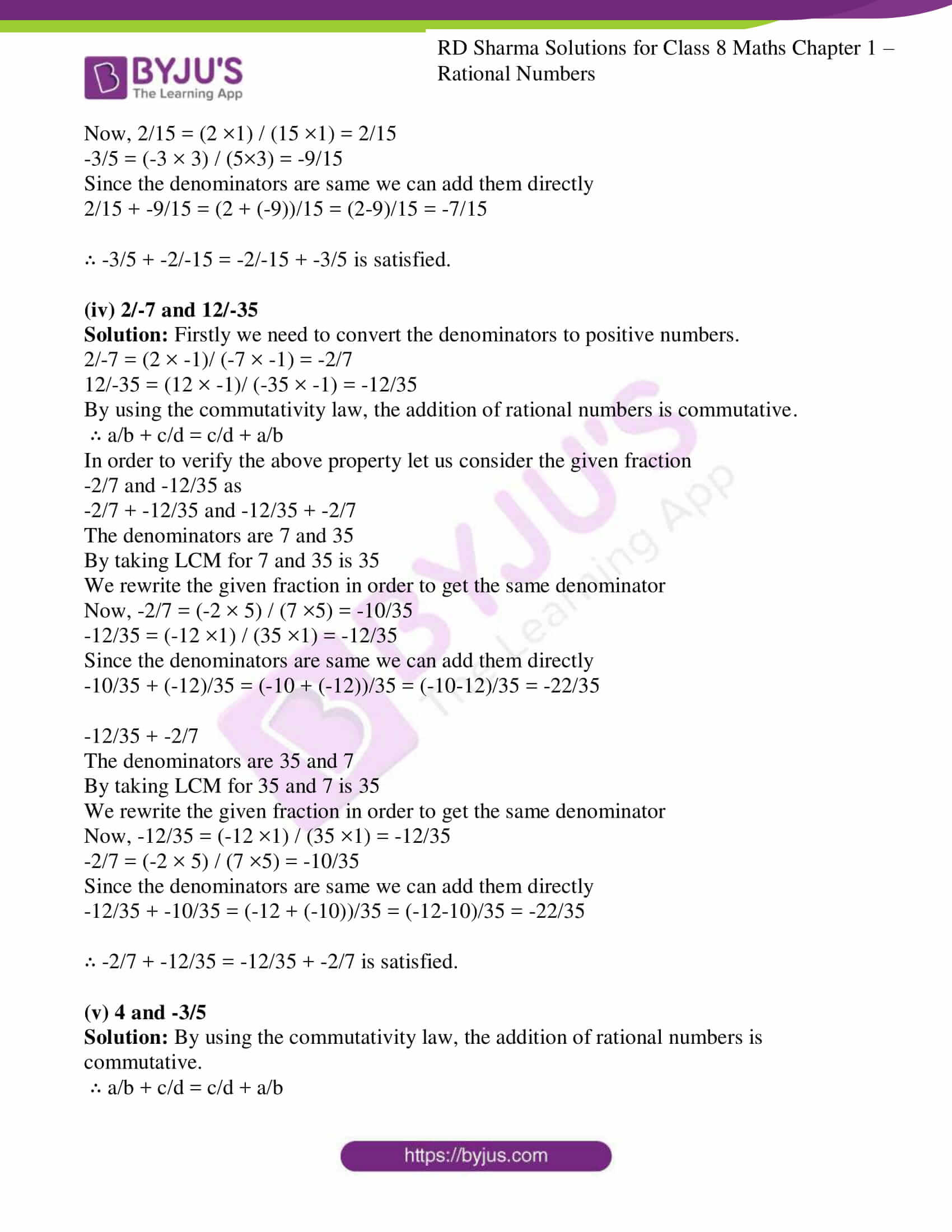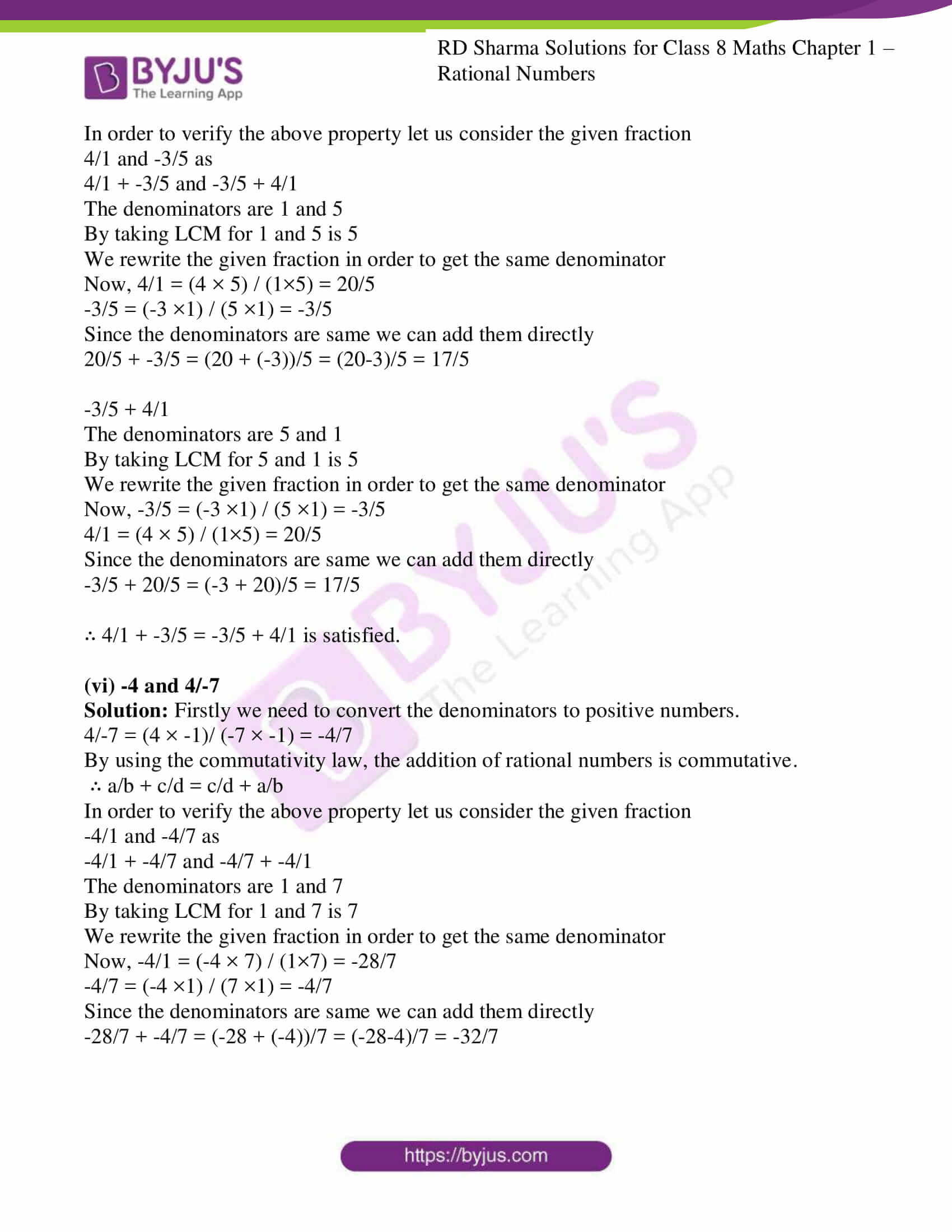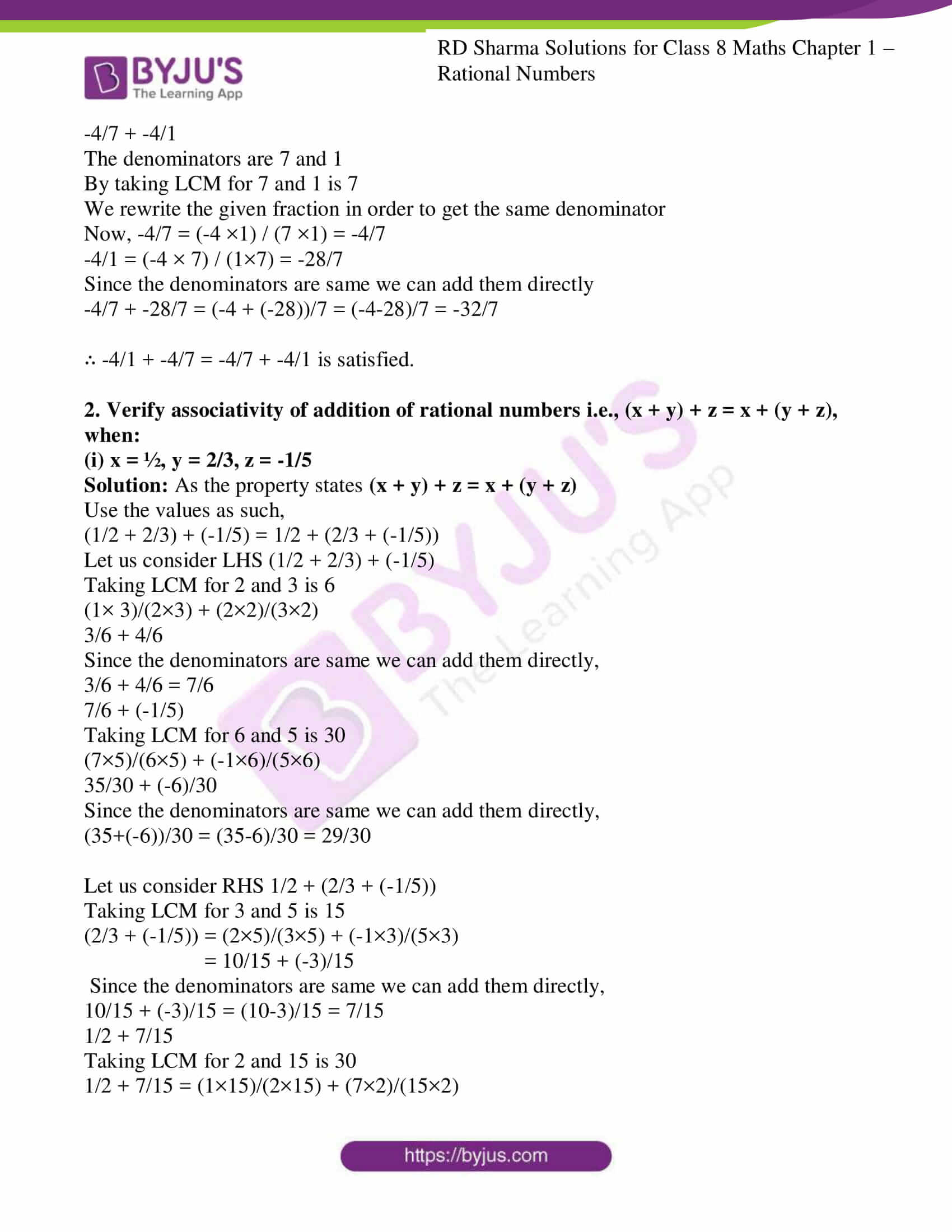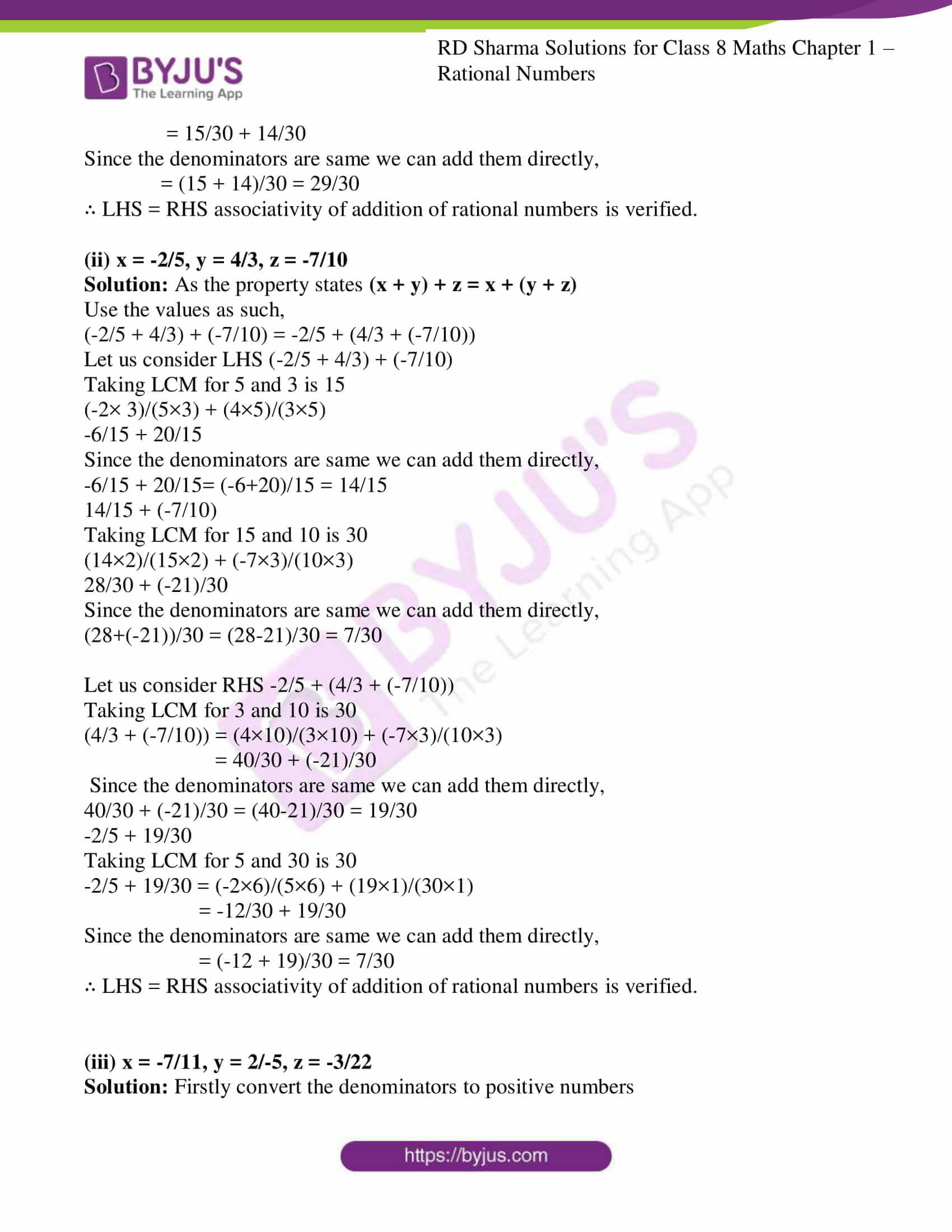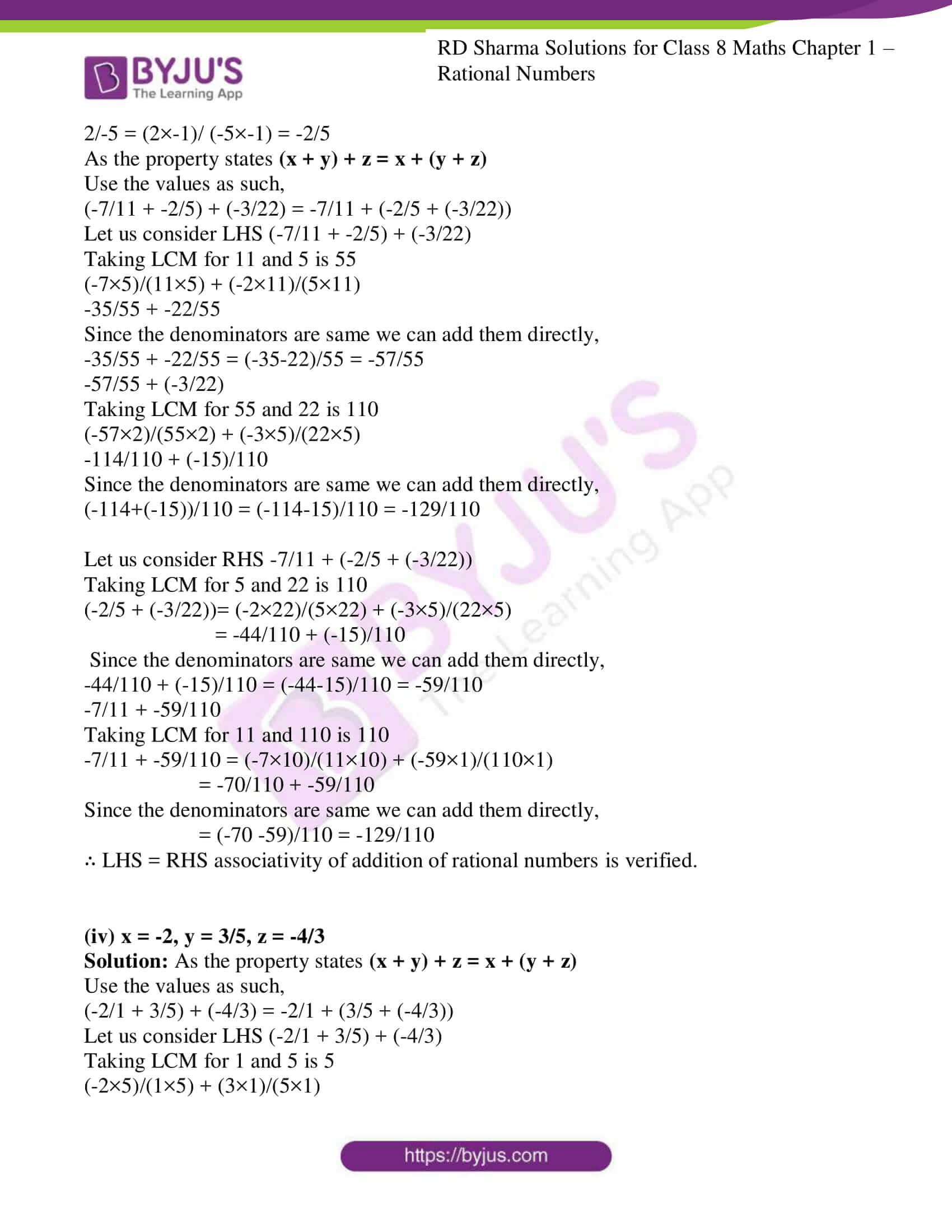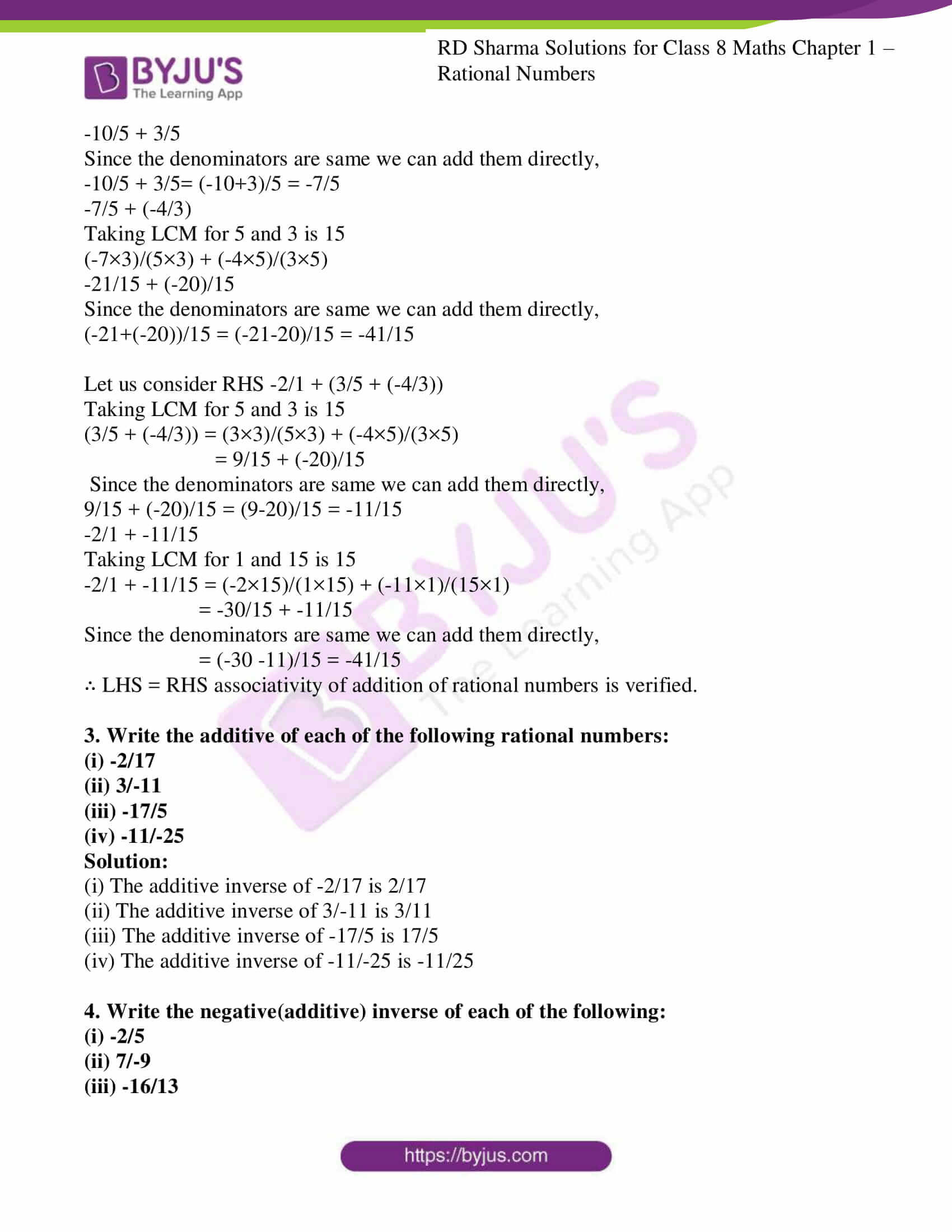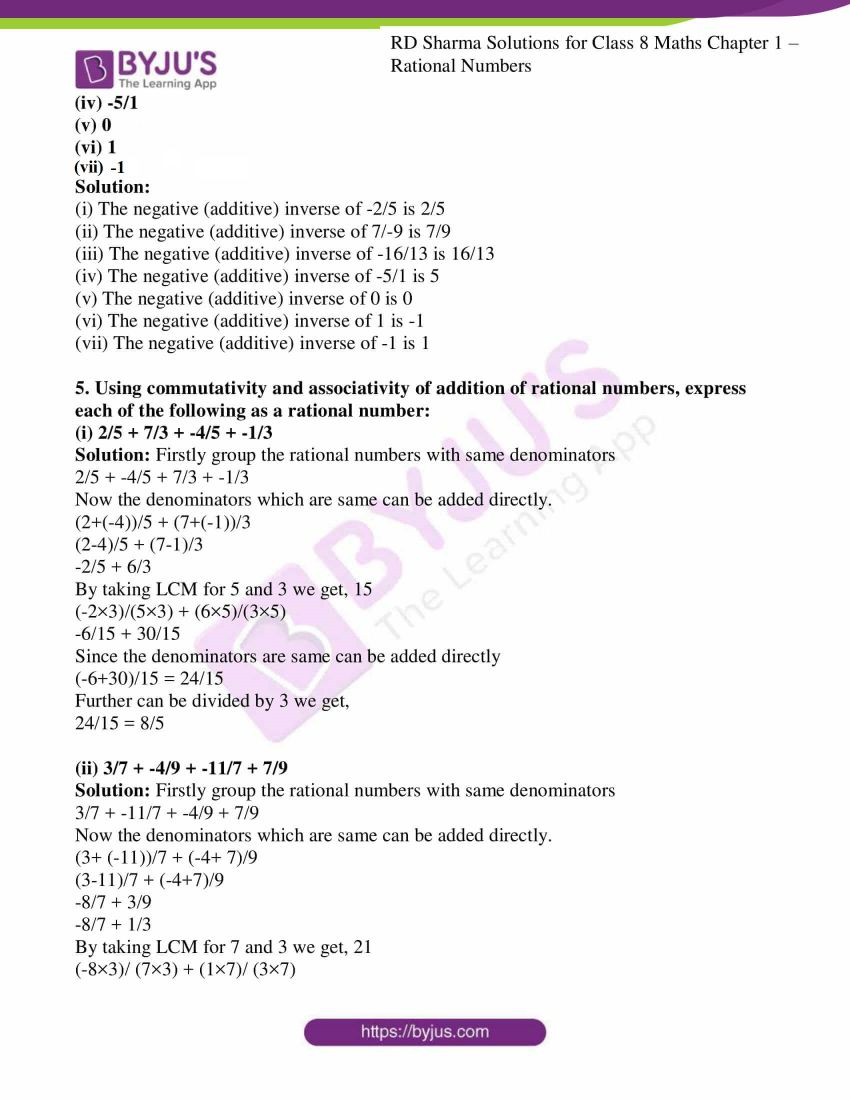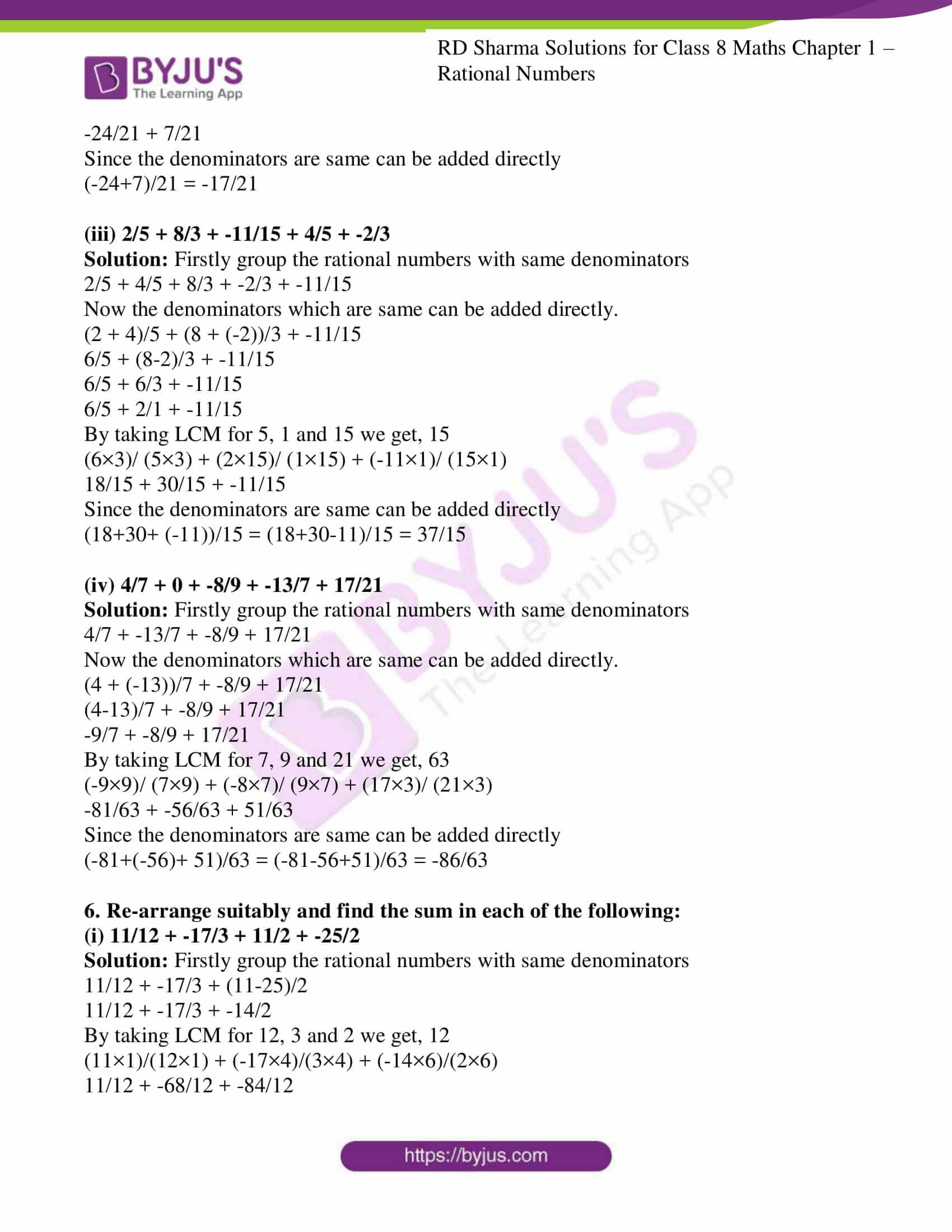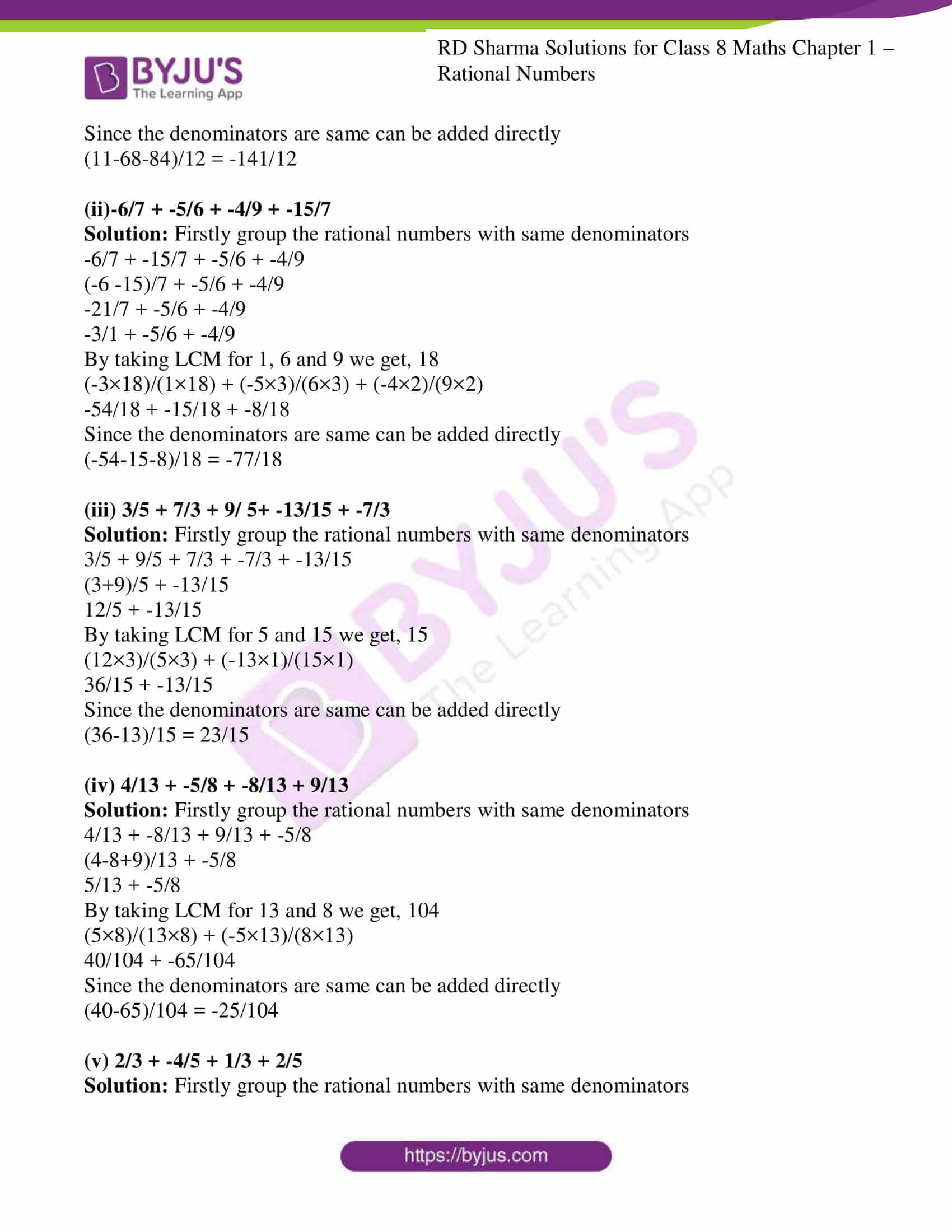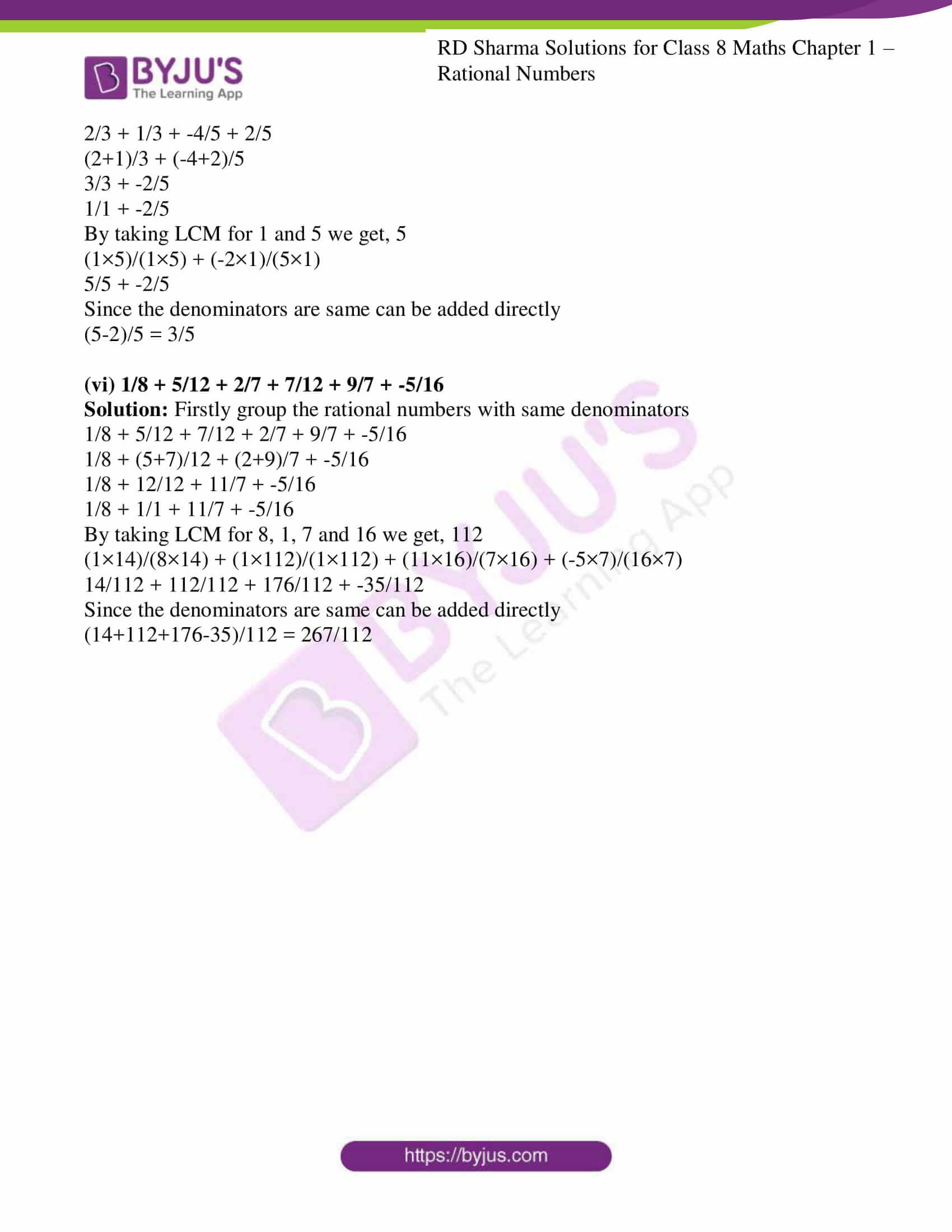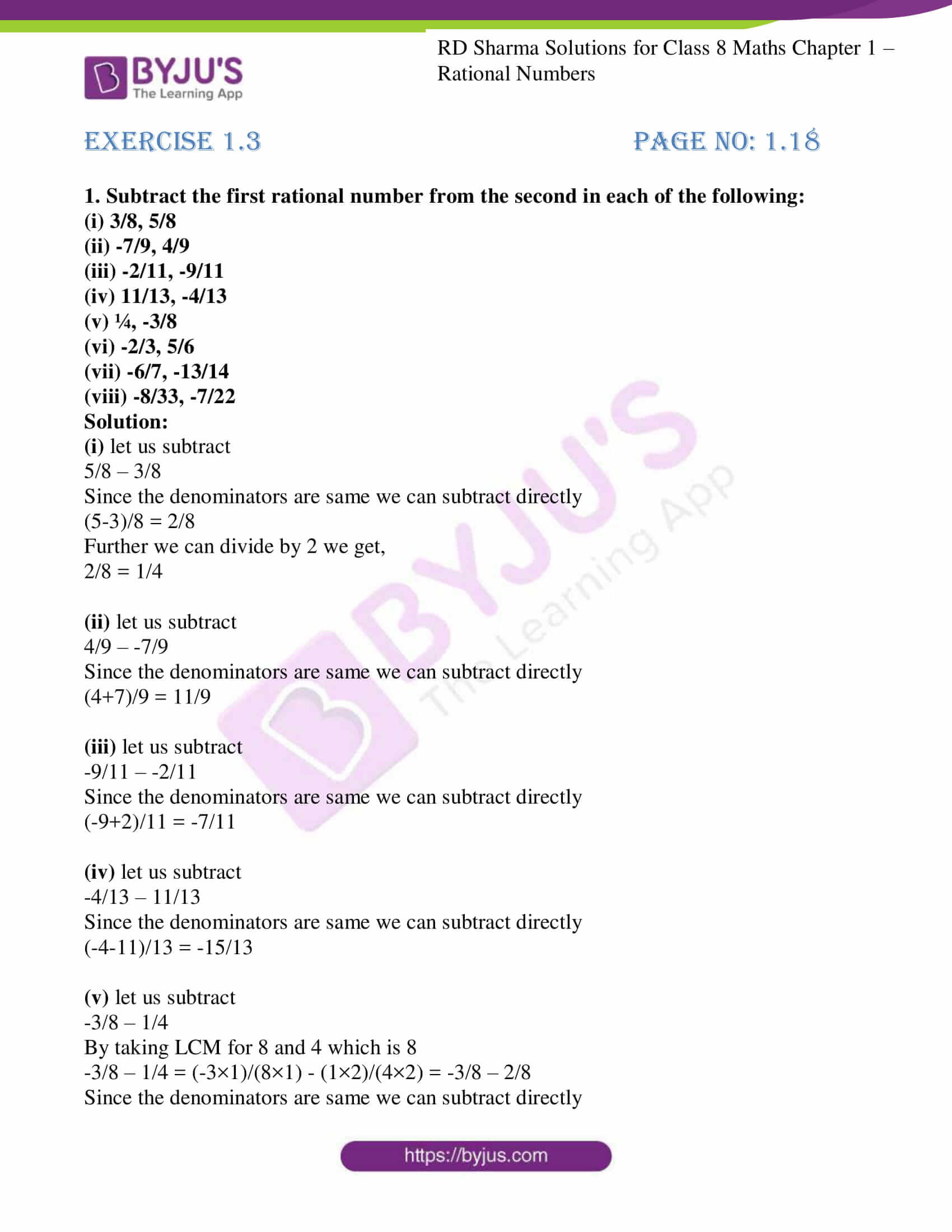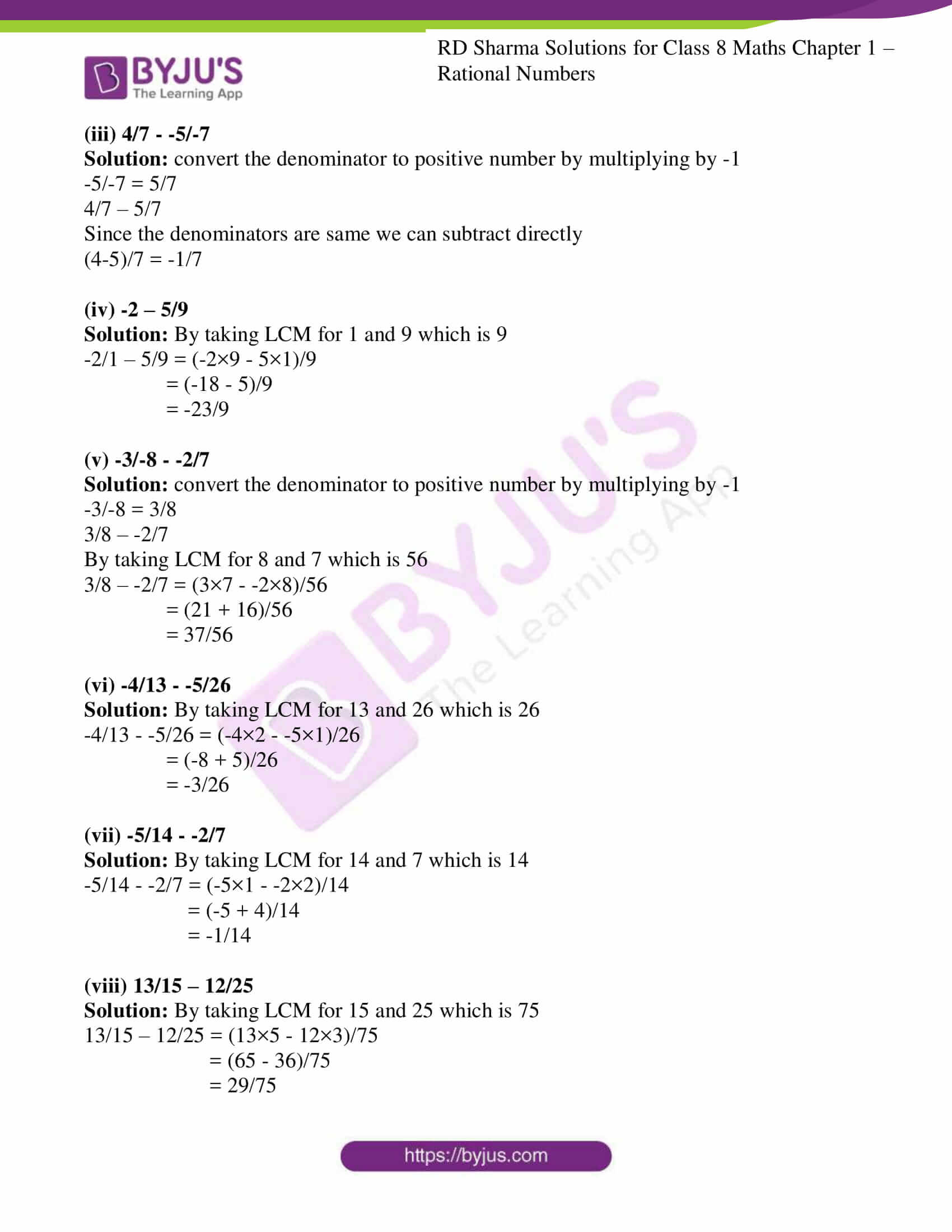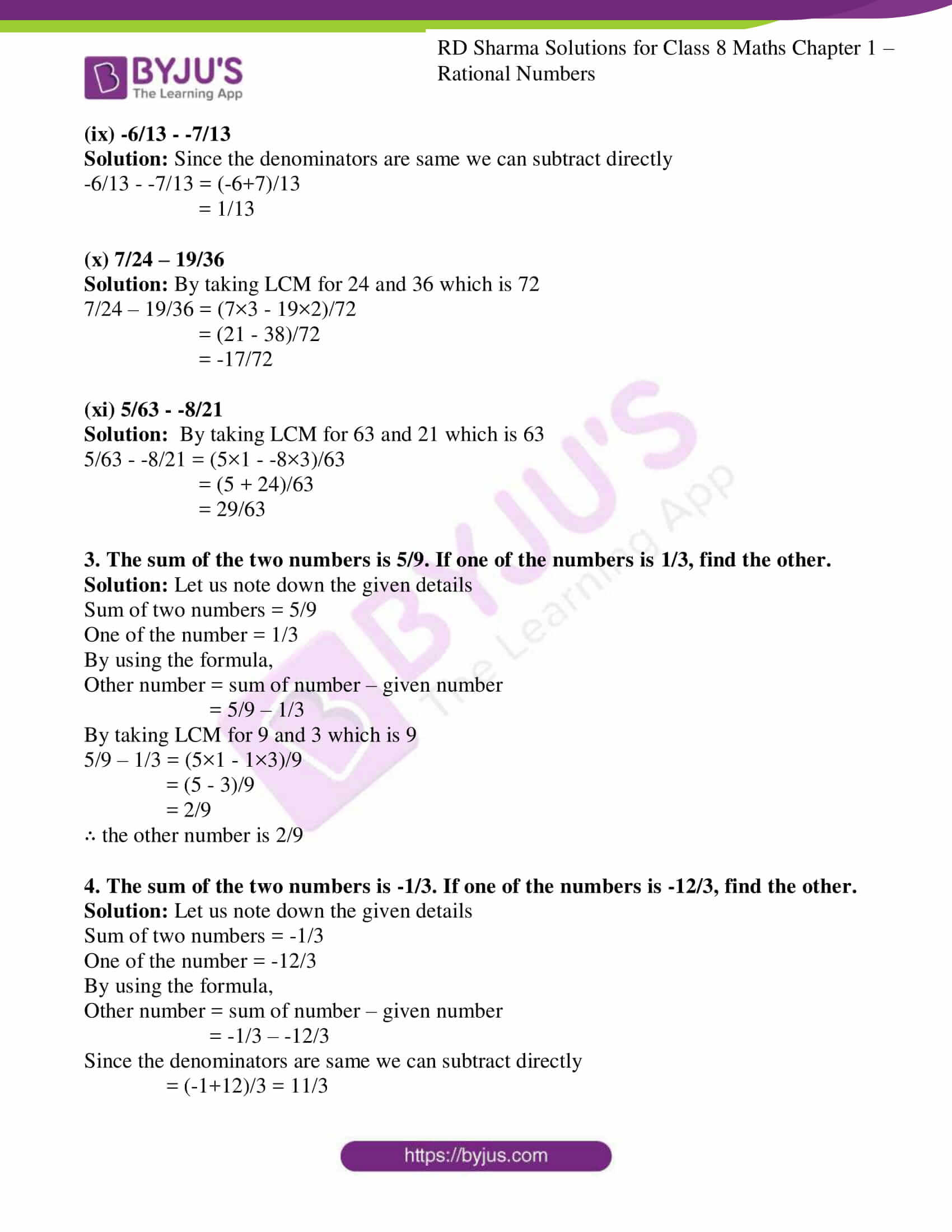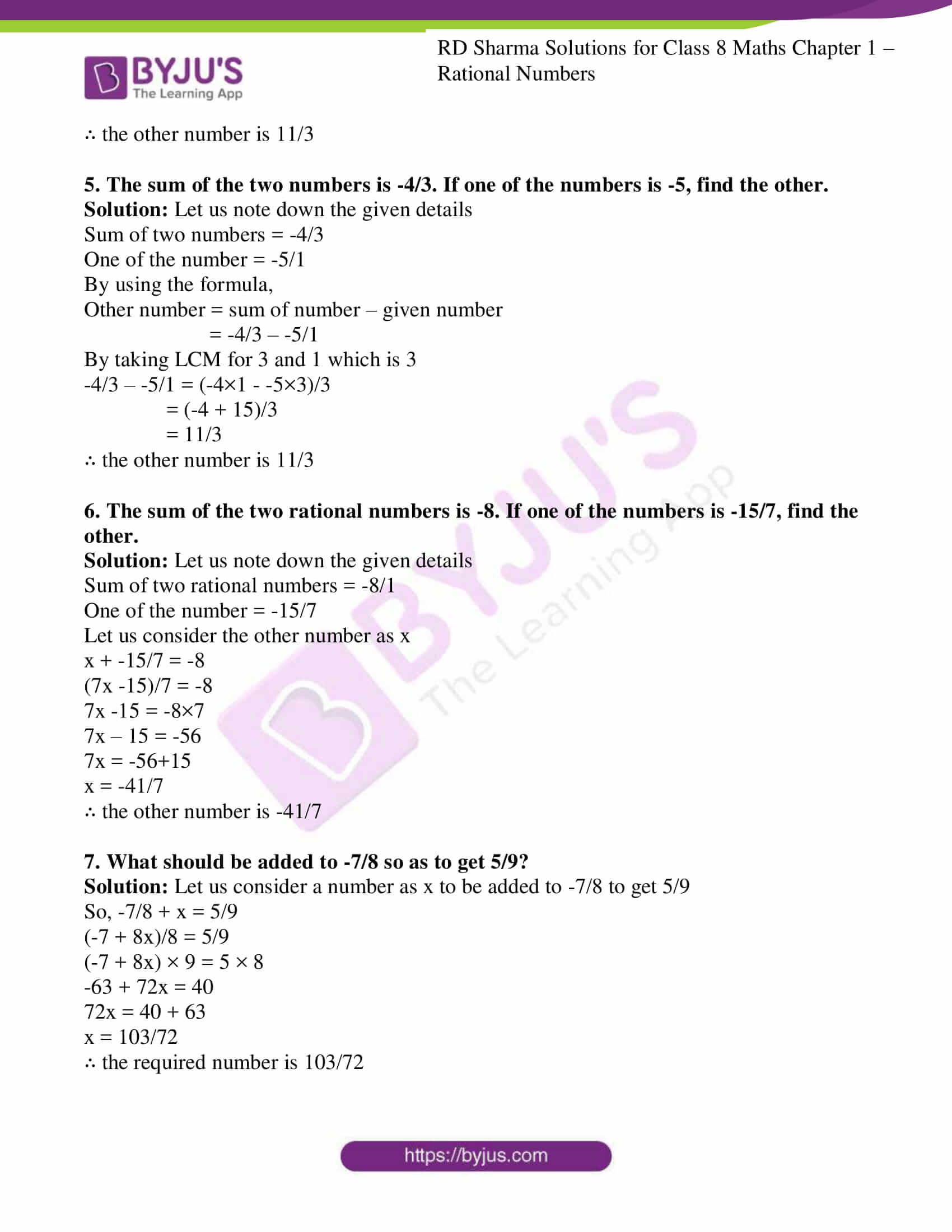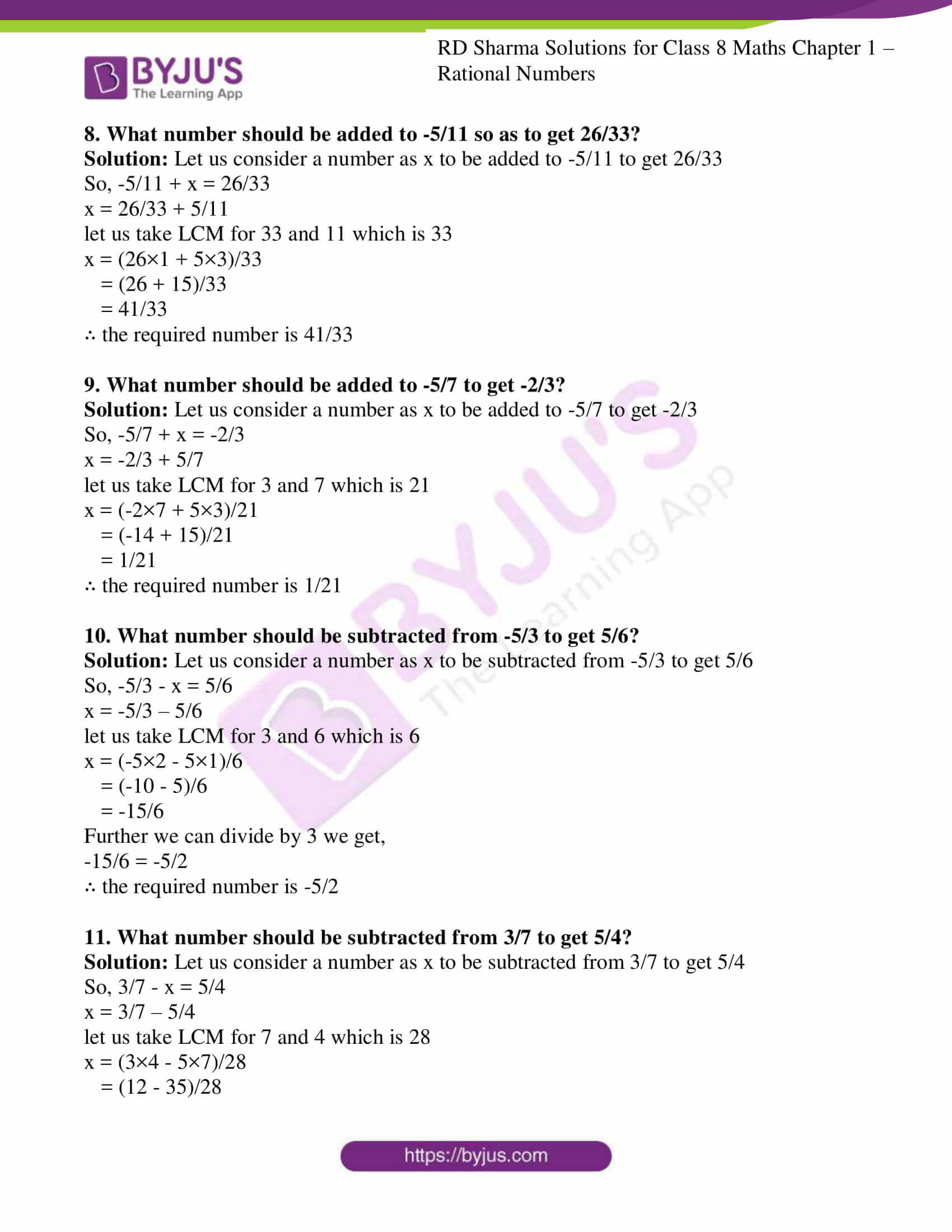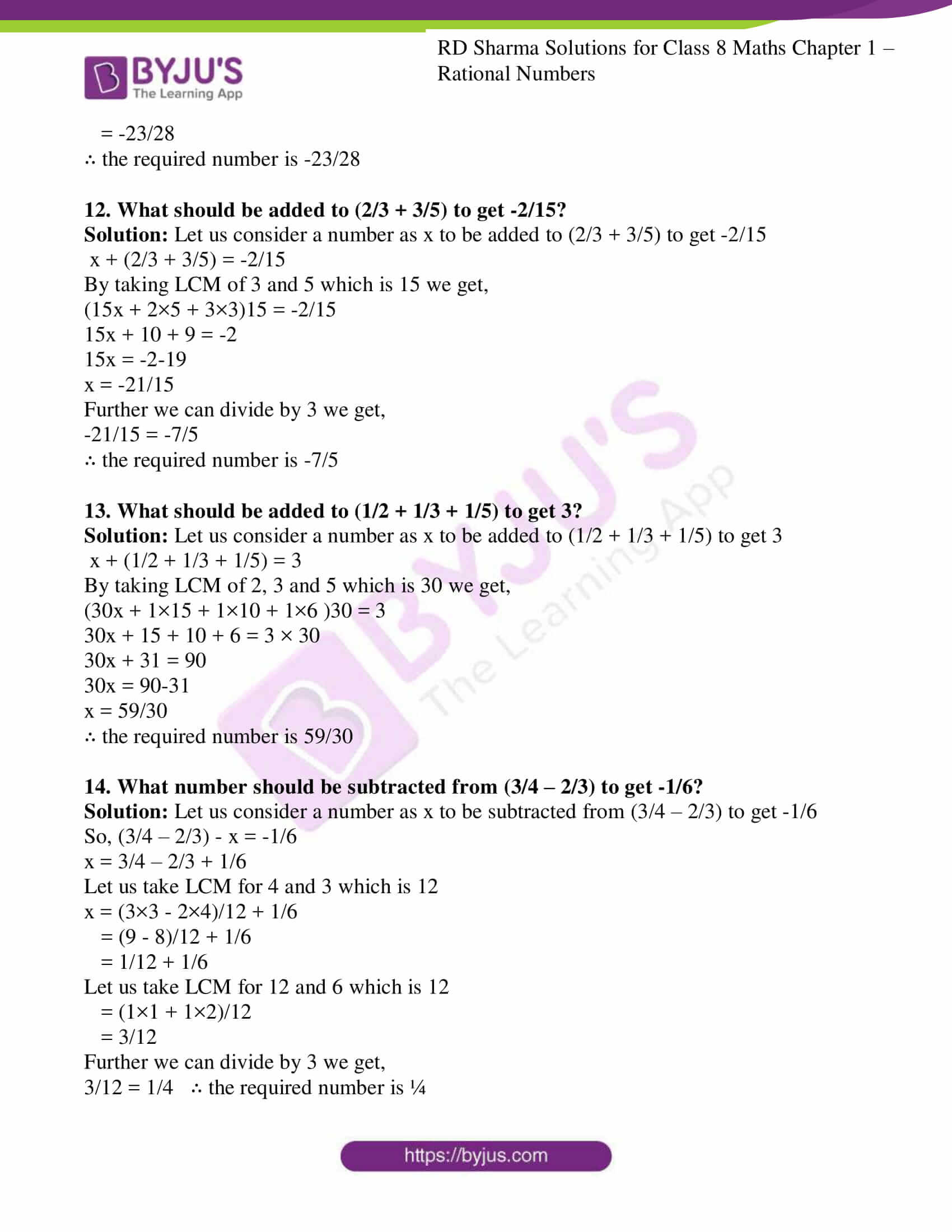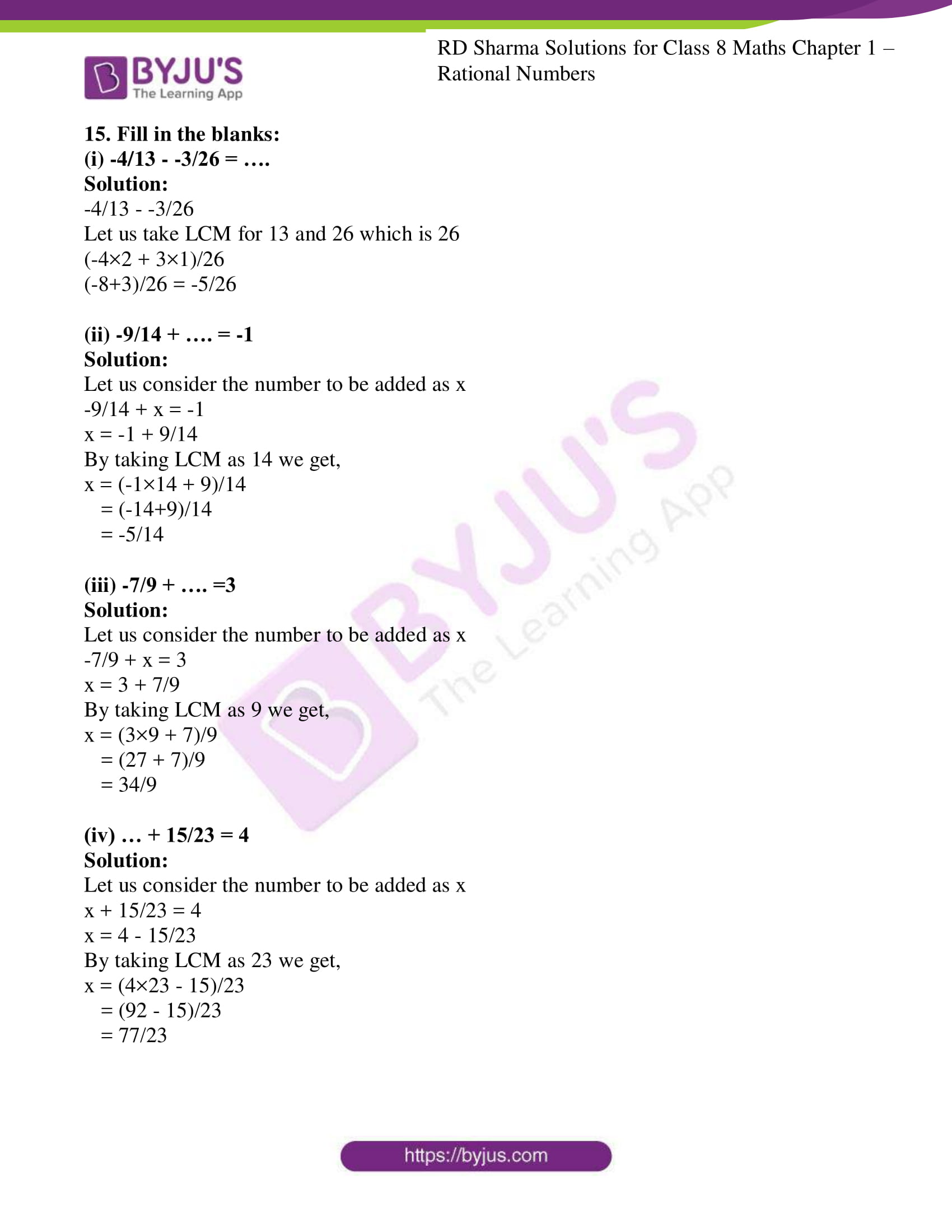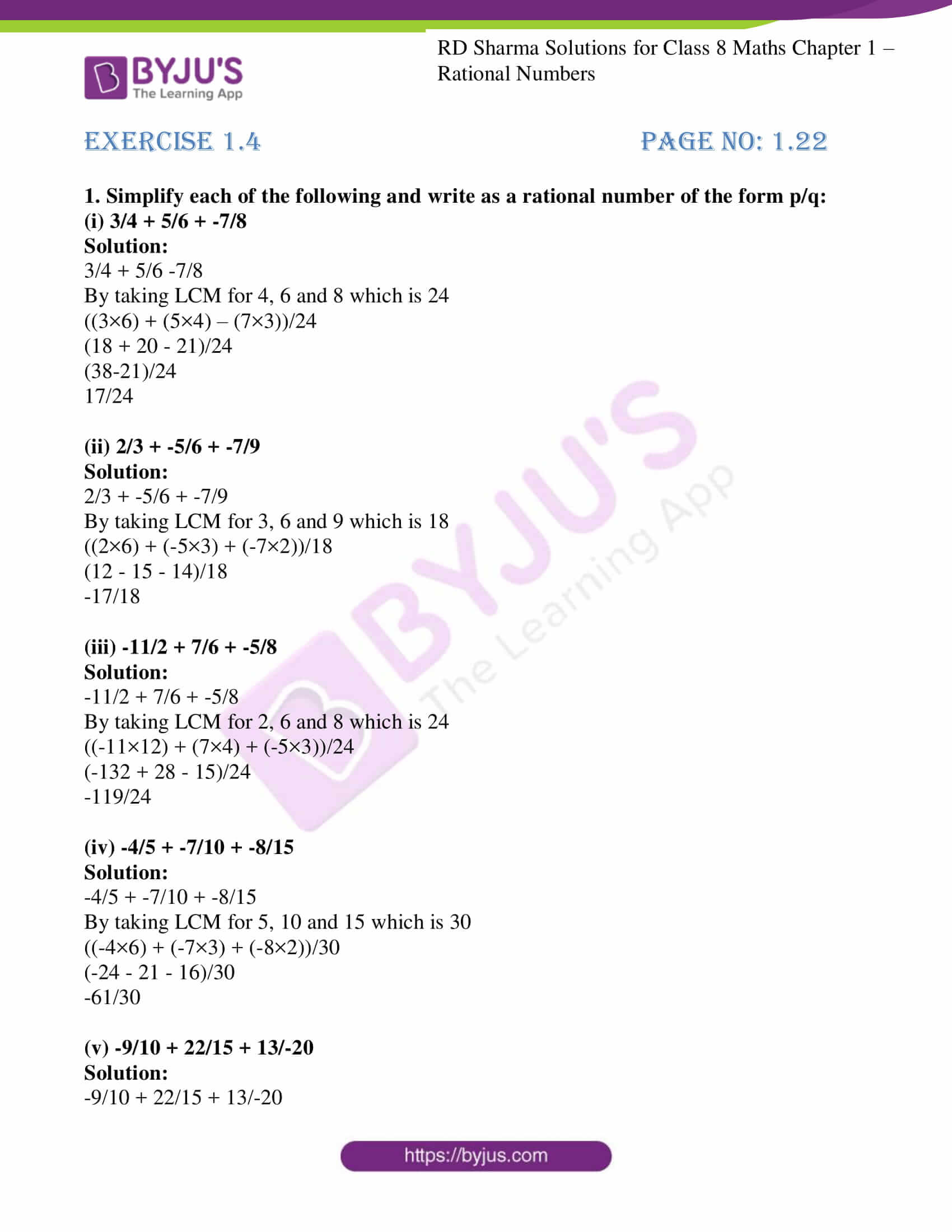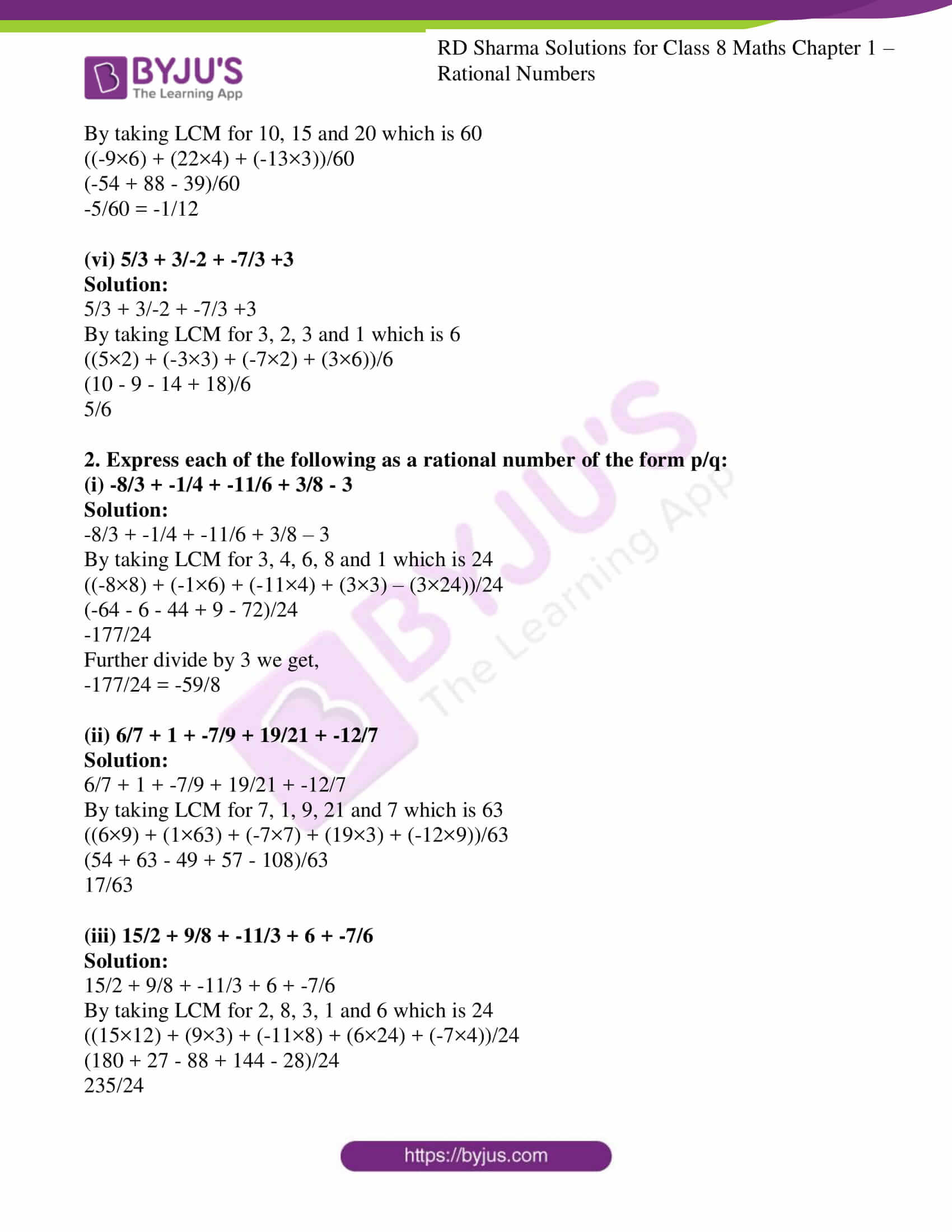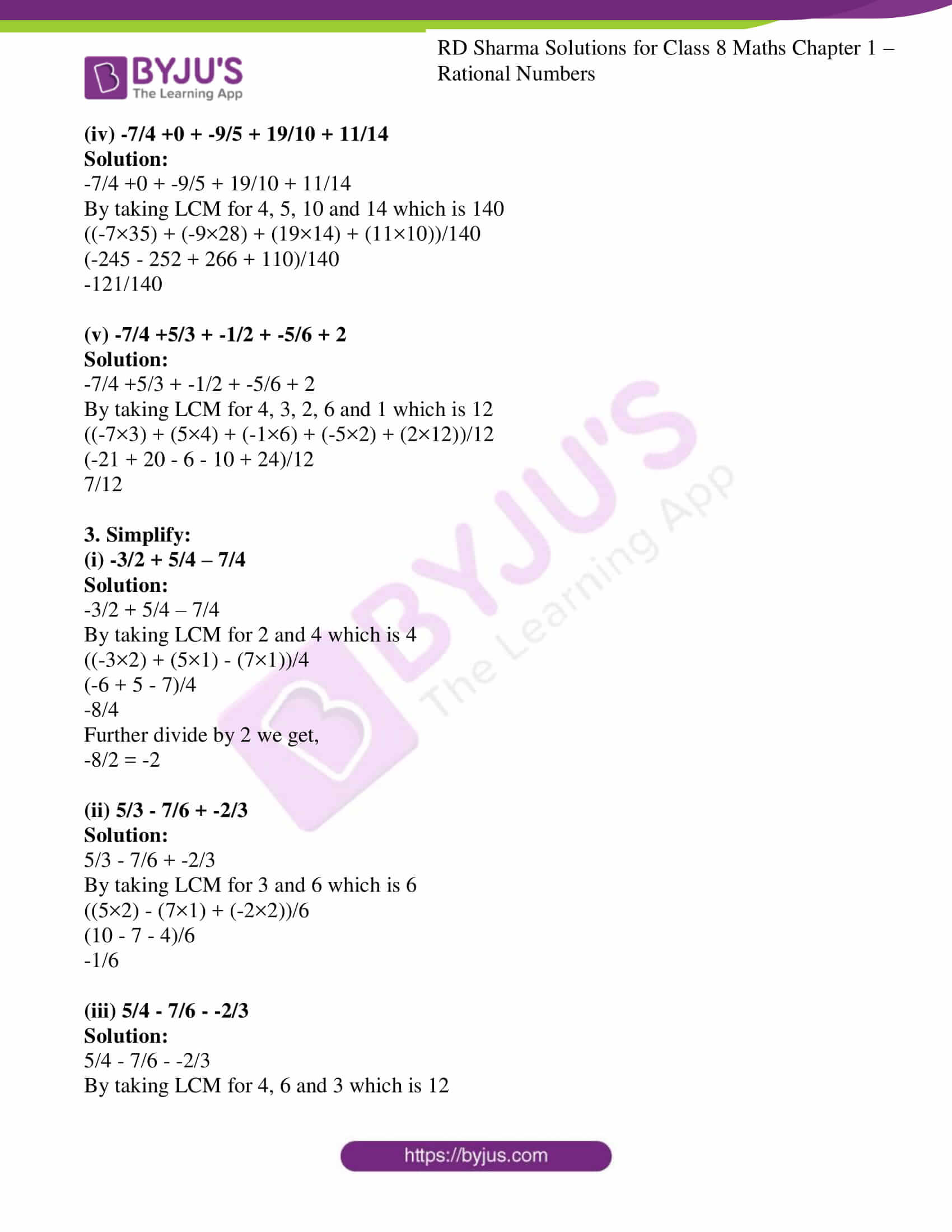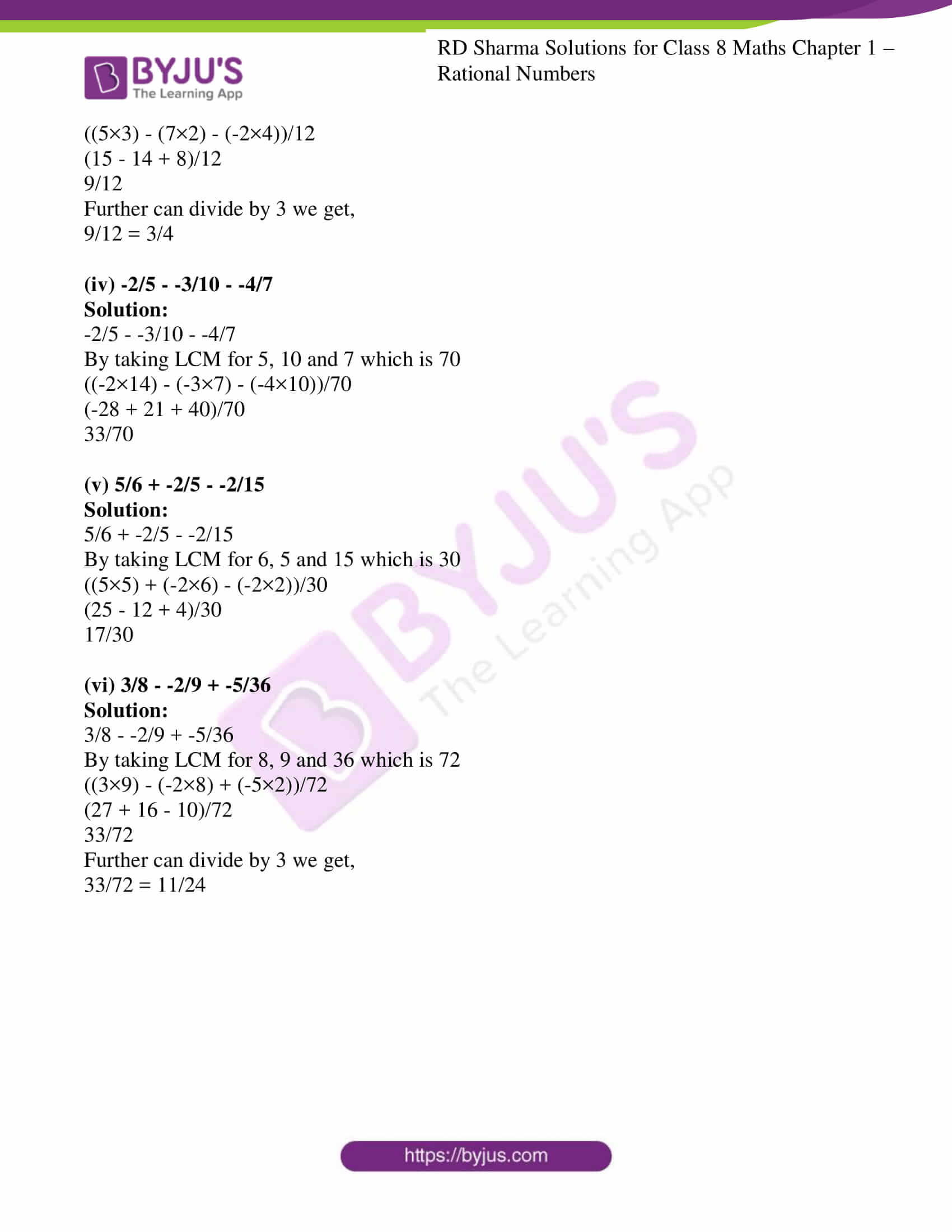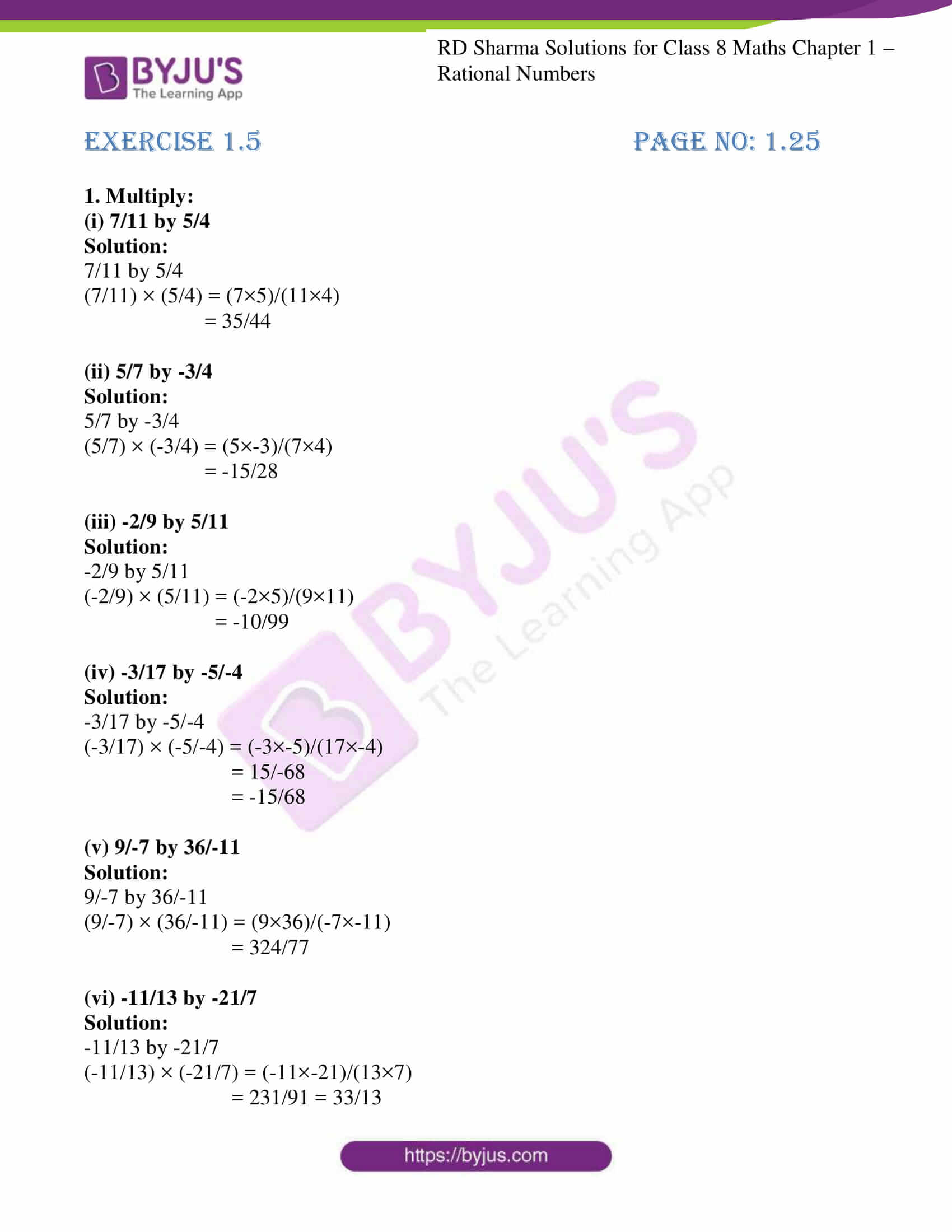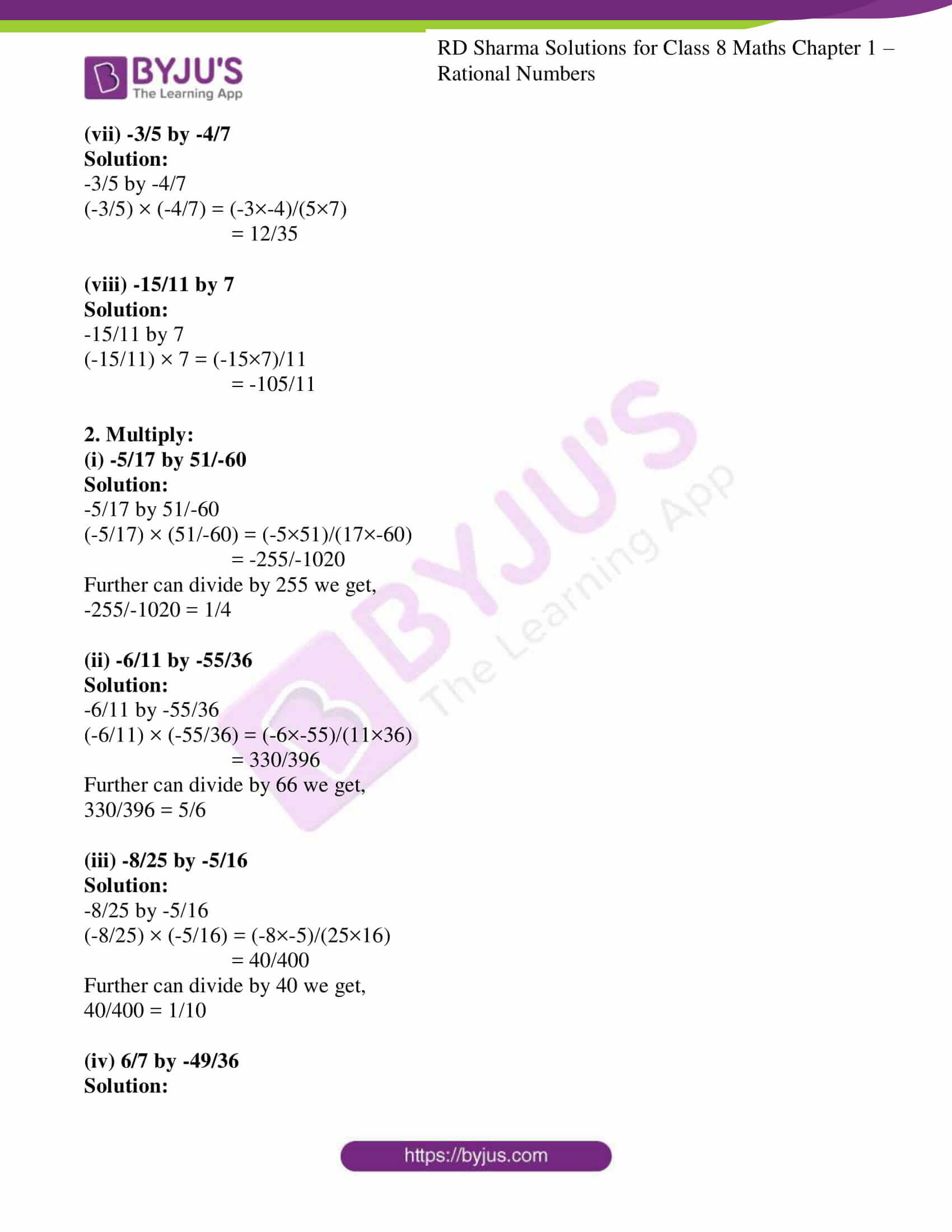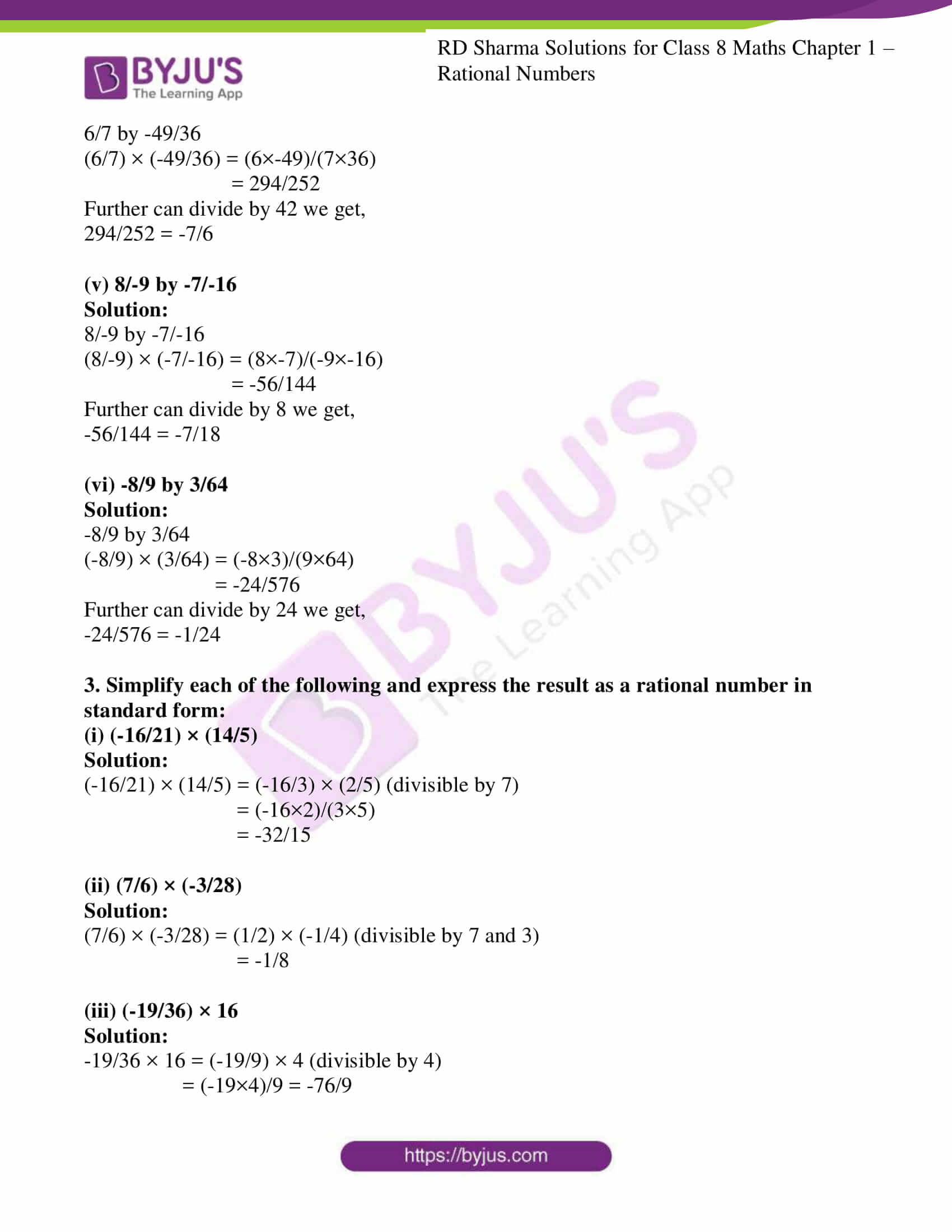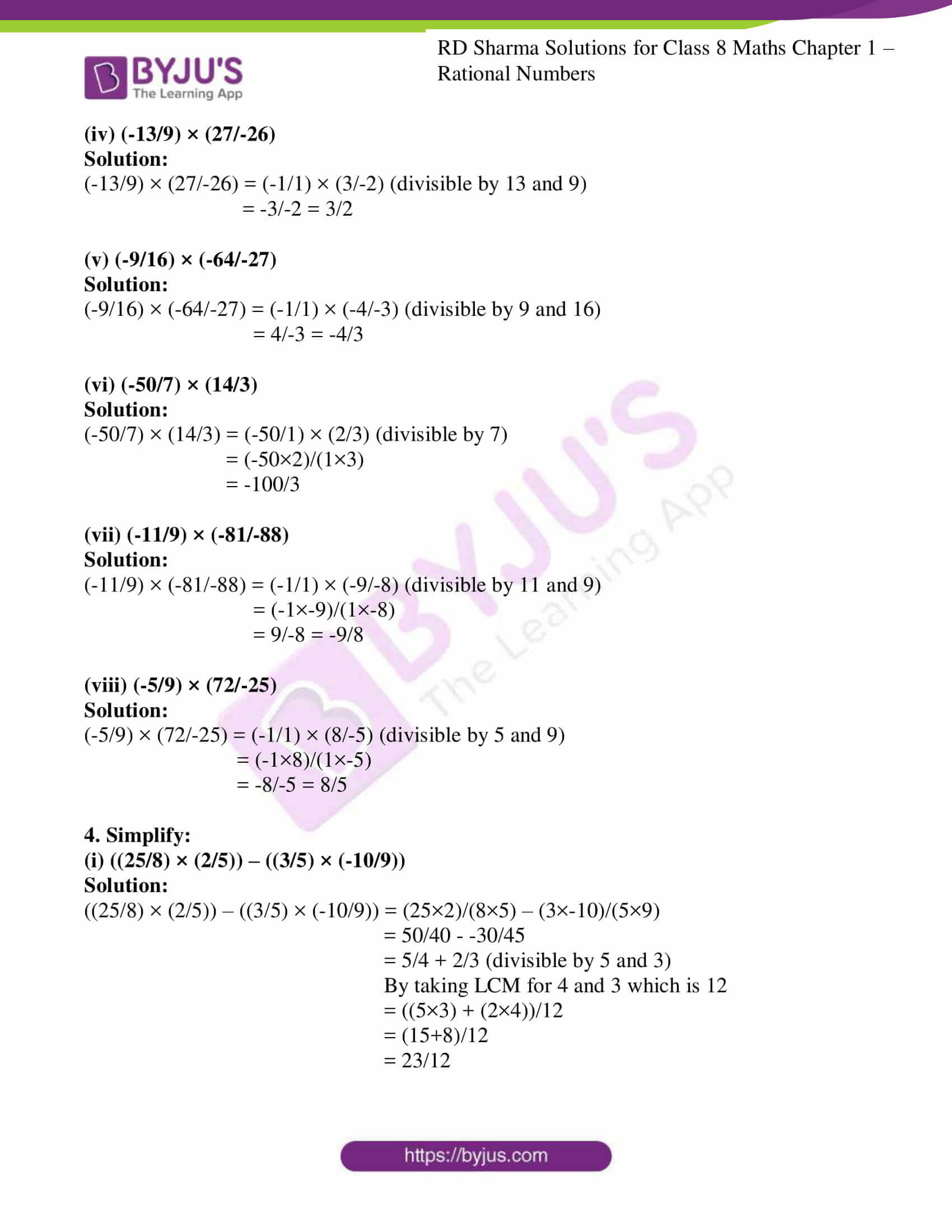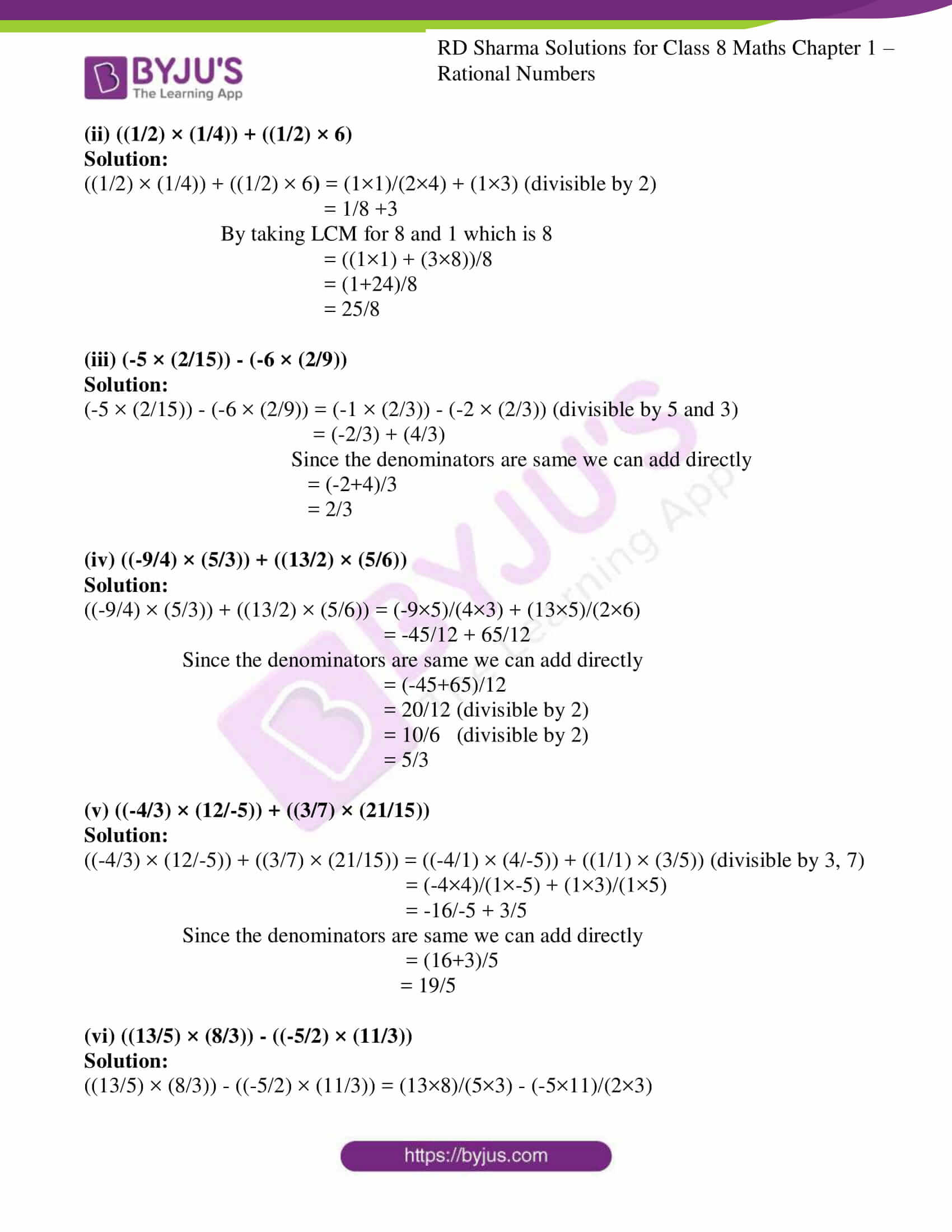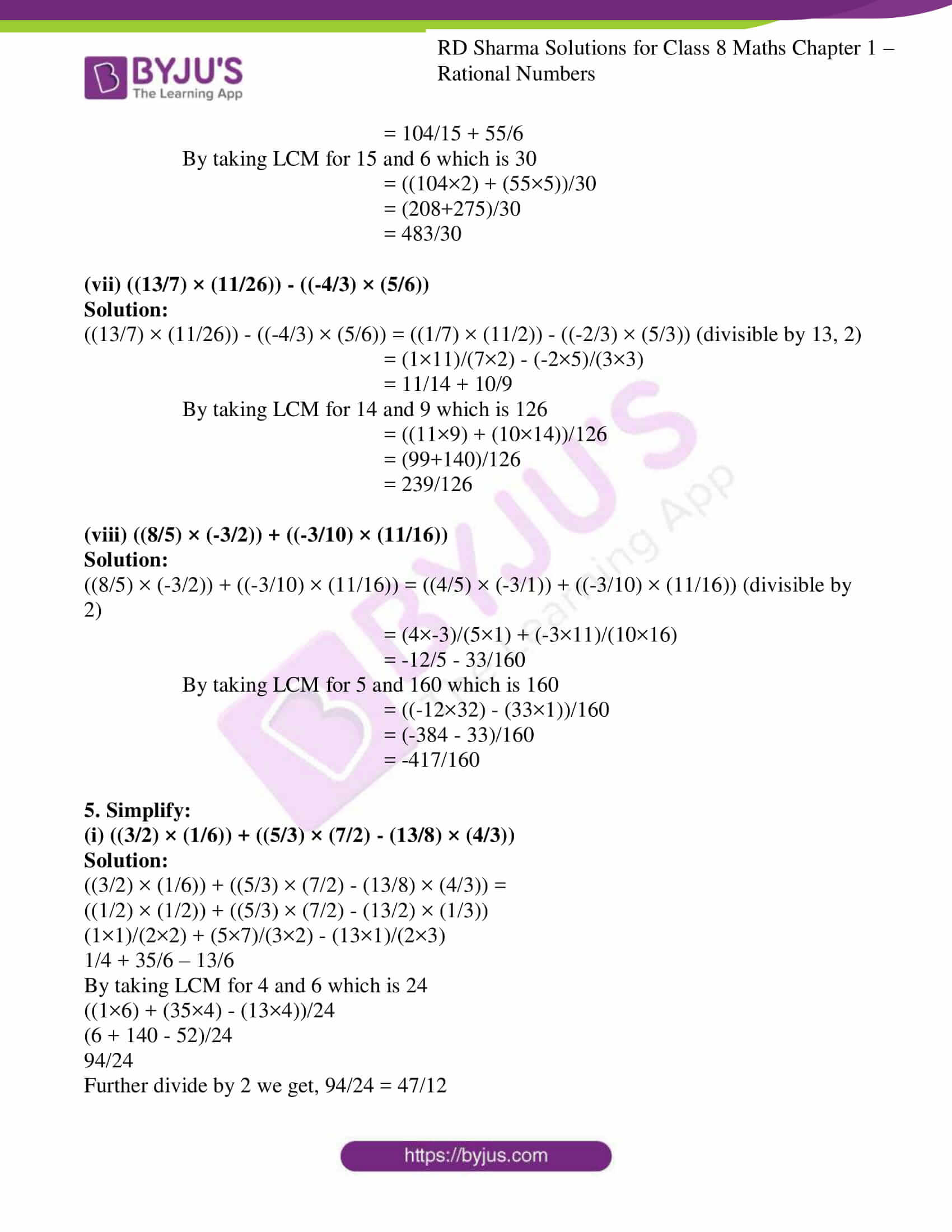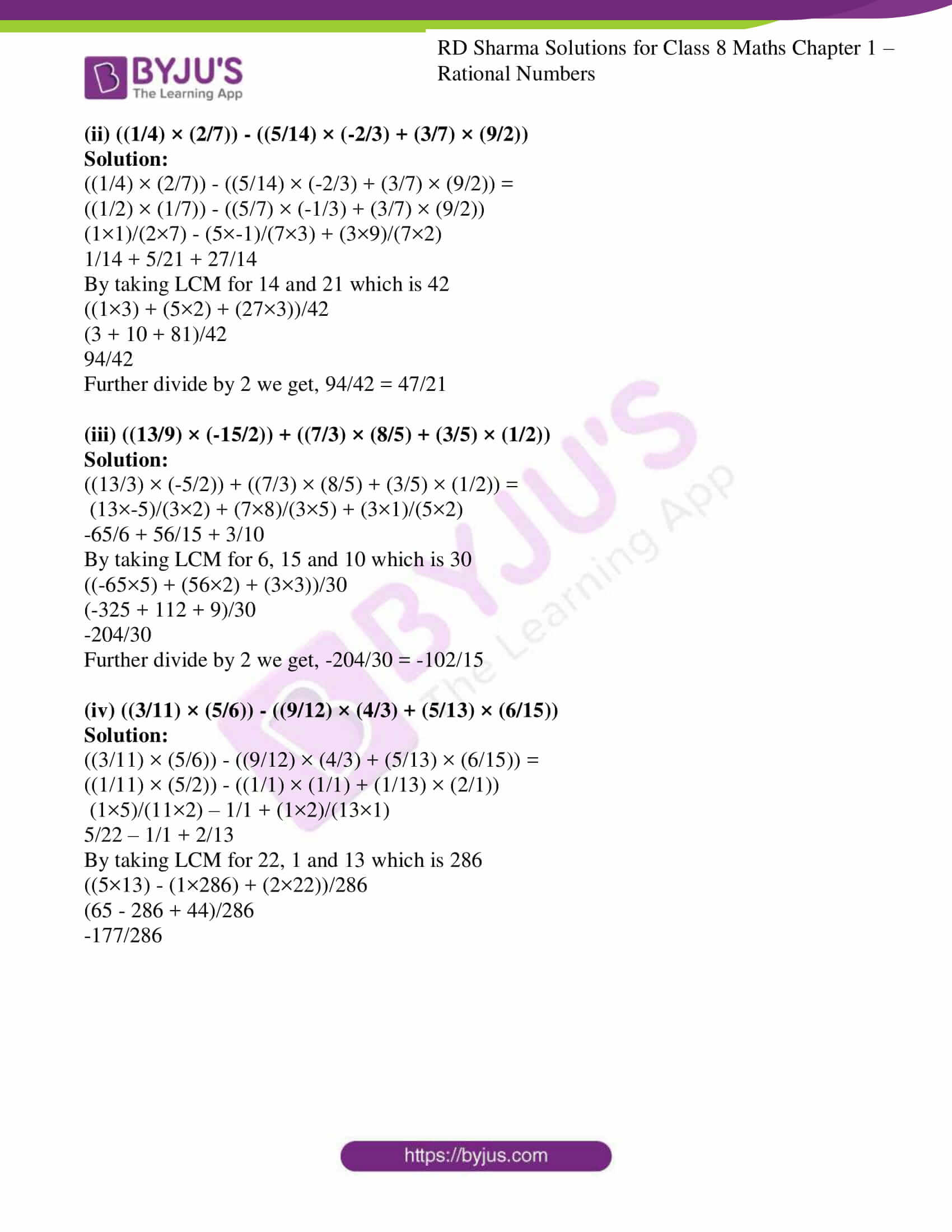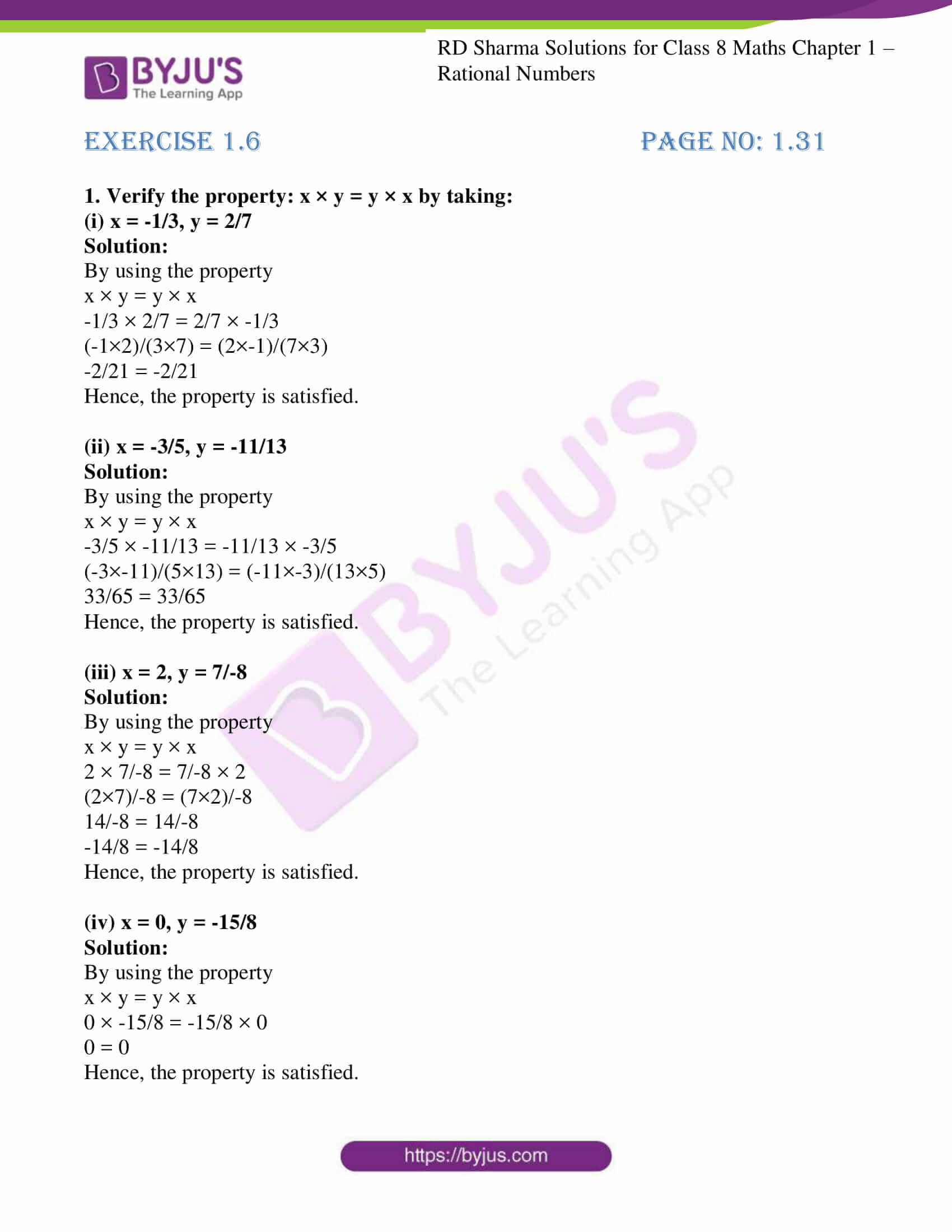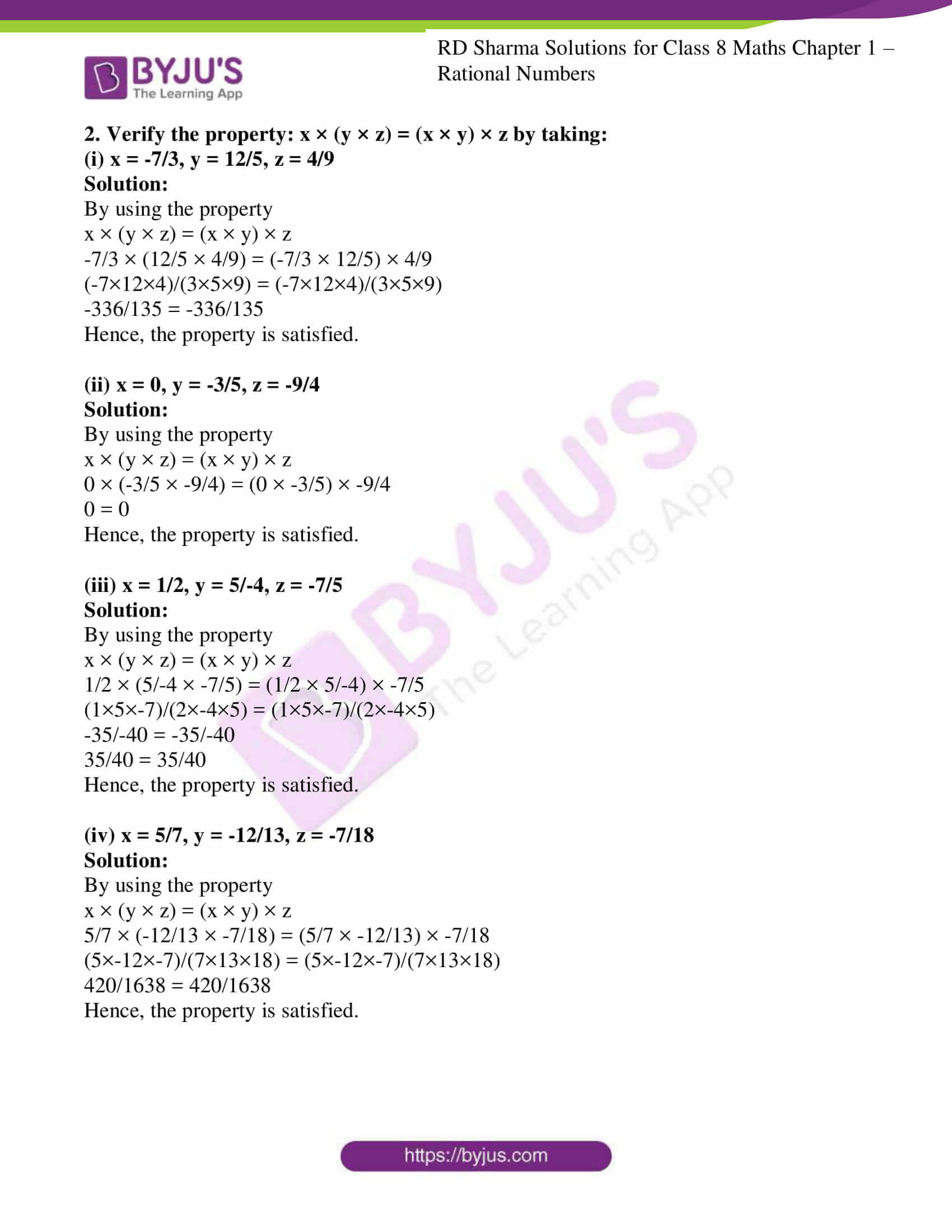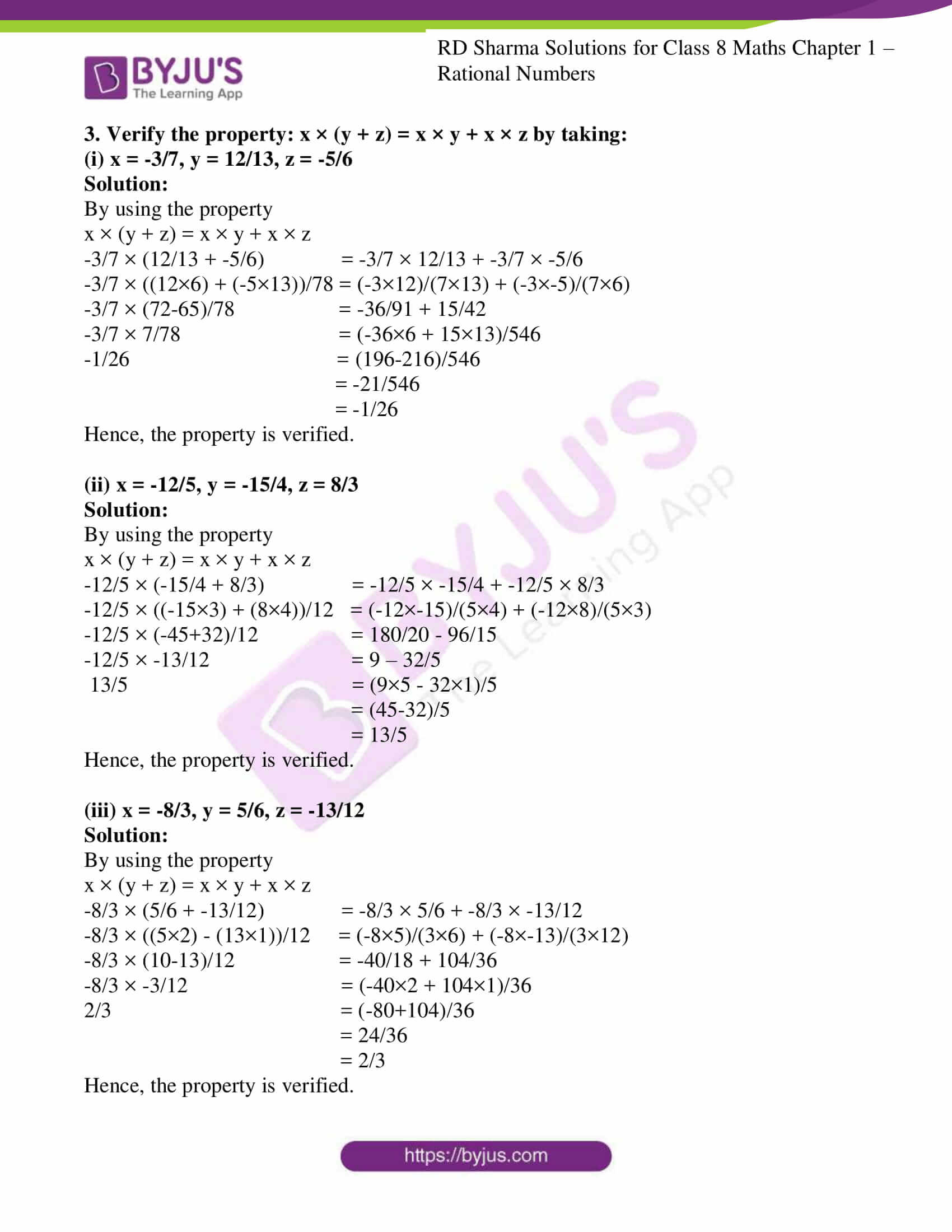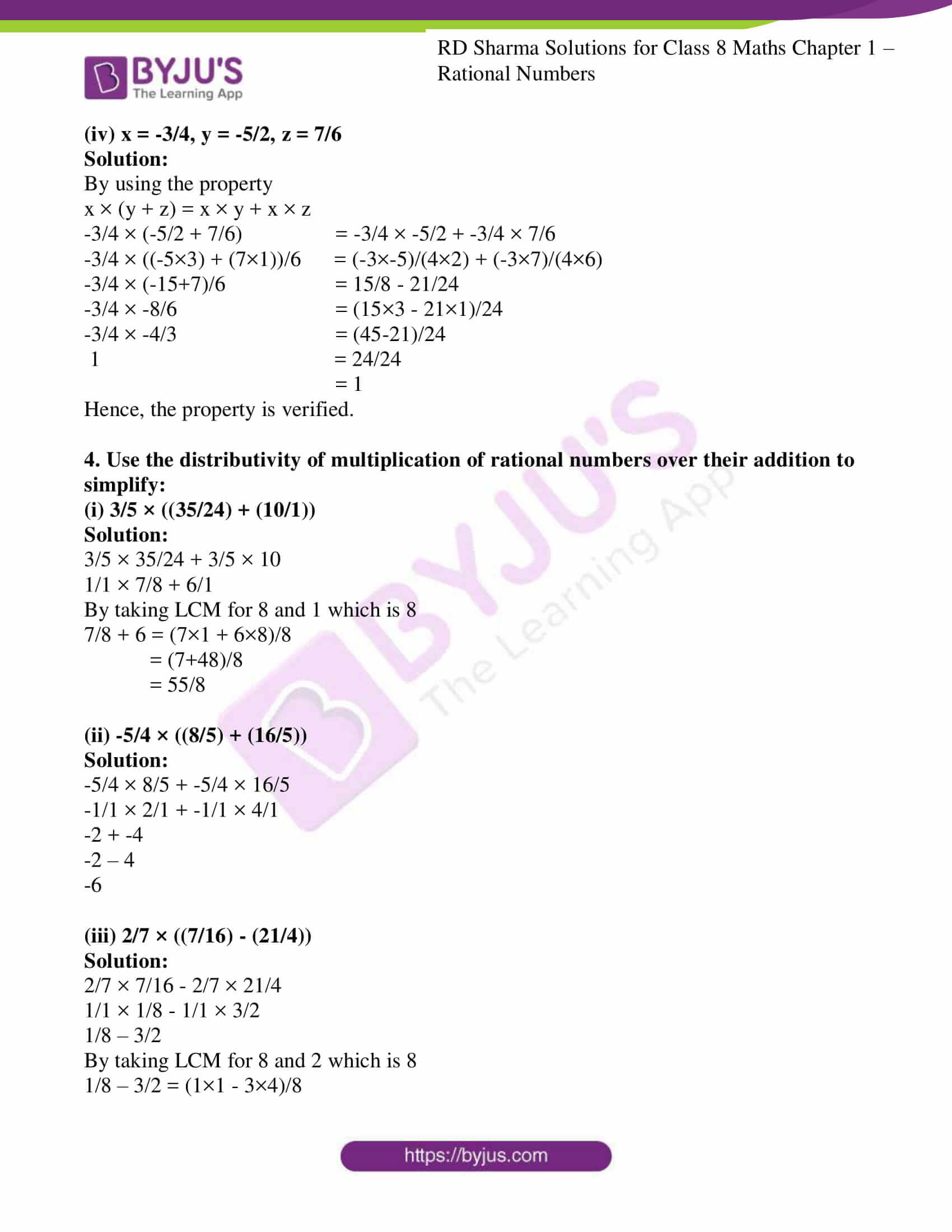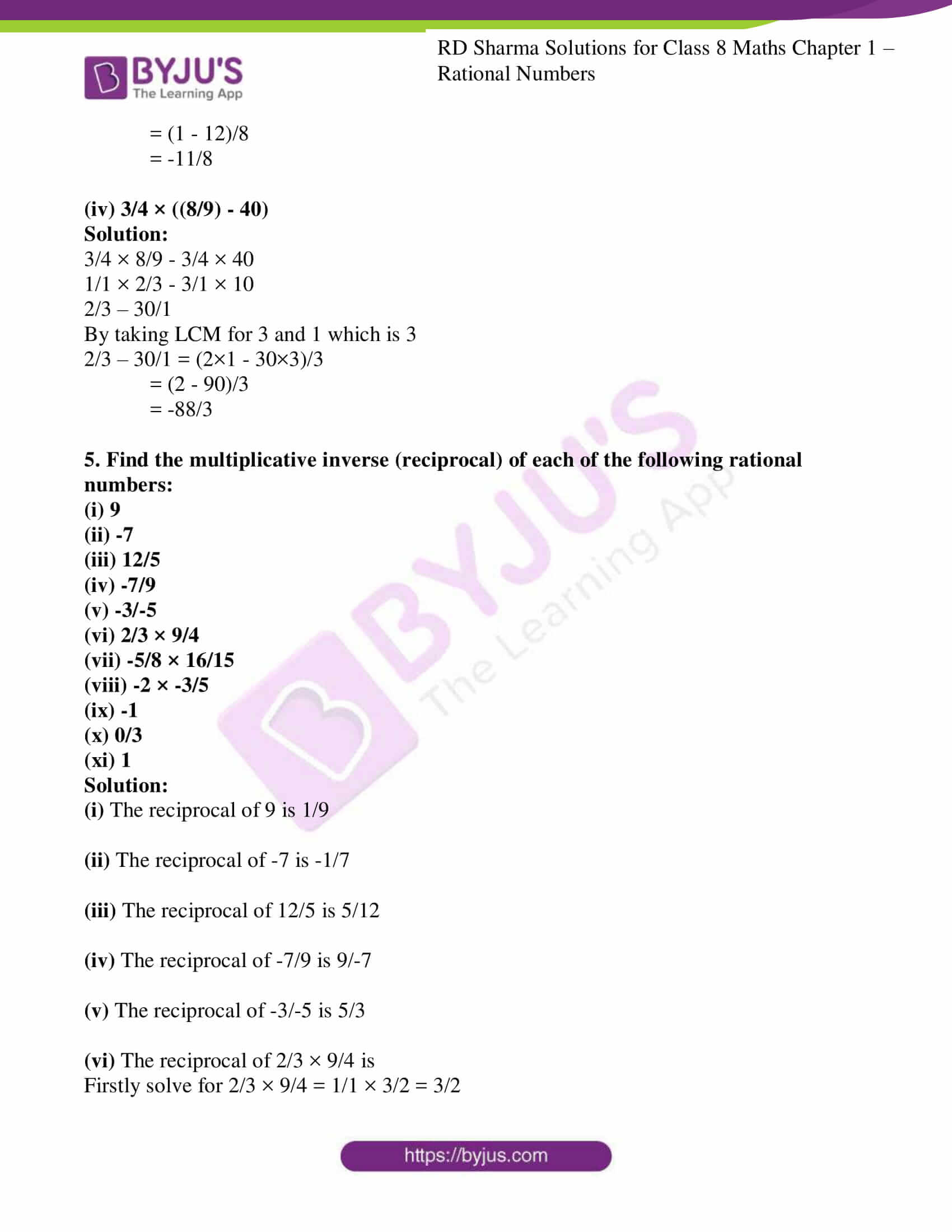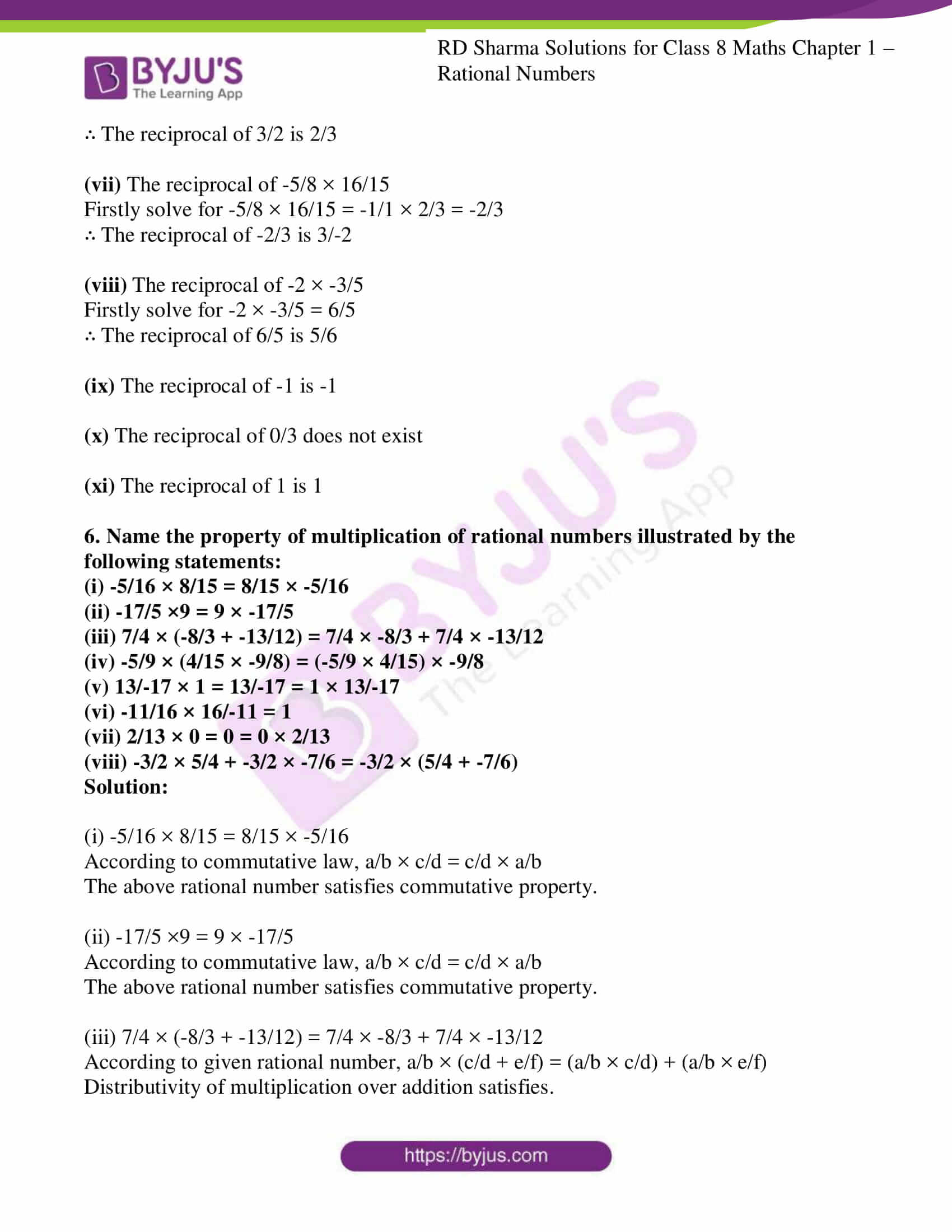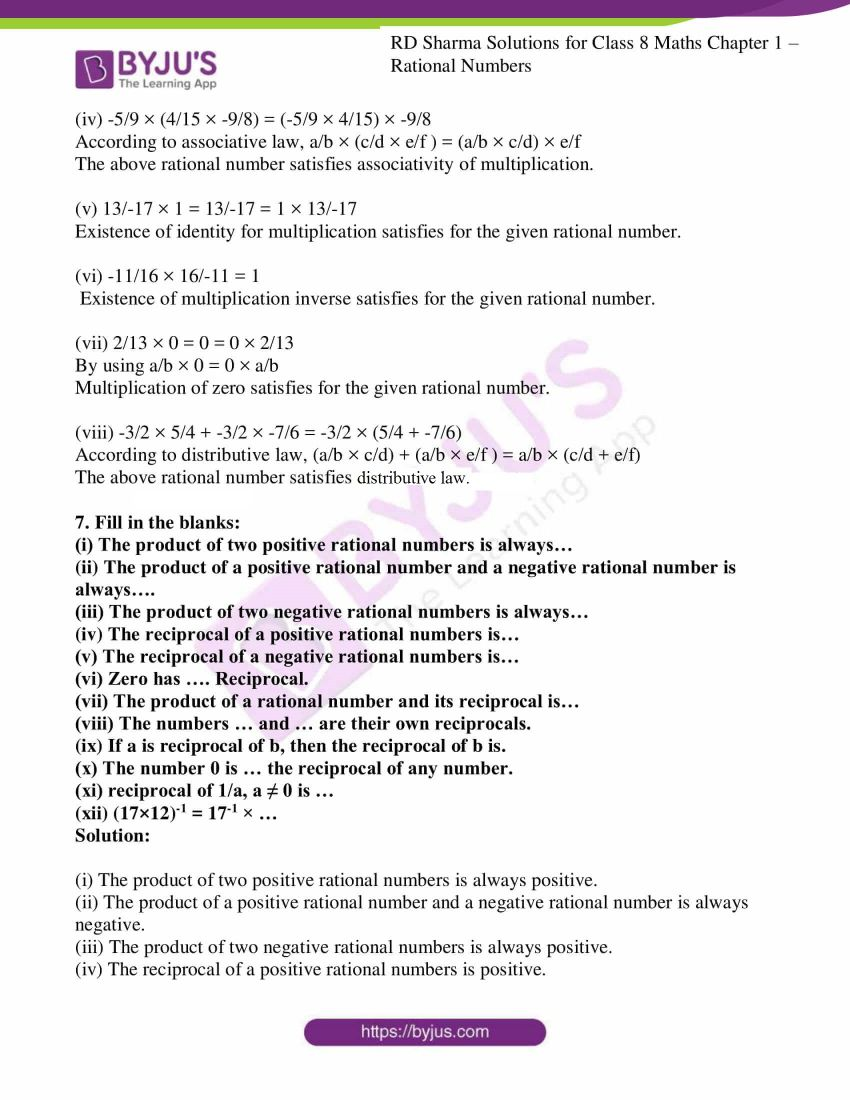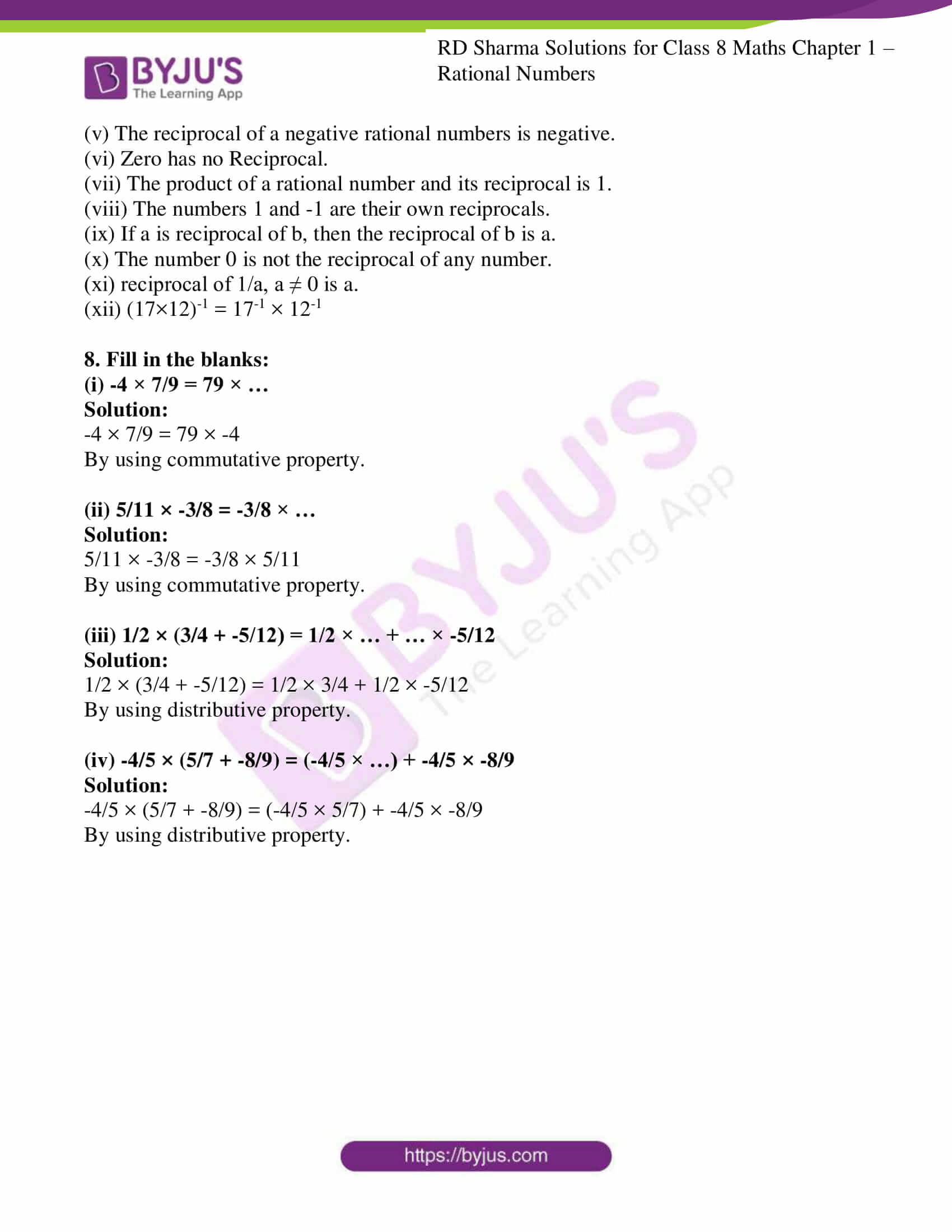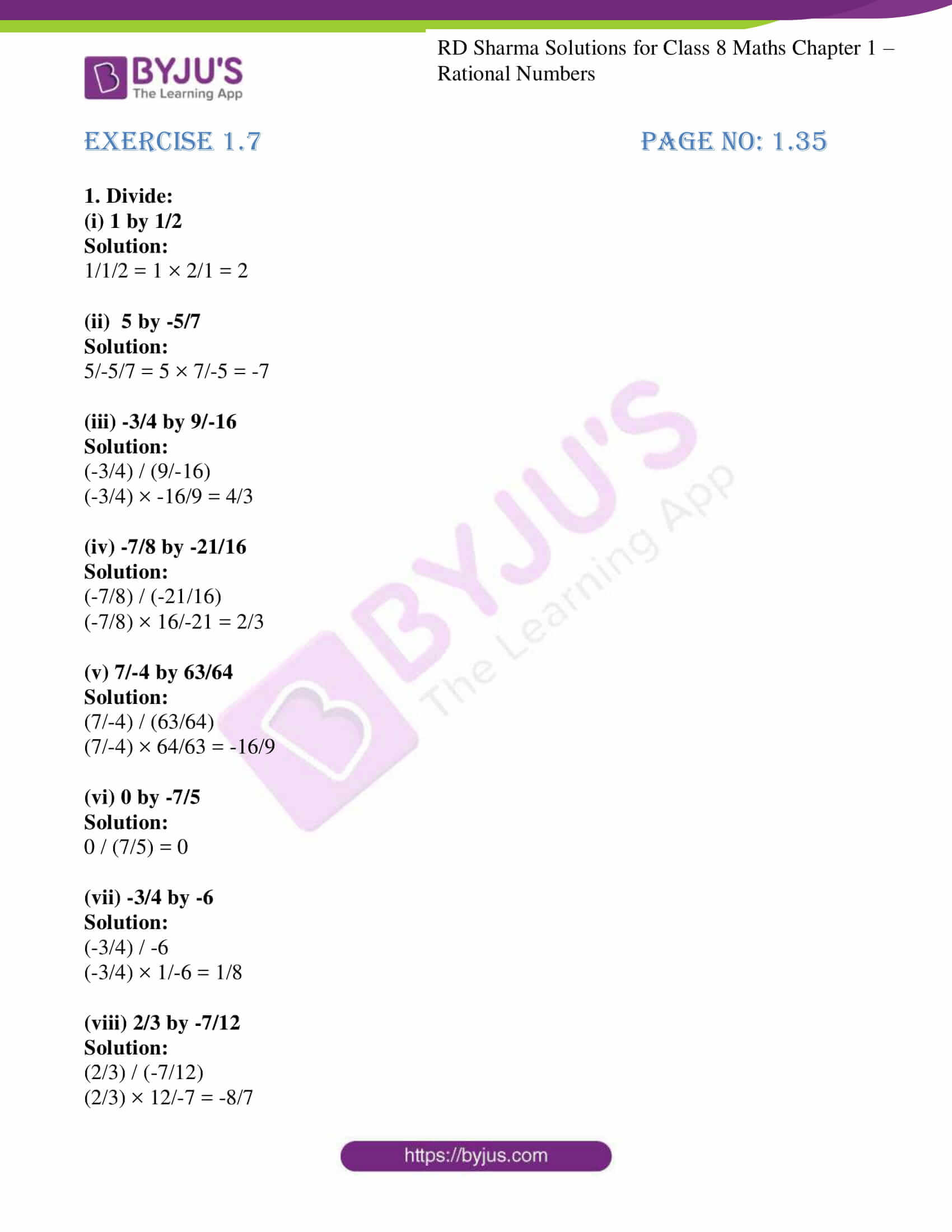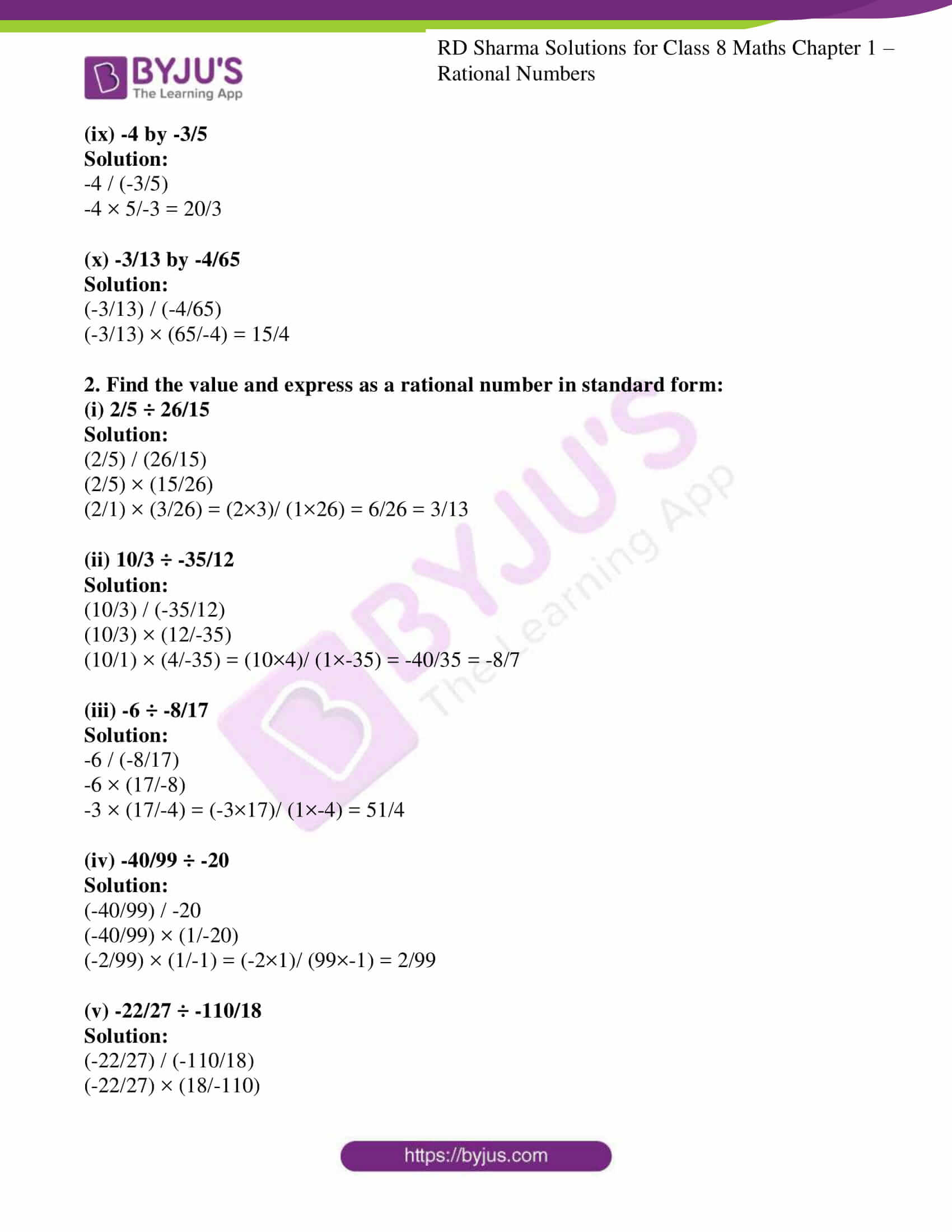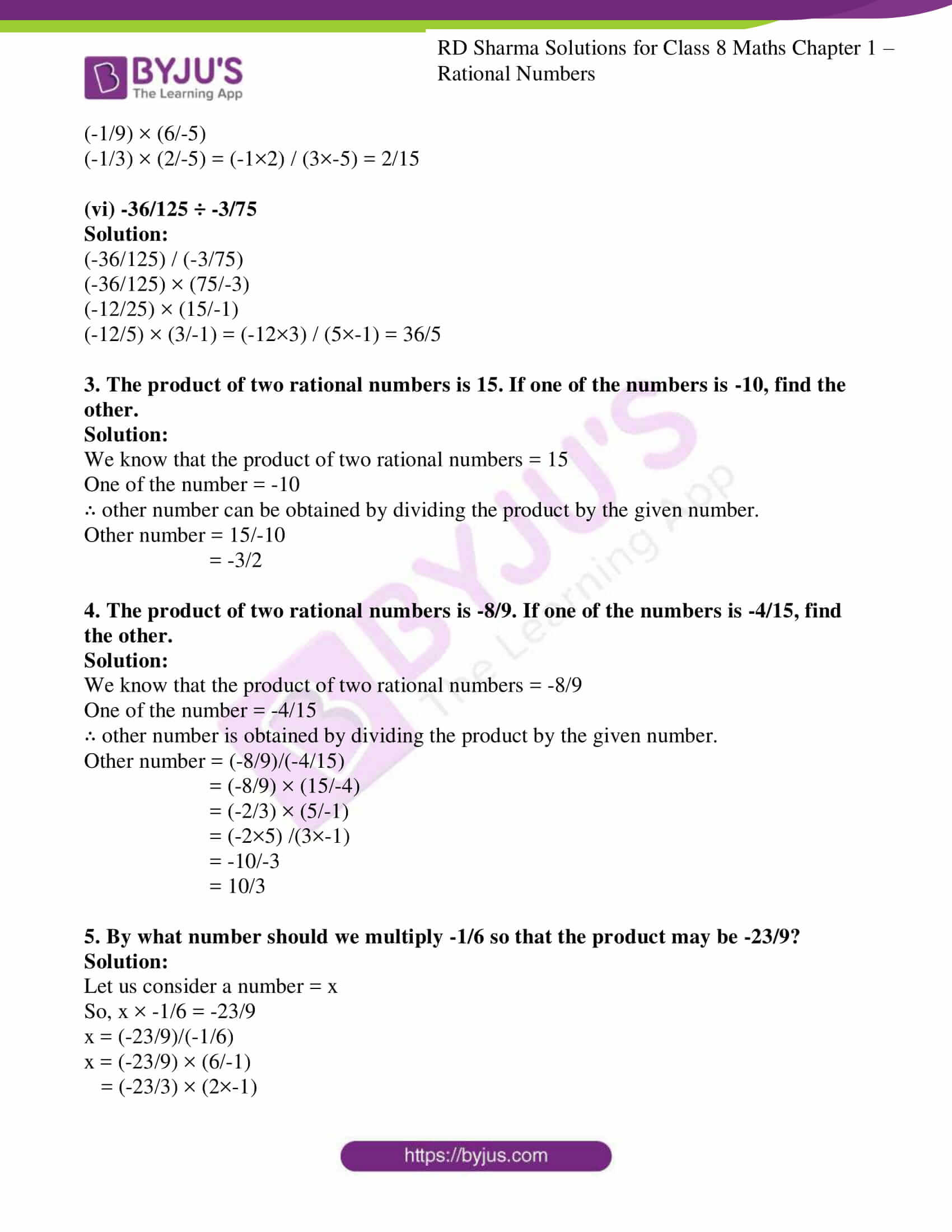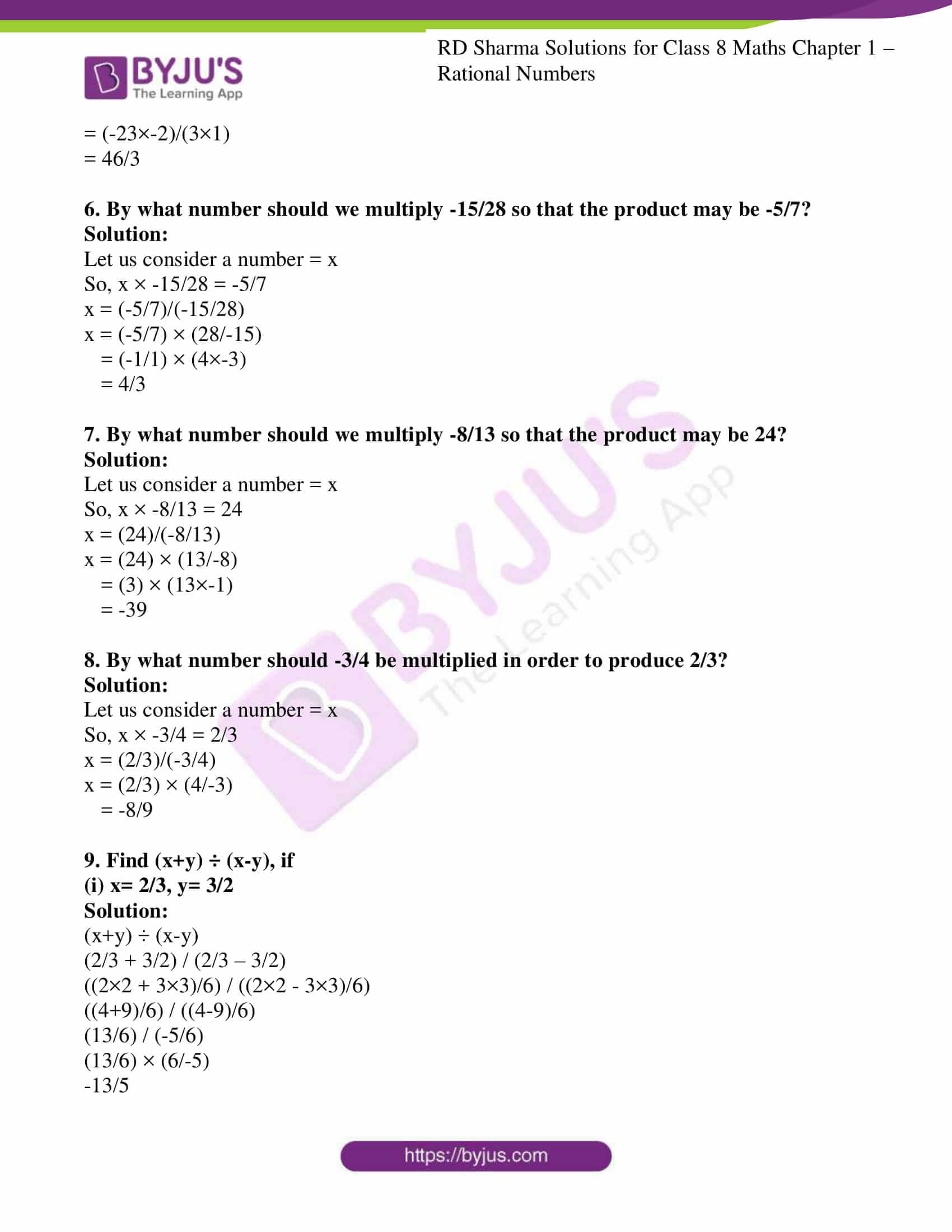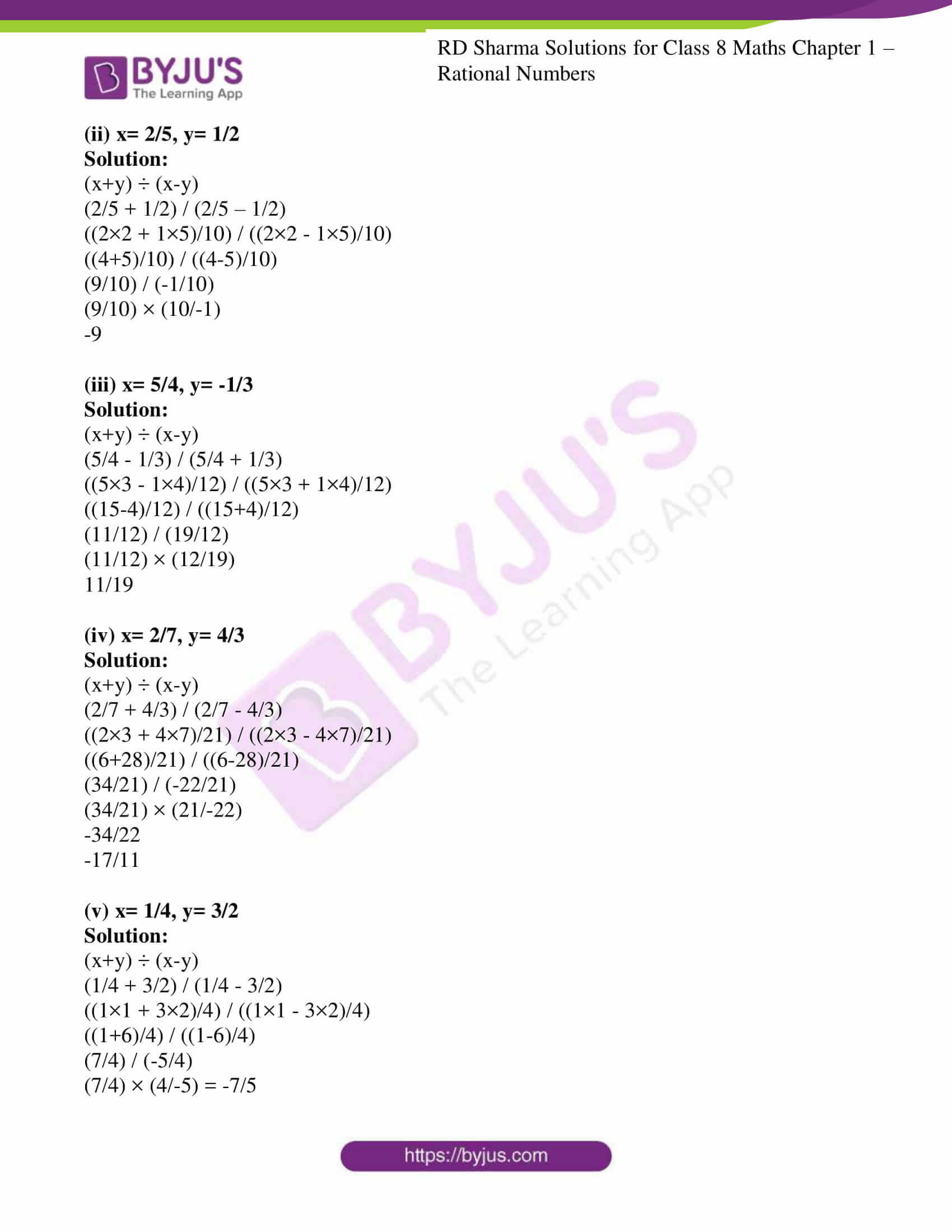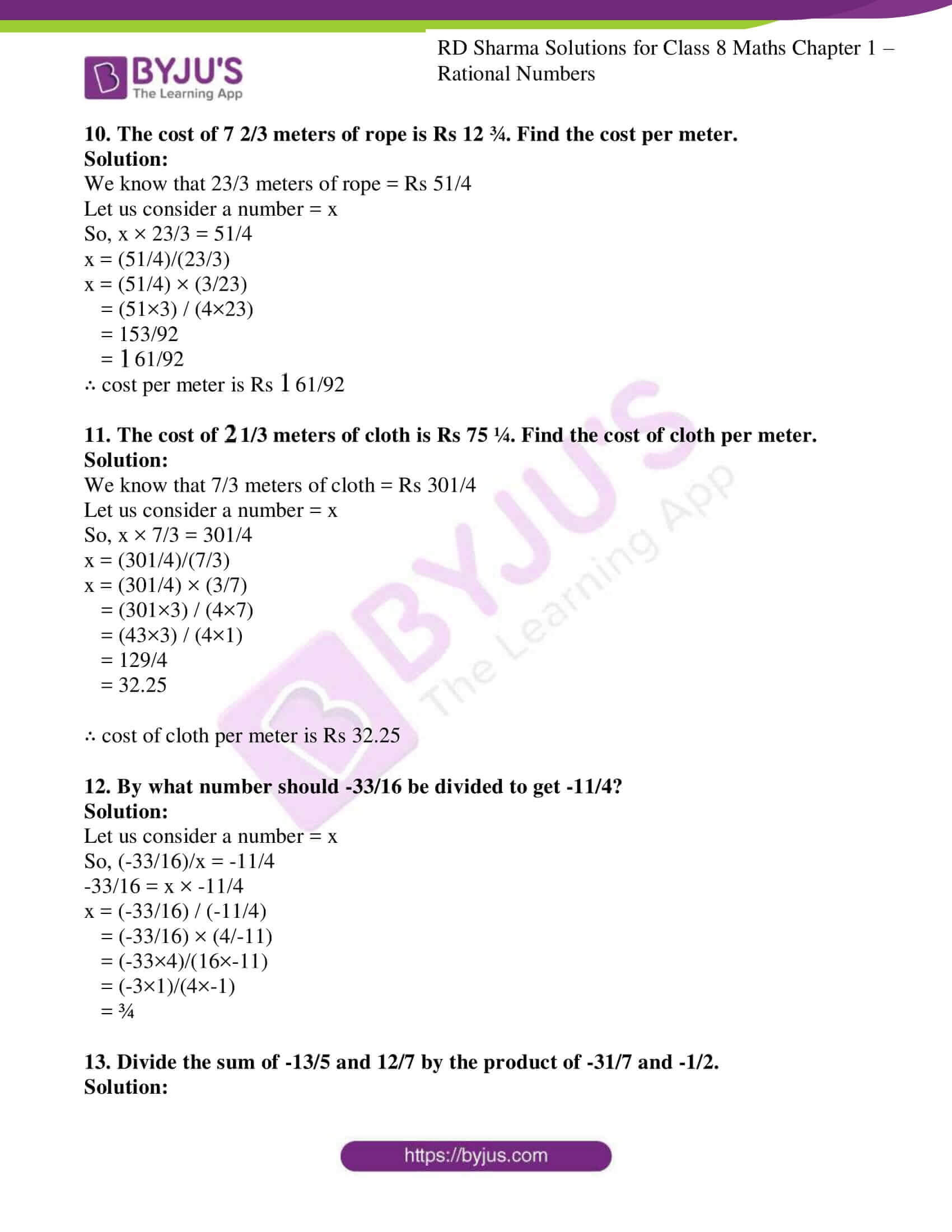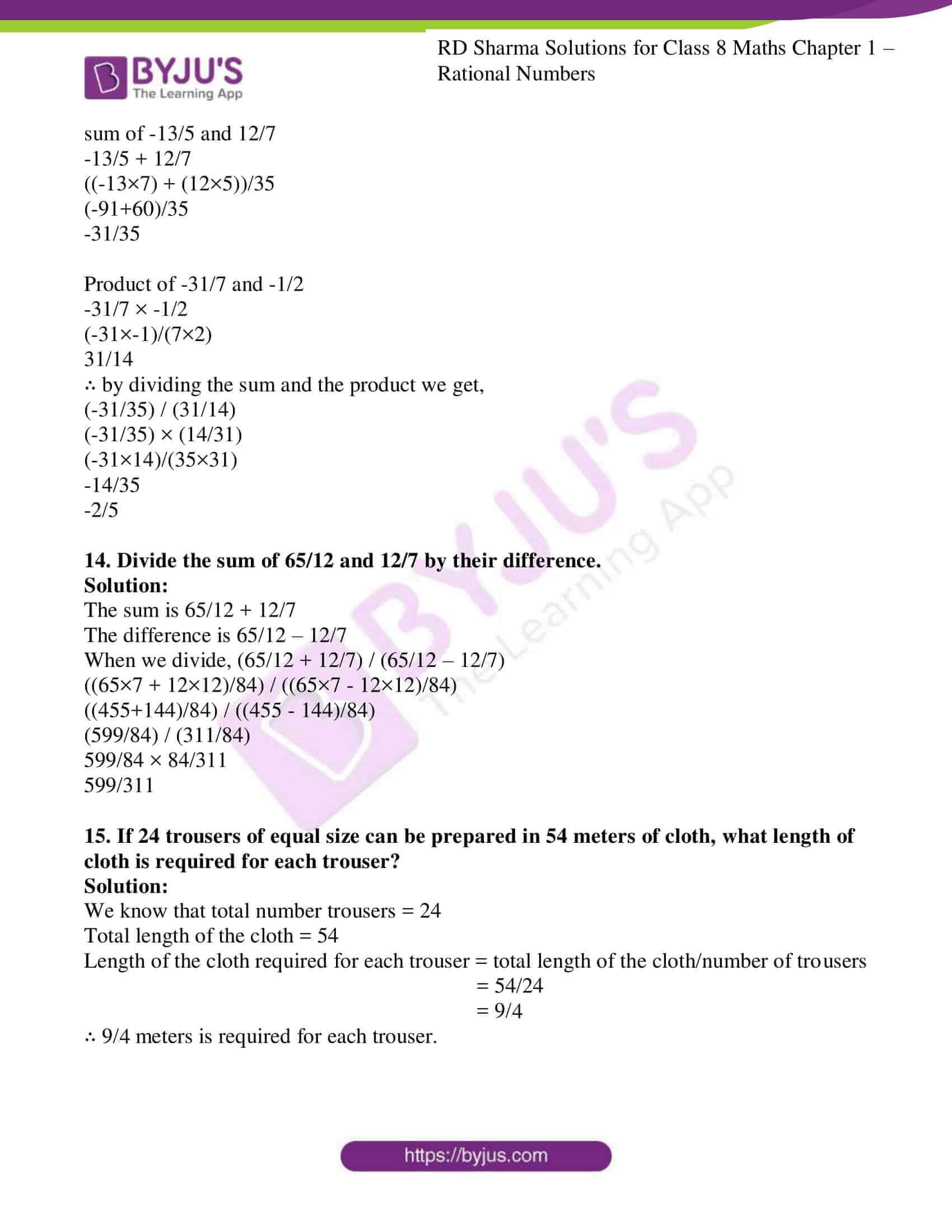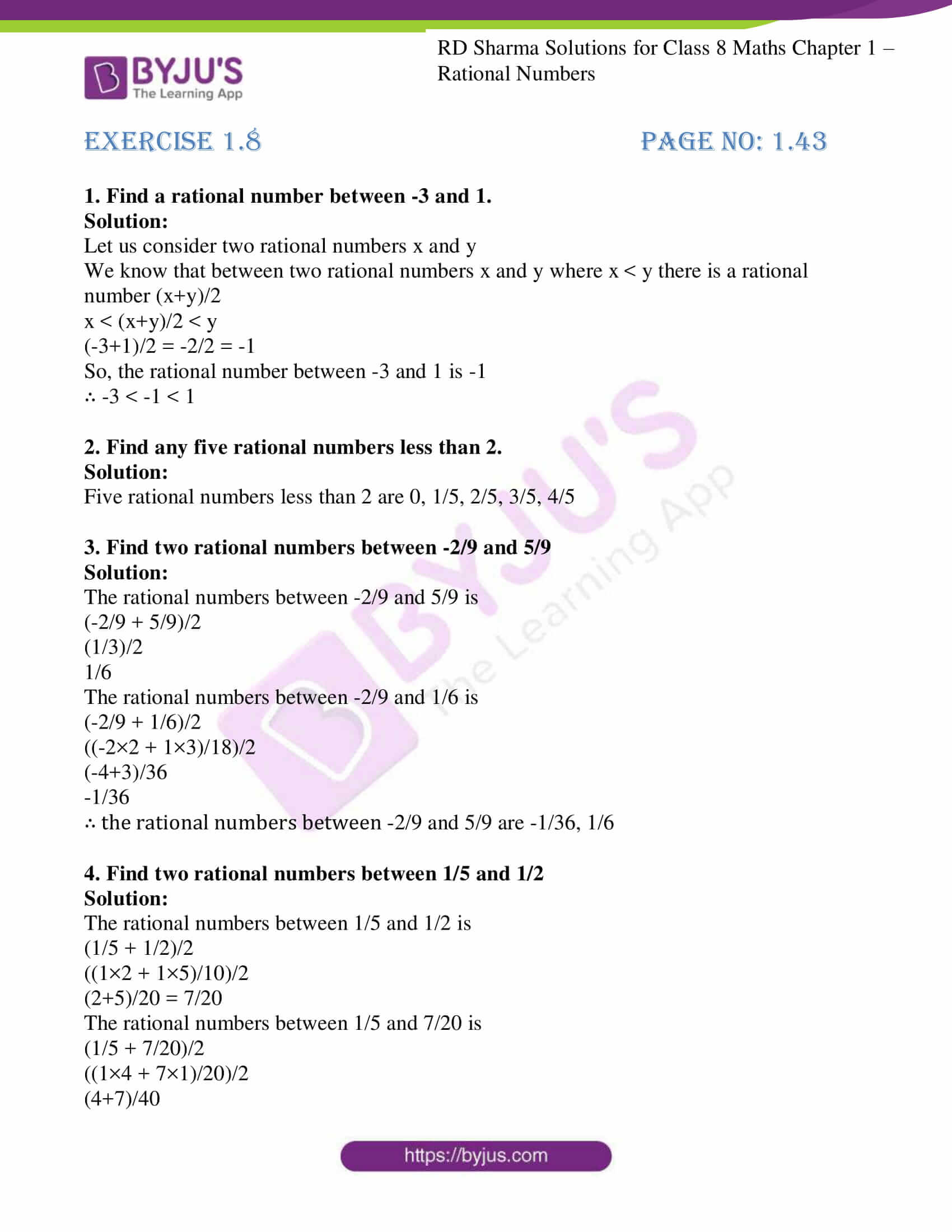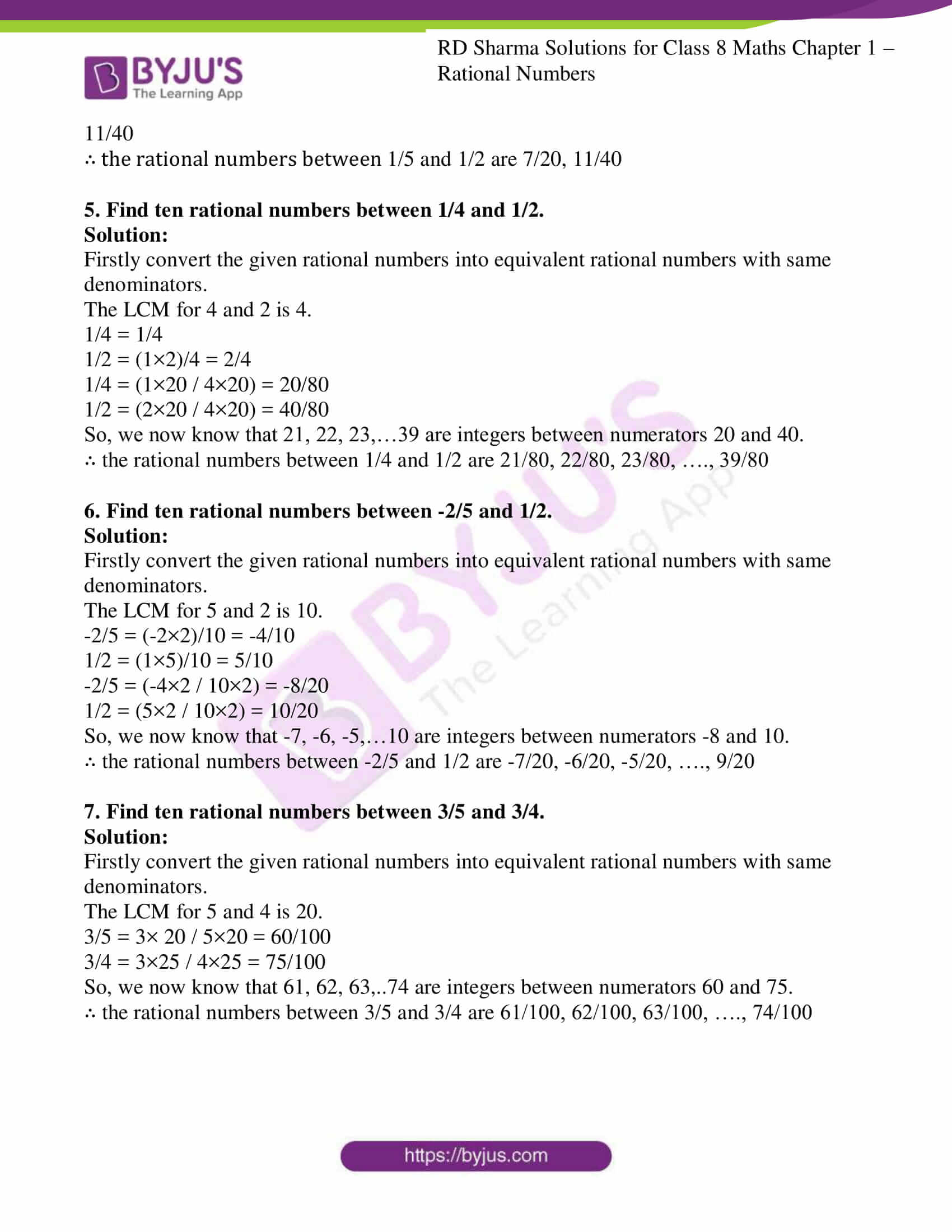## RD Sharma Solutions for Class 8 Maths Chapter 1 – Rational Numbers

Chapter 1- Rational Numbers contains 8 exercises and the RD Sharma Solutions present on this page provide the solutions for the questions present in each exercise.

## EXERCISE 1.1 PAGE NO: 1.5

1. Add the following rational numbers:

(i) -5/7 and 3/7

(ii) -15/4 and 7/4

(iii) -8/11 and -4/11

(iv) 6/13 and -9/13

Solution:

Since the denominators are of same positive numbers we can add them directly

(i) -5/7 + 3/7 = (-5+3)/7 = -2/7

(ii) -15/4 + 7/4 = (-15+7)/4 = -8/4

Further dividing by 4 we get,

-8/4 = -2

(iii) -8/11 + -4/11 = (-8 + (-4))/11 = (-8-4)/11 = -12/11

(iv) 6/13 + -9/13 = (6 + (-9))/13 = (6-9)/13 = -3/13

2. Add the following rational numbers:

(i) 3/4 and -5/8

Solution: The denominators are 4 and 8

By taking LCM for 4 and 8 is 8

We rewrite the given fraction in order to get the same denominator

3/4 = (3×2) / (4×2) = 6/8 and

-5/8 = (-5×1) / (8×1) = -5/8

Since the denominators are same we can add them directly

6/8 + -5/8 = (6 + (-5))/8 = (6-5)/8 = 1/8

(ii) 5/-9 and 7/3

Solution: Firstly we need to convert the denominators to positive numbers.

5/-9 = (5 × -1)/ (-9 × -1) = -5/9

The denominators are 9 and 3

By taking LCM for 9 and 3 is 9

We rewrite the given fraction in order to get the same denominator

-5/9 = (-5×1) / (9×1) = -5/9 and

7/3 = (7×3) / (3×3) = 21/9

Since the denominators are same we can add them directly

-5/9 + 21/9 = (-5+21)/9 = 16/9

(iii) -3 and 3/5

Solution: The denominators are 1 and 5

By taking LCM for 1 and 5 is 5

We rewrite the given fraction in order to get the same denominator

-3/1 = (-3×5) / (1×5) = -15/5 and

3/5 = (3×1) / (5×1) = 3/5

Now, the denominators are same we can add them directly

-15/5 + 3/5 = (-15+3)/5 = -12/5

(iv) -7/27 and 11/18

Solution: The denominators are 27 and 18

By taking LCM for 27 and 18 is 54

We rewrite the given fraction in order to get the same denominator

-7/27 = (-7×2) / (27×2) = -14/54 and

11/18 = (11×3) / (18×3) = 33/54

Now, the denominators are same we can add them directly

-14/54 + 33/54 = (-14+33)/54 = 19/54

(v) 31/-4 and -5/8

Solution: Firstly we need to convert the denominators to positive numbers.

31/-4 = (31 × -1)/ (-4 × -1) = -31/4

The denominators are 4 and 8

By taking LCM for 4 and 8 is 8

We rewrite the given fraction in order to get the same denominator

-31/4 = (-31×2) / (4×2) = -62/8 and

-5/8 = (-5×1) / (8×1) = -5/8

Since the denominators are same we can add them directly

-62/8 + (-5)/8 = (-62 + (-5))/8 = (-62-5)/8 = -67/8

(vi) 5/36 and -7/12

Solution: The denominators are 36 and 12

By taking LCM for 36 and 12 is 36

We rewrite the given fraction in order to get the same denominator

5/36 = (5×1) / (36×1) = 5/36 and

-7/12 = (-7×3) / (12×3) = -21/36

Now, the denominators are same we can add them directly

5/36 + -21/36 = (5 + (-21))/36 = 5-21/36 = -16/36 = -4/9

(vii) -5/16 and 7/24

Solution: The denominators are 16 and 24

By taking LCM for 16 and 24 is 48

We rewrite the given fraction in order to get the same denominator

-5/16 = (-5×3) / (16×3) = -15/48 and

7/24 = (7×2) / (24×2) = 14/48

Now, the denominators are same we can add them directly

-15/48 + 14/48 = (-15 + 14)/48 = -1/48

(viii) 7/-18 and 8/27

Solution: Firstly we need to convert the denominators to positive numbers.

7/-18 = (7 × -1)/ (-18 × -1) = -7/18

The denominators are 18 and 27

By taking LCM for 18 and 27 is 54

We rewrite the given fraction in order to get the same denominator

-7/18 = (-7×3) / (18×3) = -21/54 and

8/27 = (8×2) / (27×2) = 16/54

Since the denominators are same we can add them directly

-21/54 + 16/54 = (-21 + 16)/54 = -5/54

3.Simplify:

(i) 8/9 + -11/6

Solution: let us take the LCM for 9 and 6 which is 18

(8×2)/(9×2) + (-11×3)/(6×3)

16/18 + -33/18

Since the denominators are same we can add them directly

(16-33)/18 = -17/18

(ii) 3 + 5/-7

Solution: Firstly convert the denominator to positive number

5/-7 = (5×-1)/(-7×-1) = -5/7

3/1 + -5/7

Now let us take the LCM for 1 and 7 which is 7

(3×7)/(1×7) + (-5×1)/(7×1)

21/7 + -5/7

Since the denominators are same we can add them directly

(21-5)/7 = 16/7

(iii) 1/-12 + 2/-15

Solution: Firstly convert the denominator to positive number

1/-12 = (1×-1)/(-12×-1) = -1/12

2/-15 = (2×-1)/(-15×-1) = -2/15

-1/12 + -2/15

Now let us take the LCM for 12 and 15 which is 60

(-1×5)/(12×5) + (-2×4)/(15×4)

-5/60 + -8/60

Since the denominators are same we can add them directly

(-5-8)/60 = -13/60

(iv) -8/19 + -4/57

Solution: let us take the LCM for 19 and 57 which is 57

(-8×3)/(19×3) + (-4×1)/(57×1)

-24/57 + -4/57

Since the denominators are same we can add them directly

(-24-4)/57 = -28/57

(v) 7/9 + 3/-4

Solution: Firstly convert the denominator to positive number

3/-4 = (3×-1)/(-4×-1) = -3/4

7/9 + -3/4

Now let us take the LCM for 9 and 4 which is 36

(7×4)/(9×4) + (-3×9)/(4×9)

28/36 + -27/36

Since the denominators are same we can add them directly

(28-27)/36 = 1/36

(vi) 5/26 + 11/-39

Solution: Firstly convert the denominator to positive number

11/-39 = (11×-1)/(-39×-1) = -11/39

5/26 + -11/39

Now let us take the LCM for 26 and 39 which is 78

(5×3)/(26×3) + (-11×2)/(39×2)

15/78 + -22/78

Since the denominators are same we can add them directly

(15-22)/78 = -7/78

(vii) -16/9 + -5/12

Solution: let us take the LCM for 9 and 12 which is 108

(-16×12)/(9×12) + (-5×9)/(12×9)

-192/108 + -45/108

Since the denominators are same we can add them directly

(-192-45)/108 = -237/108

Further divide the fraction by 3 we get,

-237/108 = -79/36

(viii) -13/8 + 5/36

Solution: let us take the LCM for 8 and 36 which is 72

(-13×9)/(8×9) + (5×2)/(36×2)

-117/72 + 10/72

Since the denominators are same we can add them directly

(-117+10)/72 = -107/72

(ix) 0 + -3/5

Solution: We know that anything added to 0 results in the same.

0 + -3/5 = -3/5

(x) 1 + -4/5

Solution: let us take the LCM for 1 and 5 which is 5

(1×5)/(1×5) + (-4×1)/(5×1)

5/5 + -4/5

Since the denominators are same we can add them directly

(5-4)/5 = 1/5

4. Add and express the sum as a mixed fraction:

(i) -12/5 and 43/10

Solution: let us add the given fraction

-12/5 + 43/10

let us take the LCM for 5 and 10 which is 10

(-12×2)/(5×2) + (43×1)/(10×1)

-24/10 + 43/10

Since the denominators are same we can add them directly

(-24+43)/10 = 19/10

19/10 can be written as $$1\frac{9}{10}$$ in mixed fraction.

Solution: let us add the given fraction

let us take the LCM for 7 and 4 which is 28

(24×4)/(7×4) + (-11×7)/(4×7)

96/28 + -77/28

Since the denominators are same we can add them directly

(96-77)/28 = 19/28

(iii) -31/6 and -27/8

Solution: let us add the given fraction

-31/6 + -27/8

let us take the LCM for 6 and 8 which is 24

(-31×4)/(6×4) + (-27×3)/(8×3)

-124/24 + -81/24

Since the denominators are same we can add them directly

(-124-81)/24 = -205/24

-205/24 can be written as $$-8\frac{13}{24}$$ in mixed fraction.

(iv) 101/6 and 7/8

Solution: let us add the given fraction

101/6 + 7/8

let us take the LCM for 6 and 8 which is 24

(101×4)/(6×4) + (7×3)/(8×3)

404/24 + 21/24

Since the denominators are same we can add them directly

(404+21)/24 = 425/24

425/24 can be written as $$17\frac{17}{24}$$ in mixed fraction.

### EXERCISE 1.2 PAGE NO: 1.14

1. Verify commutativity of addition of rational numbers for each of the following pairs of rational numbers:

(i) -11/5 and 4/7

Solution: By using the commutativity law, the addition of rational numbers is commutative ∴ a/b + c/d = c/d + a/b

In order to verify the above property let us consider the given fraction

-11/5 and 4/7 as

-11/5 + 4/7 and 4/7 + -11/5

The denominators are 5 and 7

By taking LCM for 5 and 7 is 35

We rewrite the given fraction in order to get the same denominator

Now, -11/5 = (-11 × 7) / (5 ×7) = -77/35

4/7 = (4 ×5) / (7 ×5) = 20/35

Since the denominators are same we can add them directly

-77/35 + 20/35 = (-77+20)/35 = -57/35

4/7 + -11/5

The denominators are 7 and 5

By taking LCM for 7 and 5 is 35

We rewrite the given fraction in order to get the same denominator

Now, 4/7 = (4 × 5) / (7 ×5) = 20/35

-11/5 = (-11 ×7) / (5 ×7) = -77/35

Since the denominators are same we can add them directly

20/35 + -77/35 = (20 + (-77))/35 = (20-77)/35 = -57/35

∴ -11/5 + 4/7 = 4/7 + -11/5 is satisfied.

(ii) 4/9 and 7/-12

Solution: Firstly we need to convert the denominators to positive numbers.

7/-12 = (7 × -1)/ (-12 × -1) = -7/12

By using the commutativity law, the addition of rational numbers is commutative.

∴ a/b + c/d = c/d + a/b

In order to verify the above property let us consider the given fraction

4/9 and -7/12 as

4/9 + -7/12 and -7/12 + 4/9

The denominators are 9 and 12

By taking LCM for 9 and 12 is 36

We rewrite the given fraction in order to get the same denominator

Now, 4/9 = (4 × 4) / (9 ×4) = 16/36

-7/12 = (-7 ×3) / (12 ×3) = -21/36

Since the denominators are same we can add them directly

16/36 + (-21)/36 = (16 + (-21))/36 = (16-21)/36 = -5/36

-7/12 + 4/9

The denominators are 12 and 9

By taking LCM for 12 and 9 is 36

We rewrite the given fraction in order to get the same denominator

Now, -7/12 = (-7 ×3) / (12 ×3) = -21/36

4/9 = (4 × 4) / (9 ×4) = 16/36

Since the denominators are same we can add them directly

-21/36 + 16/36 = (-21 + 16)/36 = -5/36

∴ 4/9 + -7/12 = -7/12 + 4/9 is satisfied.

(iii) -3/5 and -2/-15

Solution:

By using the commutativity law, the addition of rational numbers is commutative.

∴ a/b + c/d = c/d + a/b

In order to verify the above property let us consider the given fraction

-3/5 and -2/-15 as

-3/5 + -2/-15 and -2/-15 + -3/5

-2/-15 = 2/15

The denominators are 5 and 15

By taking LCM for 5 and 15 is 15

We rewrite the given fraction in order to get the same denominator

Now, -3/5 = (-3 × 3) / (5×3) = -9/15

2/15 = (2 ×1) / (15 ×1) = 2/15

Since the denominators are same we can add them directly

-9/15 + 2/15 = (-9 + 2)/15 = -7/15

-2/-15 + -3/5

-2/-15 = 2/15

The denominators are 15 and 5

By taking LCM for 15 and 5 is 15

We rewrite the given fraction in order to get the same denominator

Now, 2/15 = (2 ×1) / (15 ×1) = 2/15

-3/5 = (-3 × 3) / (5×3) = -9/15

Since the denominators are same we can add them directly

2/15 + -9/15 = (2 + (-9))/15 = (2-9)/15 = -7/15

∴ -3/5 + -2/-15 = -2/-15 + -3/5 is satisfied.

(iv) 2/-7 and 12/-35

Solution: Firstly we need to convert the denominators to positive numbers.

2/-7 = (2 × -1)/ (-7 × -1) = -2/7

12/-35 = (12 × -1)/ (-35 × -1) = -12/35

By using the commutativity law, the addition of rational numbers is commutative.

∴ a/b + c/d = c/d + a/b

In order to verify the above property let us consider the given fraction

-2/7 and -12/35 as

-2/7 + -12/35 and -12/35 + -2/7

The denominators are 7 and 35

By taking LCM for 7 and 35 is 35

We rewrite the given fraction in order to get the same denominator

Now, -2/7 = (-2 × 5) / (7 ×5) = -10/35

-12/35 = (-12 ×1) / (35 ×1) = -12/35

Since the denominators are same we can add them directly

-10/35 + (-12)/35 = (-10 + (-12))/35 = (-10-12)/35 = -22/35

-12/35 + -2/7

The denominators are 35 and 7

By taking LCM for 35 and 7 is 35

We rewrite the given fraction in order to get the same denominator

Now, -12/35 = (-12 ×1) / (35 ×1) = -12/35

-2/7 = (-2 × 5) / (7 ×5) = -10/35

Since the denominators are same we can add them directly

-12/35 + -10/35 = (-12 + (-10))/35 = (-12-10)/35 = -22/35

∴ -2/7 + -12/35 = -12/35 + -2/7 is satisfied.

(v) 4 and -3/5

Solution: By using the commutativity law, the addition of rational numbers is commutative.

∴ a/b + c/d = c/d + a/b

In order to verify the above property let us consider the given fraction

4/1 and -3/5 as

4/1 + -3/5 and -3/5 + 4/1

The denominators are 1 and 5

By taking LCM for 1 and 5 is 5

We rewrite the given fraction in order to get the same denominator

Now, 4/1 = (4 × 5) / (1×5) = 20/5

-3/5 = (-3 ×1) / (5 ×1) = -3/5

Since the denominators are same we can add them directly

20/5 + -3/5 = (20 + (-3))/5 = (20-3)/5 = 17/5

-3/5 + 4/1

The denominators are 5 and 1

By taking LCM for 5 and 1 is 5

We rewrite the given fraction in order to get the same denominator

Now, -3/5 = (-3 ×1) / (5 ×1) = -3/5

4/1 = (4 × 5) / (1×5) = 20/5

Since the denominators are same we can add them directly

-3/5 + 20/5 = (-3 + 20)/5 = 17/5

∴ 4/1 + -3/5 = -3/5 + 4/1 is satisfied.

(vi) -4 and 4/-7

Solution: Firstly we need to convert the denominators to positive numbers.

4/-7 = (4 × -1)/ (-7 × -1) = -4/7

By using the commutativity law, the addition of rational numbers is commutative.

∴ a/b + c/d = c/d + a/b

In order to verify the above property let us consider the given fraction

-4/1 and -4/7 as

-4/1 + -4/7 and -4/7 + -4/1

The denominators are 1 and 7

By taking LCM for 1 and 7 is 7

We rewrite the given fraction in order to get the same denominator

Now, -4/1 = (-4 × 7) / (1×7) = -28/7

-4/7 = (-4 ×1) / (7 ×1) = -4/7

Since the denominators are same we can add them directly

-28/7 + -4/7 = (-28 + (-4))/7 = (-28-4)/7 = -32/7

-4/7 + -4/1

The denominators are 7 and 1

By taking LCM for 7 and 1 is 7

We rewrite the given fraction in order to get the same denominator

Now, -4/7 = (-4 ×1) / (7 ×1) = -4/7

-4/1 = (-4 × 7) / (1×7) = -28/7

Since the denominators are same we can add them directly

-4/7 + -28/7 = (-4 + (-28))/7 = (-4-28)/7 = -32/7

∴ -4/1 + -4/7 = -4/7 + -4/1 is satisfied.

2. Verify associativity of addition of rational numbers i.e., (x + y) + z = x + (y + z), when:

(i) x = ½, y = 2/3, z = -1/5

Solution: As the property states (x + y) + z = x + (y + z)

Use the values as such,

(1/2 + 2/3) + (-1/5) = 1/2 + (2/3 + (-1/5))

Let us consider LHS (1/2 + 2/3) + (-1/5)

Taking LCM for 2 and 3 is 6

(1× 3)/(2×3) + (2×2)/(3×2)

3/6 + 4/6

Since the denominators are same we can add them directly,

3/6 + 4/6 = 7/6

7/6 + (-1/5)

Taking LCM for 6 and 5 is 30

(7×5)/(6×5) + (-1×6)/(5×6)

35/30 + (-6)/30

Since the denominators are same we can add them directly,

(35+(-6))/30 = (35-6)/30 = 29/30

Let us consider RHS 1/2 + (2/3 + (-1/5))

Taking LCM for 3 and 5 is 15

(2/3 + (-1/5)) = (2×5)/(3×5) + (-1×3)/(5×3)

= 10/15 + (-3)/15

Since the denominators are same we can add them directly,

10/15 + (-3)/15 = (10-3)/15 = 7/15

1/2 + 7/15

Taking LCM for 2 and 15 is 30

1/2 + 7/15 = (1×15)/(2×15) + (7×2)/(15×2)

= 15/30 + 14/30

Since the denominators are same we can add them directly,

= (15 + 14)/30 = 29/30

∴ LHS = RHS associativity of addition of rational numbers is verified.

(ii) x = -2/5, y = 4/3, z = -7/10

Solution: As the property states (x + y) + z = x + (y + z)

Use the values as such,

(-2/5 + 4/3) + (-7/10) = -2/5 + (4/3 + (-7/10))

Let us consider LHS (-2/5 + 4/3) + (-7/10)

Taking LCM for 5 and 3 is 15

(-2× 3)/(5×3) + (4×5)/(3×5)

-6/15 + 20/15

Since the denominators are same we can add them directly,

-6/15 + 20/15= (-6+20)/15 = 14/15

14/15 + (-7/10)

Taking LCM for 15 and 10 is 30

(14×2)/(15×2) + (-7×3)/(10×3)

28/30 + (-21)/30

Since the denominators are same we can add them directly,

(28+(-21))/30 = (28-21)/30 = 7/30

Let us consider RHS -2/5 + (4/3 + (-7/10))

Taking LCM for 3 and 10 is 30

(4/3 + (-7/10)) = (4×10)/(3×10) + (-7×3)/(10×3)

= 40/30 + (-21)/30

Since the denominators are same we can add them directly,

40/30 + (-21)/30 = (40-21)/30 = 19/30

-2/5 + 19/30

Taking LCM for 5 and 30 is 30

-2/5 + 19/30 = (-2×6)/(5×6) + (19×1)/(30×1)

= -12/30 + 19/30

Since the denominators are same we can add them directly,

= (-12 + 19)/30 = 7/30

∴ LHS = RHS associativity of addition of rational numbers is verified.

(iii) x = -7/11, y = 2/-5, z = -3/22

Solution: Firstly convert the denominators to positive numbers

2/-5 = (2×-1)/ (-5×-1) = -2/5

As the property states (x + y) + z = x + (y + z)

Use the values as such,

(-7/11 + -2/5) + (-3/22) = -7/11 + (-2/5 + (-3/22))

Let us consider LHS (-7/11 + -2/5) + (-3/22)

Taking LCM for 11 and 5 is 55

(-7×5)/(11×5) + (-2×11)/(5×11)

-35/55 + -22/55

Since the denominators are same we can add them directly,

-35/55 + -22/55 = (-35-22)/55 = -57/55

-57/55 + (-3/22)

Taking LCM for 55 and 22 is 110

(-57×2)/(55×2) + (-3×5)/(22×5)

-114/110 + (-15)/110

Since the denominators are same we can add them directly,

(-114+(-15))/110 = (-114-15)/110 = -129/110

Let us consider RHS -7/11 + (-2/5 + (-3/22))

Taking LCM for 5 and 22 is 110

(-2/5 + (-3/22))= (-2×22)/(5×22) + (-3×5)/(22×5)

= -44/110 + (-15)/110

Since the denominators are same we can add them directly,

-44/110 + (-15)/110 = (-44-15)/110 = -59/110

-7/11 + -59/110

Taking LCM for 11 and 110 is 110

-7/11 + -59/110 = (-7×10)/(11×10) + (-59×1)/(110×1)

= -70/110 + -59/110

Since the denominators are same we can add them directly,

= (-70 -59)/110 = -129/110

∴ LHS = RHS associativity of addition of rational numbers is verified.

(iv) x = -2, y = 3/5, z = -4/3

Solution: As the property states (x + y) + z = x + (y + z)

Use the values as such,

(-2/1 + 3/5) + (-4/3) = -2/1 + (3/5 + (-4/3))

Let us consider LHS (-2/1 + 3/5) + (-4/3)

Taking LCM for 1 and 5 is 5

(-2×5)/(1×5) + (3×1)/(5×1)

-10/5 + 3/5

Since the denominators are same we can add them directly,

-10/5 + 3/5= (-10+3)/5 = -7/5

-7/5 + (-4/3)

Taking LCM for 5 and 3 is 15

(-7×3)/(5×3) + (-4×5)/(3×5)

-21/15 + (-20)/15

Since the denominators are same we can add them directly,

(-21+(-20))/15 = (-21-20)/15 = -41/15

Let us consider RHS -2/1 + (3/5 + (-4/3))

Taking LCM for 5 and 3 is 15

(3/5 + (-4/3)) = (3×3)/(5×3) + (-4×5)/(3×5)

= 9/15 + (-20)/15

Since the denominators are same we can add them directly,

9/15 + (-20)/15 = (9-20)/15 = -11/15

-2/1 + -11/15

Taking LCM for 1 and 15 is 15

-2/1 + -11/15 = (-2×15)/(1×15) + (-11×1)/(15×1)

= -30/15 + -11/15

Since the denominators are same we can add them directly,

= (-30 -11)/15 = -41/15

∴ LHS = RHS associativity of addition of rational numbers is verified.

3. Write the additive of each of the following rational numbers:

(i) -2/17

(ii) 3/-11

(iii) -17/5

(iv) -11/-25

Solution:

(i) The additive inverse of -2/17 is 2/17

(ii) The additive inverse of 3/-11 is 3/11

(iii) The additive inverse of -17/5 is 17/5

(iv) The additive inverse of -11/-25 is -11/25

4. Write the negative(additive) inverse of each of the following:

(i) -2/5

(ii) 7/-9

(iii) -16/13

(iv) -5/1

(v) 0

(vi) 1

(vii) – 1

Solution:

(i) The negative (additive) inverse of -2/5 is 2/5

(ii) The negative (additive) inverse of 7/-9 is 7/9

(iii) The negative (additive) inverse of -16/13 is 16/13

(iv) The negative (additive) inverse of -5/1 is 5

(v) The negative (additive) inverse of 0 is 0

(vi) The negative (additive) inverse of 1 is -1

(vii) The negative (additive) inverse of -1 is 1

5. Using commutativity and associativity of addition of rational numbers, express each of the following as a rational number:

(i) 2/5 + 7/3 + -4/5 + -1/3

Solution: Firstly group the rational numbers with same denominators

2/5 + -4/5 + 7/3 + -1/3

Now the denominators which are same can be added directly.

(2+(-4))/5 + (7+(-1))/3

(2-4)/5 + (7-1)/3

-2/5 + 6/3

By taking LCM for 5 and 3 we get, 15

(-2×3)/(5×3) + (6×5)/(3×5)

-6/15 + 30/15

Since the denominators are same can be added directly

(-6+30)/15 = 24/15

Further can be divided by 3 we get,

24/15 = 8/5

(ii) 3/7 + -4/9 + -11/7 + 7/9

Solution: Firstly group the rational numbers with same denominators

3/7 + -11/7 + -4/9 + 7/9

Now the denominators which are same can be added directly.

(3+ (-11))/7 + (-4+ 7)/9

(3-11)/7 + (-4+7)/9

-8/7 + 3/9

-8/7 + 1/3

By taking LCM for 7 and 3 we get, 21

(-8×3)/ (7×3) + (1×7)/ (3×7)

-24/21 + 7/21

Since the denominators are same can be added directly

(-24+7)/21 = -17/21

(iii) 2/5 + 8/3 + -11/15 + 4/5 + -2/3

Solution: Firstly group the rational numbers with same denominators

2/5 + 4/5 + 8/3 + -2/3 + -11/15

Now the denominators which are same can be added directly.

(2 + 4)/5 + (8 + (-2))/3 + -11/15

6/5 + (8-2)/3 + -11/15

6/5 + 6/3 + -11/15

6/5 + 2/1 + -11/15

By taking LCM for 5, 1 and 15 we get, 15

(6×3)/ (5×3) + (2×15)/ (1×15) + (-11×1)/ (15×1)

18/15 + 30/15 + -11/15

Since the denominators are same can be added directly

(18+30+ (-11))/15 = (18+30-11)/15 = 37/15

(iv) 4/7 + 0 + -8/9 + -13/7 + 17/21

Solution: Firstly group the rational numbers with same denominators

4/7 + -13/7 + -8/9 + 17/21

Now the denominators which are same can be added directly.

(4 + (-13))/7 + -8/9 + 17/21

(4-13)/7 + -8/9 + 17/21

-9/7 + -8/9 + 17/21

By taking LCM for 7, 9 and 21 we get, 63

(-9×9)/ (7×9) + (-8×7)/ (9×7) + (17×3)/ (21×3)

-81/63 + -56/63 + 51/63

Since the denominators are same can be added directly

(-81+(-56)+ 51)/63 = (-81-56+51)/63 = -86/63

6. Re-arrange suitably and find the sum in each of the following:

(i) 11/12 + -17/3 + 11/2 + -25/2

Solution: Firstly group the rational numbers with same denominators

11/12 + -17/3 + (11-25)/2

11/12 + -17/3 + -14/2

By taking LCM for 12, 3 and 2 we get, 12

(11×1)/(12×1) + (-17×4)/(3×4) + (-14×6)/(2×6)

11/12 + -68/12 + -84/12

Since the denominators are same can be added directly

(11-68-84)/12 = -141/12

(ii)-6/7 + -5/6 + -4/9 + -15/7

Solution: Firstly group the rational numbers with same denominators

-6/7 + -15/7 + -5/6 + -4/9

(-6 -15)/7 + -5/6 + -4/9

-21/7 + -5/6 + -4/9

-3/1 + -5/6 + -4/9

By taking LCM for 1, 6 and 9 we get, 18

(-3×18)/(1×18) + (-5×3)/(6×3) + (-4×2)/(9×2)

-54/18 + -15/18 + -8/18

Since the denominators are same can be added directly

(-54-15-8)/18 = -77/18

(iii) 3/5 + 7/3 + 9/ 5+ -13/15 + -7/3

Solution: Firstly group the rational numbers with same denominators

3/5 + 9/5 + 7/3 + -7/3 + -13/15

(3+9)/5 + -13/15

12/5 + -13/15

By taking LCM for 5 and 15 we get, 15

(12×3)/(5×3) + (-13×1)/(15×1)

36/15 + -13/15

Since the denominators are same can be added directly

(36-13)/15 = 23/15

(iv) 4/13 + -5/8 + -8/13 + 9/13

Solution: Firstly group the rational numbers with same denominators

4/13 + -8/13 + 9/13 + -5/8

(4-8+9)/13 + -5/8

5/13 + -5/8

By taking LCM for 13 and 8 we get, 104

(5×8)/(13×8) + (-5×13)/(8×13)

40/104 + -65/104

Since the denominators are same can be added directly

(40-65)/104 = -25/104

(v) 2/3 + -4/5 + 1/3 + 2/5

Solution: Firstly group the rational numbers with same denominators

2/3 + 1/3 + -4/5 + 2/5

(2+1)/3 + (-4+2)/5

3/3 + -2/5

1/1 + -2/5

By taking LCM for 1 and 5 we get, 5

(1×5)/(1×5) + (-2×1)/(5×1)

5/5 + -2/5

Since the denominators are same can be added directly

(5-2)/5 = 3/5

(vi) 1/8 + 5/12 + 2/7 + 7/12 + 9/7 + -5/16

Solution: Firstly group the rational numbers with same denominators

1/8 + 5/12 + 7/12 + 2/7 + 9/7 + -5/16

1/8 + (5+7)/12 + (2+9)/7 + -5/16

1/8 + 12/12 + 11/7 + -5/16

1/8 + 1/1 + 11/7 + -5/16

By taking LCM for 8, 1, 7 and 16 we get, 112

(1×14)/(8×14) + (1×112)/(1×112) + (11×16)/(7×16) + (-5×7)/(16×7)

14/112 + 112/112 + 176/112 + -35/112

Since the denominators are same can be added directly

(14+112+176-35)/112 = 267/112

### EXERCISE 1.3 PAGE NO: 1.18

1. Subtract the first rational number from the second in each of the following:

(i) 3/8, 5/8

(ii) -7/9, 4/9

(iii) -2/11, -9/11

(iv) 11/13, -4/13

(v) ¼, -3/8

(vi) -2/3, 5/6

(vii) -6/7, -13/14

(viii) -8/33, -7/22

Solution:

(i) let us subtract

5/8 – 3/8

Since the denominators are same we can subtract directly

(5-3)/8 = 2/8

Further we can divide by 2 we get,

2/8 = 1/4

(ii) let us subtract

4/9 – -7/9

Since the denominators are same we can subtract directly

(4+7)/9 = 11/9

(iii) let us subtract

-9/11 – -2/11

Since the denominators are same we can subtract directly

(-9+2)/11 = -7/11

(iv) let us subtract

-4/13 – 11/13

Since the denominators are same we can subtract directly

(-4-11)/13 = -15/13

(v) let us subtract

-3/8 – 1/4

By taking LCM for 8 and 4 which is 8

-3/8 – 1/4 = (-3×1)/(8×1) – (1×2)/(4×2) = -3/8 – 2/8

Since the denominators are same we can subtract directly

(-3-2)/8 = -5/8

(vi) let us subtract

5/6 – -2/3

By taking LCM for 6 and 3 which is 6

5/6 – -2/3 = (5×1)/(6×1) – (-2×2)/(3×2) = 5/6 – -4/6

Since the denominators are same we can subtract directly

(5+4)/6 = 9/6

Further we can divide by 3 we get,

9/6 = 3/2

(vii) let us subtract

-13/14 – -6/7

By taking LCM for 14 and 7 which is 14

-13/14 – -6/7 = (-13×1)/(14×1) – (-6×2)/(7×2) = -13/14 – -12/14

Since the denominators are same we can subtract directly

(-13+12)/14 = -1/14

(viii) let us subtract

-7/22 – -8/33

By taking LCM for 22 and 33 which is 66

-7/22 – -8/33 = (-7×3)/(22×3) – (-8×2)/(33×2) = -21/66 – -16/66

Since the denominators are same we can subtract directly

(-21+16)/66 = -5/66

2. Evaluate each of the following:

(i) 2/3 – 3/5

Solution: By taking LCM for 3 and 5 which is 15

2/3 – 3/5 = (2×5 – 3×3)/15

= 1/15

(ii) -4/7 – 2/-3

Solution: convert the denominator to positive number by multiplying by -1

2/-3 = -2/3

-4/7 – -2/3

By taking LCM for 7 and 3 which is 21

-4/7 – -2/3 = (-4×3 – -2×7)/21

= (-12+14)/21

= 2/21

(iii) 4/7 – -5/-7

Solution: convert the denominator to positive number by multiplying by -1

-5/-7 = 5/7

4/7 – 5/7

Since the denominators are same we can subtract directly

(4-5)/7 = -1/7

(iv) -2 – 5/9

Solution: By taking LCM for 1 and 9 which is 9

-2/1 – 5/9 = (-2×9 – 5×1)/9

= (-18 – 5)/9

= -23/9

(v) -3/-8 – -2/7

Solution: convert the denominator to positive number by multiplying by -1

-3/-8 = 3/8

3/8 – -2/7

By taking LCM for 8 and 7 which is 56

3/8 – -2/7 = (3×7 – -2×8)/56

= (21 + 16)/56

= 37/56

(vi) -4/13 – -5/26

Solution: By taking LCM for 13 and 26 which is 26

-4/13 – -5/26 = (-4×2 – -5×1)/26

= (-8 + 5)/26

= -3/26

(vii) -5/14 – -2/7

Solution: By taking LCM for 14 and 7 which is 14

-5/14 – -2/7 = (-5×1 – -2×2)/14

= (-5 + 4)/14

= -1/14

(viii) 13/15 – 12/25

Solution: By taking LCM for 15 and 25 which is 75

13/15 – 12/25 = (13×5 – 12×3)/75

= (65 – 36)/75

= 29/75

(ix) -6/13 – -7/13

Solution: Since the denominators are same we can subtract directly

-6/13 – -7/13 = (-6+7)/13

= 1/13

(x) 7/24 – 19/36

Solution: By taking LCM for 24 and 36 which is 72

7/24 – 19/36 = (7×3 – 19×2)/72

= (21 – 38)/72

= -17/72

(xi) 5/63 – -8/21

Solution: By taking LCM for 63 and 21 which is 63

5/63 – -8/21 = (5×1 – -8×3)/63

= (5 + 24)/63

= 29/63

3. The sum of the two numbers is 5/9. If one of the numbers is 1/3, find the other.

Solution: Let us note down the given details

Sum of two numbers = 5/9

One of the number = 1/3

By using the formula,

Other number = sum of number – given number

= 5/9 – 1/3

By taking LCM for 9 and 3 which is 9

5/9 – 1/3 = (5×1 – 1×3)/9

= (5 – 3)/9

= 2/9

∴ the other number is 2/9

4. The sum of the two numbers is -1/3. If one of the numbers is -12/3, find the other.

Solution: Let us note down the given details

Sum of two numbers = -1/3

One of the number = -12/3

By using the formula,

Other number = sum of number – given number

= -1/3 – -12/3

Since the denominators are same we can subtract directly

= (-1+12)/3 = 11/3

∴ the other number is 11/3

5. The sum of the two numbers is -4/3. If one of the numbers is -5, find the other.

Solution: Let us note down the given details

Sum of two numbers = -4/3

One of the number = -5/1

By using the formula,

Other number = sum of number – given number

= -4/3 – -5/1

By taking LCM for 3 and 1 which is 3

-4/3 – -5/1 = (-4×1 – -5×3)/3

= (-4 + 15)/3

= 11/3

∴ the other number is 11/3

6. The sum of the two rational numbers is -8. If one of the numbers is -15/7, find the other.

Solution: Let us note down the given details

Sum of two rational numbers = -8/1

One of the number = -15/7

Let us consider the other number as x

x + -15/7 = -8

(7x -15)/7 = -8

7x -15 = -8×7

7x – 15 = -56

7x = -56+15

x = -41/7

∴ the other number is -41/7

7. What should be added to -7/8 so as to get 5/9?

Solution: Let us consider a number as x to be added to -7/8 to get 5/9

So, -7/8 + x = 5/9

(-7 + 8x)/8 = 5/9

(-7 + 8x) × 9 = 5 × 8

-63 + 72x = 40

72x = 40 + 63

x = 103/72

∴ the required number is 103/72

8. What number should be added to -5/11 so as to get 26/33?

Solution: Let us consider a number as x to be added to -5/11 to get 26/33

So, -5/11 + x = 26/33

x = 26/33 + 5/11

let us take LCM for 33 and 11 which is 33

x = (26×1 + 5×3)/33

= (26 + 15)/33

= 41/33

∴ the required number is 41/33

9. What number should be added to -5/7 to get -2/3?

Solution: Let us consider a number as x to be added to -5/7 to get -2/3

So, -5/7 + x = -2/3

x = -2/3 + 5/7

let us take LCM for 3 and 7 which is 21

x = (-2×7 + 5×3)/21

= (-14 + 15)/21

= 1/21

∴ the required number is 1/21

10. What number should be subtracted from -5/3 to get 5/6?

Solution: Let us consider a number as x to be subtracted from -5/3 to get 5/6

So, -5/3 – x = 5/6

x = -5/3 – 5/6

let us take LCM for 3 and 6 which is 6

x = (-5×2 – 5×1)/6

= (-10 – 5)/6

= -15/6

Further we can divide by 3 we get,

-15/6 = -5/2

∴ the required number is -5/2

11. What number should be subtracted from 3/7 to get 5/4?

Solution: Let us consider a number as x to be subtracted from 3/7 to get 5/4

So, 3/7 – x = 5/4

x = 3/7 – 5/4

let us take LCM for 7 and 4 which is 28

x = (3×4 – 5×7)/28

= (12 – 35)/28

= -23/28

∴ the required number is -23/28

12. What should be added to (2/3 + 3/5) to get -2/15?

Solution: Let us consider a number as x to be added to (2/3 + 3/5) to get -2/15

x + (2/3 + 3/5) = -2/15

By taking LCM of 3 and 5 which is 15 we get,

(15x + 2×5 + 3×3)15 = -2/15

15x + 10 + 9 = -2

15x = -2-19

x = -21/15

Further we can divide by 3 we get,

-21/15 = -7/5

∴ the required number is -7/5

13. What should be added to (1/2 + 1/3 + 1/5) to get 3?

Solution: Let us consider a number as x to be added to (1/2 + 1/3 + 1/5) to get 3

x + (1/2 + 1/3 + 1/5) = 3

By taking LCM of 2, 3 and 5 which is 30 we get,

(30x + 1×15 + 1×10 + 1×6 )30 = 3

30x + 15 + 10 + 6 = 3 × 30

30x + 31 = 90

30x = 90-31

x = 59/30

∴ the required number is 59/30

14. What number should be subtracted from (3/4 – 2/3) to get -1/6?

Solution: Let us consider a number as x to be subtracted from (3/4 – 2/3) to get -1/6

So, (3/4 – 2/3) – x = -1/6

x = 3/4 – 2/3 + 1/6

Let us take LCM for 4 and 3 which is 12

x = (3×3 – 2×4)/12 + 1/6

= (9 – 8)/12 + 1/6

= 1/12 + 1/6

Let us take LCM for 12 and 6 which is 12

= (1×1 + 1×2)/12

= 3/12

Further we can divide by 3 we get,

3/12 = 1/4 ∴ the required number is ¼

15. Fill in the blanks:

(i) -4/13 – -3/26 = ….

Solution:

-4/13 – -3/26

Let us take LCM for 13 and 26 which is 26

(-4×2 + 3×1)/26

(-8+3)/26 = -5/26

(ii) -9/14 + …. = -1

Solution:

Let us consider the number to be added as x

-9/14 + x = -1

x = -1 + 9/14

By taking LCM as 14 we get,

x = (-1×14 + 9)/14

= (-14+9)/14

= -5/14

(iii) -7/9 + …. =3

Solution:

Let us consider the number to be added as x

-7/9 + x = 3

x = 3 + 7/9

By taking LCM as 9 we get,

x = (3×9 + 7)/9

= (27 + 7)/9

= 34/9

(iv) … + 15/23 = 4

Solution:

Let us consider the number to be added as x

x + 15/23 = 4

x = 4 – 15/23

By taking LCM as 23 we get,

x = (4×23 – 15)/23

= (92 – 15)/23

= 77/23

### EXERCISE 1.4 PAGE NO: 1.22

1. Simplify each of the following and write as a rational number of the form p/q:

(i) 3/4 + 5/6 + -7/8

Solution:

3/4 + 5/6 -7/8

By taking LCM for 4, 6 and 8 which is 24

((3×6) + (5×4) – (7×3))/24

(18 + 20 – 21)/24

(38-21)/24

17/24

(ii) 2/3 + -5/6 + -7/9

Solution:

2/3 + -5/6 + -7/9

By taking LCM for 3, 6 and 9 which is 18

((2×6) + (-5×3) + (-7×2))/18

(12 – 15 – 14)/18

-17/18

(iii) -11/2 + 7/6 + -5/8

Solution:

-11/2 + 7/6 + -5/8

By taking LCM for 2, 6 and 8 which is 24

((-11×12) + (7×4) + (-5×3))/24

(-132 + 28 – 15)/24

-119/24

(iv) -4/5 + -7/10 + -8/15

Solution:

-4/5 + -7/10 + -8/15

By taking LCM for 5, 10 and 15 which is 30

((-4×6) + (-7×3) + (-8×2))/30

(-24 – 21 – 16)/30

-61/30

(v) -9/10 + 22/15 + 13/-20

Solution:

-9/10 + 22/15 + 13/-20

By taking LCM for 10, 15 and 20 which is 60

((-9×6) + (22×4) + (-13×3))/60

(-54 + 88 – 39)/60

-5/60 = -1/12

(vi) 5/3 + 3/-2 + -7/3 +3

Solution:

5/3 + 3/-2 + -7/3 +3

By taking LCM for 3, 2, 3 and 1 which is 6

((5×2) + (-3×3) + (-7×2) + (3×6))/6

(10 – 9 – 14 + 18)/6

5/6

2. Express each of the following as a rational number of the form p/q:

(i) -8/3 + -1/4 + -11/6 + 3/8 – 3

Solution:

-8/3 + -1/4 + -11/6 + 3/8 – 3

By taking LCM for 3, 4, 6, 8 and 1 which is 24

((-8×8) + (-1×6) + (-11×4) + (3×3) – (3×24))/24

(-64 – 6 – 44 + 9 – 72)/24

-177/24

Further divide by 3 we get,

-177/24 = -59/8

(ii) 6/7 + 1 + -7/9 + 19/21 + -12/7

Solution:

6/7 + 1 + -7/9 + 19/21 + -12/7

By taking LCM for 7, 1, 9, 21 and 7 which is 63

((6×9) + (1×63) + (-7×7) + (19×3) + (-12×9))/63

(54 + 63 – 49 + 57 – 108)/63

17/63

(iii) 15/2 + 9/8 + -11/3 + 6 + -7/6

Solution:

15/2 + 9/8 + -11/3 + 6 + -7/6

By taking LCM for 2, 8, 3, 1 and 6 which is 24

((15×12) + (9×3) + (-11×8) + (6×24) + (-7×4))/24

(180 + 27 – 88 + 144 – 28)/24

235/24

(iv) -7/4 +0 + -9/5 + 19/10 + 11/14

Solution:

-7/4 +0 + -9/5 + 19/10 + 11/14

By taking LCM for 4, 5, 10 and 14 which is 140

((-7×35) + (-9×28) + (19×14) + (11×10))/140

(-245 – 252 + 266 + 110)/140

-121/140

(v) -7/4 +5/3 + -1/2 + -5/6 + 2

Solution:

-7/4 +5/3 + -1/2 + -5/6 + 2

By taking LCM for 4, 3, 2, 6 and 1 which is 12

((-7×3) + (5×4) + (-1×6) + (-5×2) + (2×12))/12

(-21 + 20 – 6 – 10 + 24)/12

7/12

3. Simplify:

(i) -3/2 + 5/4 – 7/4

Solution:

-3/2 + 5/4 – 7/4

By taking LCM for 2 and 4 which is 4

((-3×2) + (5×1) – (7×1))/4

(-6 + 5 – 7)/4

-8/4

Further divide by 2 we get,

-8/2 = -2

(ii) 5/3 – 7/6 + -2/3

Solution:

5/3 – 7/6 + -2/3

By taking LCM for 3 and 6 which is 6

((5×2) – (7×1) + (-2×2))/6

(10 – 7 – 4)/6

-1/6

(iii) 5/4 – 7/6 – -2/3

Solution:

5/4 – 7/6 – -2/3

By taking LCM for 4, 6 and 3 which is 12

((5×3) – (7×2) – (-2×4))/12

(15 – 14 + 8)/12

9/12

Further can divide by 3 we get,

9/12 = 3/4

(iv) -2/5 – -3/10 – -4/7

Solution:

-2/5 – -3/10 – -4/7

By taking LCM for 5, 10 and 7 which is 70

((-2×14) – (-3×7) – (-4×10))/70

(-28 + 21 + 40)/70

33/70

(v) 5/6 + -2/5 – -2/15

Solution:

5/6 + -2/5 – -2/15

By taking LCM for 6, 5 and 15 which is 30

((5×5) + (-2×6) – (-2×2))/30

(25 – 12 + 4)/30

17/30

(vi) 3/8 – -2/9 + -5/36

Solution:

3/8 – -2/9 + -5/36

By taking LCM for 8, 9 and 36 which is 72

((3×9) – (-2×8) + (-5×2))/72

(27 + 16 – 10)/72

33/72

Further can divide by 3 we get,

33/72 = 11/24

### EXERCISE 1.5 PAGE NO: 1.25

1. Multiply:

(i) 7/11 by 5/4

Solution:

7/11 by 5/4

(7/11) × (5/4) = (7×5)/(11×4)

= 35/44

(ii) 5/7 by -3/4

Solution:

5/7 by -3/4

(5/7) × (-3/4) = (5×-3)/(7×4)

= -15/28

(iii) -2/9 by 5/11

Solution:

-2/9 by 5/11

(-2/9) × (5/11) = (-2×5)/(9×11)

= -10/99

(iv) -3/17 by -5/-4

Solution:

-3/17 by -5/-4

(-3/17) × (-5/-4) = (-3×-5)/(17×-4)

= 15/-68

= -15/68

(v) 9/-7 by 36/-11

Solution:

9/-7 by 36/-11

(9/-7) × (36/-11) = (9×36)/(-7×-11)

(vi) -11/13 by -21/7

Solution:

-11/13 by -21/7

(-11/13) × (-21/7) = (-11×-21)/(13×7)

= 231/91 = 33/13

(vii) -3/5 by -4/7

Solution:

-3/5 by -4/7

(-3/5) × (-4/7) = (-3×-4)/(5×7)

= 12/35

(viii) -15/11 by 7

Solution:

-15/11 by 7

(-15/11) × 7 = (-15×7)/11

= -105/11

2. Multiply:

(i) -5/17 by 51/-60

Solution:

-5/17 by 51/-60

(-5/17) × (51/-60) = (-5×51)/(17×-60)

= -255/-1020

Further can divide by 255 we get,

-255/-1020 = 1/4

(ii) -6/11 by -55/36

Solution:

-6/11 by -55/36

(-6/11) × (-55/36) = (-6×-55)/(11×36)

= 330/396

Further can divide by 66 we get,

330/396 = 5/6

(iii) -8/25 by -5/16

Solution:

-8/25 by -5/16

(-8/25) × (-5/16) = (-8×-5)/(25×16)

= 40/400

Further can divide by 40 we get,

40/400 = 1/10

(iv) 6/7 by -49/36

Solution:

6/7 by -49/36

(6/7) × (-49/36) = (6×-49)/(7×36)

= 294/252

Further can divide by 42 we get,

294/252 = -7/6

(v) 8/-9 by -7/-16

Solution:

8/-9 by -7/-16

(8/-9) × (-7/-16) = (8×-7)/(-9×-16)

= -56/144

Further can divide by 8 we get,

-56/144 = -7/18

(vi) -8/9 by 3/64

Solution:

-8/9 by 3/64

(-8/9) × (3/64) = (-8×3)/(9×64)

= -24/576

Further can divide by 24 we get,

-24/576 = -1/24

3. Simplify each of the following and express the result as a rational number in standard form:

(i) (-16/21) × (14/5)

Solution:

(-16/21) × (14/5) = (-16/3) × (2/5) (divisible by 7)

= (-16×2)/(3×5)

= -32/15

(ii) (7/6) × (-3/28)

Solution:

(7/6) × (-3/28) = (1/2) × (-1/4) (divisible by 7 and 3)

= -1/8

(iii) (-19/36) × 16

Solution:

-19/36 × 16 = (-19/9) × 4 (divisible by 4)

= (-19×4)/9 = -76/9

(iv) (-13/9) × (27/-26)

Solution:

(-13/9) × (27/-26) = (-1/1) × (3/-2) (divisible by 13 and 9)

= -3/-2 = 3/2

(v) (-9/16) × (-64/-27)

Solution:

(-9/16) × (-64/-27) = (-1/1) × (-4/-3) (divisible by 9 and 16)

= 4/-3 = -4/3

(vi) (-50/7) × (14/3)

Solution:

(-50/7) × (14/3) = (-50/1) × (2/3) (divisible by 7)

= (-50×2)/(1×3)

= -100/3

(vii) (-11/9) × (-81/-88)

Solution:

(-11/9) × (-81/-88) = (-1/1) × (-9/-8) (divisible by 11 and 9)

= (-1×-9)/(1×-8)

= 9/-8 = -9/8

(viii) (-5/9) × (72/-25)

Solution:

(-5/9) × (72/-25) = (-1/1) × (8/-5) (divisible by 5 and 9)

= (-1×8)/(1×-5)

= -8/-5 = 8/5

4. Simplify:

(i) ((25/8) × (2/5)) – ((3/5) × (-10/9))

Solution:

((25/8) × (2/5)) – ((3/5) × (-10/9)) = (25×2)/(8×5) – (3×-10)/(5×9)

= 50/40 – -30/45

= 5/4 + 2/3 (divisible by 5 and 3)

By taking LCM for 4 and 3 which is 12

= ((5×3) + (2×4))/12

= (15+8)/12

= 23/12

(ii) ((1/2) × (1/4)) + ((1/2) × 6)

Solution:

((1/2) × (1/4)) + ((1/2) × 6) = (1×1)/(2×4) + (1×3) (divisible by 2)

= 1/8 +3

By taking LCM for 8 and 1 which is 8

= ((1×1) + (3×8))/8

= (1+24)/8

= 25/8

(iii) (-5 × (2/15)) – (-6 × (2/9))

Solution:

(-5 × (2/15)) – (-6 × (2/9)) = (-1 × (2/3)) – (-2 × (2/3)) (divisible by 5 and 3)

= (-2/3) + (4/3)

Since the denominators are same we can add directly

= (-2+4)/3

= 2/3

(iv) ((-9/4) × (5/3)) + ((13/2) × (5/6))

Solution:

((-9/4) × (5/3)) + ((13/2) × (5/6)) = (-9×5)/(4×3) + (13×5)/(2×6)

= -45/12 + 65/12

Since the denominators are same we can add directly

= (-45+65)/12

= 20/12 (divisible by 2)

= 10/6 (divisible by 2)

= 5/3

(v) ((-4/3) × (12/-5)) + ((3/7) × (21/15))

Solution:

((-4/3) × (12/-5)) + ((3/7) × (21/15)) = ((-4/1) × (4/-5)) + ((1/1) × (3/5)) (divisible by 3, 7)

= (-4×4)/(1×-5) + (1×3)/(1×5)

= -16/-5 + 3/5

Since the denominators are same we can add directly

= (16+3)/5

= 19/5

(vi) ((13/5) × (8/3)) – ((-5/2) × (11/3))

Solution:

((13/5) × (8/3)) – ((-5/2) × (11/3)) = (13×8)/(5×3) – (-5×11)/(2×3)

= 104/15 + 55/6

By taking LCM for 15 and 6 which is 30

= ((104×2) + (55×5))/30

= (208+275)/30

= 483/30

(vii) ((13/7) × (11/26)) – ((-4/3) × (5/6))

Solution:

((13/7) × (11/26)) – ((-4/3) × (5/6)) = ((1/7) × (11/2)) – ((-2/3) × (5/3)) (divisible by 13, 2)

= (1×11)/(7×2) – (-2×5)/(3×3)

= 11/14 + 10/9

By taking LCM for 14 and 9 which is 126

= ((11×9) + (10×14))/126

= (99+140)/126

= 239/126

(viii) ((8/5) × (-3/2)) + ((-3/10) × (11/16))

Solution:

((8/5) × (-3/2)) + ((-3/10) × (11/16)) = ((4/5) × (-3/1)) + ((-3/10) × (11/16)) (divisible by 2)

= (4×-3)/(5×1) + (-3×11)/(10×16)

= -12/5 – 33/160

By taking LCM for 5 and 160 which is 160

= ((-12×32) – (33×1))/160

= (-384 – 33)/160

= -417/160

5. Simplify:

(i) ((3/2) × (1/6)) + ((5/3) × (7/2) – (13/8) × (4/3))

Solution:

((3/2) × (1/6)) + ((5/3) × (7/2) – (13/8) × (4/3)) =

((1/2) × (1/2)) + ((5/3) × (7/2) – (13/2) × (1/3))

(1×1)/(2×2) + (5×7)/(3×2) – (13×1)/(2×3)

1/4 + 35/6 – 13/6

By taking LCM for 4 and 6 which is 24

((1×6) + (35×4) – (13×4))/24

(6 + 140 – 52)/24

94/24

Further divide by 2 we get, 94/24 = 47/12

(ii) ((1/4) × (2/7)) – ((5/14) × (-2/3) + (3/7) × (9/2))

Solution:

((1/4) × (2/7)) – ((5/14) × (-2/3) + (3/7) × (9/2)) =

((1/2) × (1/7)) – ((5/7) × (-1/3) + (3/7) × (9/2))

(1×1)/(2×7) – (5×-1)/(7×3) + (3×9)/(7×2)

1/14 + 5/21 + 27/14

By taking LCM for 14 and 21 which is 42

((1×3) + (5×2) + (27×3))/42

(3 + 10 + 81)/42

94/42

Further divide by 2 we get, 94/42 = 47/21

(iii) ((13/9) × (-15/2)) + ((7/3) × (8/5) + (3/5) × (1/2))

Solution:

((13/3) × (-5/2)) + ((7/3) × (8/5) + (3/5) × (1/2)) =

(13×-5)/(3×2) + (7×8)/(3×5) + (3×1)/(5×2)

-65/6 + 56/15 + 3/10

By taking LCM for 6, 15 and 10 which is 30

((-65×5) + (56×2) + (3×3))/30

(-325 + 112 + 9)/30

-204/30

Further divide by 2 we get, -204/30 = -102/15

(iv) ((3/11) × (5/6)) – ((9/12) × (4/3) + (5/13) × (6/15))

Solution:

((3/11) × (5/6)) – ((9/12) × (4/3) + (5/13) × (6/15)) =

((1/11) × (5/2)) – ((1/1) × (1/1) + (1/13) × (2/1))

(1×5)/(11×2) – 1/1 + (1×2)/(13×1)

5/22 – 1/1 + 2/13

By taking LCM for 22, 1 and 13 which is 286

((5×13) – (1×286) + (2×22))/286

(65 – 286 + 44)/286

-177/286

### EXERCISE 1.6 PAGE NO: 1.31

1. Verify the property: x × y = y × x by taking:

(i) x = -1/3, y = 2/7

Solution:

By using the property

x × y = y × x

-1/3 × 2/7 = 2/7 × -1/3

(-1×2)/(3×7) = (2×-1)/(7×3)

-2/21 = -2/21

Hence, the property is satisfied.

(ii) x = -3/5, y = -11/13

Solution:

By using the property

x × y = y × x

-3/5 × -11/13 = -11/13 × -3/5

(-3×-11)/(5×13) = (-11×-3)/(13×5)

33/65 = 33/65

Hence, the property is satisfied.

(iii) x = 2, y = 7/-8

Solution:

By using the property

x × y = y × x

2 × 7/-8 = 7/-8 × 2

(2×7)/-8 = (7×2)/-8

14/-8 = 14/-8

-14/8 = -14/8

Hence, the property is satisfied.

(iv) x = 0, y = -15/8

Solution:

By using the property

x × y = y × x

0 × -15/8 = -15/8 × 0

0 = 0

Hence, the property is satisfied.

2. Verify the property: x × (y × z) = (x × y) × z by taking:

(i) x = -7/3, y = 12/5, z = 4/9

Solution:

By using the property

x × (y × z) = (x × y) × z

-7/3 × (12/5 × 4/9) = (-7/3 × 12/5) × 4/9

(-7×12×4)/(3×5×9) = (-7×12×4)/(3×5×9)

-336/135 = -336/135

Hence, the property is satisfied.

(ii) x = 0, y = -3/5, z = -9/4

Solution:

By using the property

x × (y × z) = (x × y) × z

0 × (-3/5 × -9/4) = (0 × -3/5) × -9/4

0 = 0

Hence, the property is satisfied.

(iii) x = 1/2, y = 5/-4, z = -7/5

Solution:

By using the property

x × (y × z) = (x × y) × z

1/2 × (5/-4 × -7/5) = (1/2 × 5/-4) × -7/5

(1×5×-7)/(2×-4×5) = (1×5×-7)/(2×-4×5)

-35/-40 = -35/-40

35/40 = 35/40

Hence, the property is satisfied.

(iv) x = 5/7, y = -12/13, z = -7/18

Solution:

By using the property

x × (y × z) = (x × y) × z

5/7 × (-12/13 × -7/18) = (5/7 × -12/13) × -7/18

(5×-12×-7)/(7×13×18) = (5×-12×-7)/(7×13×18)

420/1638 = 420/1638

Hence, the property is satisfied.

3. Verify the property: x × (y + z) = x × y + x × z by taking:

(i) x = -3/7, y = 12/13, z = -5/6

Solution:

By using the property

x × (y + z) = x × y + x × z

-3/7 × (12/13 + -5/6) = -3/7 × 12/13 + -3/7 × -5/6

-3/7 × ((12×6) + (-5×13))/78 = (-3×12)/(7×13) + (-3×-5)/(7×6)

-3/7 × (72-65)/78 = -36/91 + 15/42

-3/7 × 7/78 = (-36×6 + 15×13)/546

-1/26 = (196-216)/546

= -21/546

= -1/26

Hence, the property is verified.

(ii) x = -12/5, y = -15/4, z = 8/3

Solution:

By using the property

x × (y + z) = x × y + x × z

-12/5 × (-15/4 + 8/3) = -12/5 × -15/4 + -12/5 × 8/3

-12/5 × ((-15×3) + (8×4))/12 = (-12×-15)/(5×4) + (-12×8)/(5×3)

-12/5 × (-45+32)/12 = 180/20 – 96/15

-12/5 × -13/12 = 9 – 32/5

13/5 = (9×5 – 32×1)/5

= (45-32)/5

= 13/5

Hence, the property is verified.

(iii) x = -8/3, y = 5/6, z = -13/12

Solution:

By using the property

x × (y + z) = x × y + x × z

-8/3 × (5/6 + -13/12) = -8/3 × 5/6 + -8/3 × -13/12

-8/3 × ((5×2) – (13×1))/12 = (-8×5)/(3×6) + (-8×-13)/(3×12)

-8/3 × (10-13)/12 = -40/18 + 104/36

-8/3 × -3/12 = (-40×2 + 104×1)/36

2/3 = (-80+104)/36

= 24/36

= 2/3

Hence, the property is verified.

(iv) x = -3/4, y = -5/2, z = 7/6

Solution:

By using the property

x × (y + z) = x × y + x × z

-3/4 × (-5/2 + 7/6) = -3/4 × -5/2 + -3/4 × 7/6

-3/4 × ((-5×3) + (7×1))/6 = (-3×-5)/(4×2) + (-3×7)/(4×6)

-3/4 × (-15+7)/6 = 15/8 – 21/24

-3/4 × -8/6 = (15×3 – 21×1)/24

-3/4 × -4/3 = (45-21)/24

1 = 24/24

= 1

Hence, the property is verified.

4. Use the distributivity of multiplication of rational numbers over their addition to simplify:

(i) 3/5 × ((35/24) + (10/1))

Solution:

3/5 × 35/24 + 3/5 × 10

1/1 × 7/8 + 6/1

By taking LCM for 8 and 1 which is 8

7/8 + 6 = (7×1 + 6×8)/8

= (7+48)/8

= 55/8

(ii) -5/4 × ((8/5) + (16/5))

Solution:

-5/4 × 8/5 + -5/4 × 16/5

-1/1 × 2/1 + -1/1 × 4/1

-2 + -4

-2 – 4

-6

(iii) 2/7 × ((7/16) – (21/4))

Solution:

2/7 × 7/16 – 2/7 × 21/4

1/1 × 1/8 – 1/1 × 3/2

1/8 – 3/2

By taking LCM for 8 and 2 which is 8

1/8 – 3/2 = (1×1 – 3×4)/8

= (1 – 12)/8

= -11/8

(iv) 3/4 × ((8/9) – 40)

Solution:

3/4 × 8/9 – 3/4 × 40

1/1 × 2/3 – 3/1 × 10

2/3 – 30/1

By taking LCM for 3 and 1 which is 3

2/3 – 30/1 = (2×1 – 30×3)/3

= (2 – 90)/3

= -88/3

5. Find the multiplicative inverse (reciprocal) of each of the following rational numbers:

(i) 9

(ii) -7

(iii) 12/5

(iv) -7/9

(v) -3/-5

(vi) 2/3 × 9/4

(vii) -5/8 × 16/15

(viii) -2 × -3/5

(ix) -1

(x) 0/3

(xi) 1

Solution:

(i) The reciprocal of 9 is 1/9

(ii) The reciprocal of -7 is -1/7

(iii) The reciprocal of 12/5 is 5/12

(iv) The reciprocal of -7/9 is 9/-7

(v) The reciprocal of -3/-5 is 5/3

(vi) The reciprocal of 2/3 × 9/4 is

Firstly solve for 2/3 × 9/4 = 1/1 × 3/2 = 3/2

∴ The reciprocal of 3/2 is 2/3

(vii) The reciprocal of -5/8 × 16/15

Firstly solve for -5/8 × 16/15 = -1/1 × 2/3 = -2/3

∴ The reciprocal of -2/3 is 3/-2

(viii) The reciprocal of -2 × -3/5

Firstly solve for -2 × -3/5 = 6/5

∴ The reciprocal of 6/5 is 5/6

(ix) The reciprocal of -1 is -1

(x) The reciprocal of 0/3 does not exist

(xi) The reciprocal of 1 is 1

6. Name the property of multiplication of rational numbers illustrated by the following statements:

(i) -5/16 × 8/15 = 8/15 × -5/16

(ii) -17/5 ×9 = 9 × -17/5

(iii) 7/4 × (-8/3 + -13/12) = 7/4 × -8/3 + 7/4 × -13/12

(iv) -5/9 × (4/15 × -9/8) = (-5/9 × 4/15) × -9/8

(v) 13/-17 × 1 = 13/-17 = 1 × 13/-17

(vi) -11/16 × 16/-11 = 1

(vii) 2/13 × 0 = 0 = 0 × 2/13

(viii) -3/2 × 5/4 + -3/2 × -7/6 = -3/2 × (5/4 + -7/6)

Solution:

(i) -5/16 × 8/15 = 8/15 × -5/16

According to commutative law, a/b × c/d = c/d × a/b

The above rational number satisfies commutative property.

(ii) -17/5 ×9 = 9 × -17/5

According to commutative law, a/b × c/d = c/d × a/b

The above rational number satisfies commutative property.

(iii) 7/4 × (-8/3 + -13/12) = 7/4 × -8/3 + 7/4 × -13/12

According to given rational number, a/b × (c/d + e/f) = (a/b × c/d) + (a/b × e/f)

Distributivity of multiplication over addition satisfies.

(iv) -5/9 × (4/15 × -9/8) = (-5/9 × 4/15) × -9/8

According to associative law, a/b × (c/d × e/f ) = (a/b × c/d) × e/f

The above rational number satisfies associativity of multiplication.

(v) 13/-17 × 1 = 13/-17 = 1 × 13/-17

Existence of identity for multiplication satisfies for the given rational number.

(vi) -11/16 × 16/-11 = 1

Existence of multiplication inverse satisfies for the given rational number.

(vii) 2/13 × 0 = 0 = 0 × 2/13

By using a/b × 0 = 0 × a/b

Multiplication of zero satisfies for the given rational number.

(viii) -3/2 × 5/4 + -3/2 × -7/6 = -3/2 × (5/4 + -7/6)

According to distributive law, (a/b × c/d) + (a/b × e/f ) = a/b × (c/d + e/f)

The above rational number satisfies distributive law.

7. Fill in the blanks:

(i) The product of two positive rational numbers is always…

(ii) The product of a positive rational number and a negative rational number is always….

(iii) The product of two negative rational numbers is always…

(iv) The reciprocal of a positive rational numbers is…

(v) The reciprocal of a negative rational numbers is…

(vi) Zero has …. Reciprocal.

(vii) The product of a rational number and its reciprocal is…

(viii) The numbers … and … are their own reciprocals.

(ix) If a is reciprocal of b, then the reciprocal of b is.

(x) The number 0 is … the reciprocal of any number.

(xi) reciprocal of 1/a, a ≠ 0 is …

(xii) (17×12)-1 = 17-1 × …

Solution:

(i) The product of two positive rational numbers is always positive.

(ii) The product of a positive rational number and a negative rational number is always negative.

(iii) The product of two negative rational numbers is always positive.

(iv) The reciprocal of a positive rational numbers is positive.

(v) The reciprocal of a negative rational numbers is negative.

(vi) Zero has no Reciprocal.

(vii) The product of a rational number and its reciprocal is 1.

(viii) The numbers 1 and -1 are their own reciprocals.

(ix) If a is reciprocal of b, then the reciprocal of b is a.

(x) The number 0 is not the reciprocal of any number.

(xi) reciprocal of 1/a, a ≠ 0 is a.

(xii) (17×12)-1 = 17-1 × 12-1

8. Fill in the blanks:

(i) -4 × 7/9 = 79 × …

Solution:

-4 × 7/9 = 79 × -4

By using commutative property.

(ii) 5/11 × -3/8 = -3/8 × …

Solution:

5/11 × -3/8 = -3/8 × 5/11

By using commutative property.

(iii) 1/2 × (3/4 + -5/12) = 1/2 × … + … × -5/12

Solution:

1/2 × (3/4 + -5/12) = 1/2 × 3/4 + 1/2 × -5/12

By using distributive property.

(iv) -4/5 × (5/7 + -8/9) = (-4/5 × …) + -4/5 × -8/9

Solution:

-4/5 × (5/7 + -8/9) = (-4/5 × 5/7) + -4/5 × -8/9

By using distributive property.

### EXERCISE 1.7 PAGE NO: 1.35

1. Divide:

(i) 1 by 1/2

Solution:

1/1/2 = 1 × 2/1 = 2

(ii) 5 by -5/7

Solution:

5/-5/7 = 5 × 7/-5 = -7

(iii) -3/4 by 9/-16

Solution:

(-3/4) / (9/-16)

(-3/4) × -16/9 = 4/3

(iv) -7/8 by -21/16

Solution:

(-7/8) / (-21/16)

(-7/8) × 16/-21 = 2/3

(v) 7/-4 by 63/64

Solution:

(7/-4) / (63/64)

(7/-4) × 64/63 = -16/9

(vi) 0 by -7/5

Solution:

0 / (7/5) = 0

(vii) -3/4 by -6

Solution:

(-3/4) / -6

(-3/4) × 1/-6 = 1/8

(viii) 2/3 by -7/12

Solution:

(2/3) / (-7/12)

(2/3) × 12/-7 = -8/7

(ix) -4 by -3/5

Solution:

-4 / (-3/5)

-4 × 5/-3 = 20/3

(x) -3/13 by -4/65

Solution:

(-3/13) / (-4/65)

(-3/13) × (65/-4) = 15/4

2. Find the value and express as a rational number in standard form:

(i) 2/5 ÷ 26/15

Solution:

(2/5) / (26/15)

(2/5) × (15/26)

(2/1) × (3/26) = (2×3)/ (1×26) = 6/26 = 3/13

(ii) 10/3 ÷ -35/12

Solution:

(10/3) / (-35/12)

(10/3) × (12/-35)

(10/1) × (4/-35) = (10×4)/ (1×-35) = -40/35 = -8/7

(iii) -6 ÷ -8/17

Solution:

-6 / (-8/17)

-6 × (17/-8)

-3 × (17/-4) = (-3×17)/ (1×-4) = 51/4

(iv) -40/99 ÷ -20

Solution:

(-40/99) / -20

(-40/99) × (1/-20)

(-2/99) × (1/-1) = (-2×1)/ (99×-1) = 2/99

(v) -22/27 ÷ -110/18

Solution:

(-22/27) / (-110/18)

(-22/27) × (18/-110)

(-1/9) × (6/-5)

(-1/3) × (2/-5) = (-1×2) / (3×-5) = 2/15

(vi) -36/125 ÷ -3/75

Solution:

(-36/125) / (-3/75)

(-36/125) × (75/-3)

(-12/25) × (15/-1)

(-12/5) × (3/-1) = (-12×3) / (5×-1) = 36/5

3. The product of two rational numbers is 15. If one of the numbers is -10, find the other.

Solution:

We know that the product of two rational numbers = 15

One of the number = -10

∴ other number can be obtained by dividing the product by the given number.

Other number = 15/-10

= -3/2

4. The product of two rational numbers is -8/9. If one of the numbers is -4/15, find the other.

Solution:

We know that the product of two rational numbers = -8/9

One of the number = -4/15

∴ other number is obtained by dividing the product by the given number.

Other number = (-8/9)/(-4/15)

= (-8/9) × (15/-4)

= (-2/3) × (5/-1)

= (-2×5) /(3×-1)

= -10/-3

= 10/3

5. By what number should we multiply -1/6 so that the product may be -23/9?

Solution:

Let us consider a number = x

So, x × -1/6 = -23/9

x = (-23/9)/(-1/6)

x = (-23/9) × (6/-1)

= (-23/3) × (2×-1)

= (-23×-2)/(3×1)

= 46/3

6. By what number should we multiply -15/28 so that the product may be -5/7?

Solution:

Let us consider a number = x

So, x × -15/28 = -5/7

x = (-5/7)/(-15/28)

x = (-5/7) × (28/-15)

= (-1/1) × (4×-3)

= 4/3

7. By what number should we multiply -8/13 so that the product may be 24?

Solution:

Let us consider a number = x

So, x × -8/13 = 24

x = (24)/(-8/13)

x = (24) × (13/-8)

= (3) × (13×-1)

= -39

8. By what number should -3/4 be multiplied in order to produce 2/3?

Solution:

Let us consider a number = x

So, x × -3/4 = 2/3

x = (2/3)/(-3/4)

x = (2/3) × (4/-3)

= -8/9

9. Find (x+y) ÷ (x-y), if

(i) x= 2/3, y= 3/2

Solution:

(x+y) ÷ (x-y)

(2/3 + 3/2) / (2/3 – 3/2)

((2×2 + 3×3)/6) / ((2×2 – 3×3)/6)

((4+9)/6) / ((4-9)/6)

(13/6) / (-5/6)

(13/6) × (6/-5)

-13/5

(ii) x= 2/5, y= 1/2

Solution:

(x+y) ÷ (x-y)

(2/5 + 1/2) / (2/5 – 1/2)

((2×2 + 1×5)/10) / ((2×2 – 1×5)/10)

((4+5)/10) / ((4-5)/10)

(9/10) / (-1/10)

(9/10) × (10/-1)

-9

(iii) x= 5/4, y= -1/3

Solution:

(x+y) ÷ (x-y)

(5/4 – 1/3) / (5/4 + 1/3)

((5×3 – 1×4)/12) / ((5×3 + 1×4)/12)

((15-4)/12) / ((15+4)/12)

(11/12) / (19/12)

(11/12) × (12/19)

11/19

(iv) x= 2/7, y= 4/3

Solution:

(x+y) ÷ (x-y)

(2/7 + 4/3) / (2/7 – 4/3)

((2×3 + 4×7)/21) / ((2×3 – 4×7)/21)

((6+28)/21) / ((6-28)/21)

(34/21) / (-22/21)

(34/21) × (21/-22)

-34/22

-17/11

(v) x= 1/4, y= 3/2

Solution:

(x+y) ÷ (x-y)

(1/4 + 3/2) / (1/4 – 3/2)

((1×1 + 3×2)/4) / ((1×1 – 3×2)/4)

((1+6)/4) / ((1-6)/4)

(7/4) / (-5/4)

(7/4) × (4/-5) = -7/5

10. The cost of $$7\frac{2}{3}$$ meters of rope is Rs 12 ¾. Find the cost per meter.

Solution:

We know that 23/3 meters of rope = Rs 51/4

Let us consider a number = x

So, x × 23/3 = 51/4

x = (51/4)/(23/3)

x = (51/4) × (3/23)

= (51×3) / (4×23)

= 153/92

= $$1\frac{61}{92}$$

∴ cost per meter is Rs $$1\frac{61}{92}$$

11. The cost of $$2\frac{1}{3}$$ meters of cloth is Rs 75 ¼. Find the cost of cloth per meter.

Solution:

We know that 7/3 meters of cloth = Rs 301/4

Let us consider a number = x

So, x × 7/3 = 301/4

x = (301/4)/(7/3)

x = (301/4) × (3/7)

= (301×3) / (4×7)

= (43×3) / (4×1)

= 129/4

= 32.25

∴ cost of cloth per meter is Rs 32.25

12. By what number should -33/16 be divided to get -11/4?

Solution:

Let us consider a number = x

So, (-33/16)/x = -11/4

-33/16 = x × -11/4

x = (-33/16) / (-11/4)

= (-33/16) × (4/-11)

= (-33×4)/(16×-11)

= (-3×1)/(4×-1)

= ¾

13. Divide the sum of -13/5 and 12/7 by the product of -31/7 and -1/2.

Solution:

sum of -13/5 and 12/7

-13/5 + 12/7

((-13×7) + (12×5))/35

(-91+60)/35

-31/35

Product of -31/7 and -1/2

-31/7 × -1/2

(-31×-1)/(7×2)

31/14

∴ by dividing the sum and the product we get,

(-31/35) / (31/14)

(-31/35) × (14/31)

(-31×14)/(35×31)

-14/35

-2/5

14. Divide the sum of 65/12 and 12/7 by their difference.

Solution:

The sum is 65/12 + 12/7

The difference is 65/12 – 12/7

When we divide, (65/12 + 12/7) / (65/12 – 12/7)

((65×7 + 12×12)/84) / ((65×7 – 12×12)/84)

((455+144)/84) / ((455 – 144)/84)

(599/84) / (311/84)

599/84 × 84/311

599/311

15. If 24 trousers of equal size can be prepared in 54 meters of cloth, what length of cloth is required for each trouser?

Solution:

We know that total number trousers = 24

Total length of the cloth = 54

Length of the cloth required for each trouser = total length of the cloth/number of trousers

= 54/24

= 9/4

∴ 9/4 meters is required for each trouser.

### EXERCISE 1.8 PAGE NO: 1.43

1. Find a rational number between -3 and 1.

Solution:

Let us consider two rational numbers x and y

We know that between two rational numbers x and y where x < y there is a rational number (x+y)/2

x < (x+y)/2 < y

(-3+1)/2 = -2/2 = -1

So, the rational number between -3 and 1 is -1

∴ -3 < -1 < 1

2. Find any five rational numbers less than 2.

Solution:

Five rational numbers less than 2 are 0, 1/5, 2/5, 3/5, 4/5

3. Find two rational numbers between -2/9 and 5/9

Solution:

The rational numbers between -2/9 and 5/9 is

(-2/9 + 5/9)/2

(1/3)/2

1/6

The rational numbers between -2/9 and 1/6 is

(-2/9 + 1/6)/2

((-2×2 + 1×3)/18)/2

(-4+3)/36

-1/36

∴ the rational numbers between -2/9 and 5/9 are -1/36, 1/6

4. Find two rational numbers between 1/5 and 1/2

Solution:

The rational numbers between 1/5 and 1/2 is

(1/5 + 1/2)/2

((1×2 + 1×5)/10)/2

(2+5)/20 = 7/20

The rational numbers between 1/5 and 7/20 is

(1/5 + 7/20)/2

((1×4 + 7×1)/20)/2

(4+7)/40

11/40

∴ the rational numbers between 1/5 and 1/2 are 7/20, 11/40

5. Find ten rational numbers between 1/4 and 1/2.

Solution:

Firstly convert the given rational numbers into equivalent rational numbers with same denominators.

The LCM for 4 and 2 is 4.

1/4 = 1/4

1/2 = (1×2)/4 = 2/4

1/4 = (1×20 / 4×20) = 20/80

1/2 = (2×20 / 4×20) = 40/80

So, we now know that 21, 22, 23,…39 are integers between numerators 20 and 40.

∴ the rational numbers between 1/4 and 1/2 are 21/80, 22/80, 23/80, …., 39/80

6. Find ten rational numbers between -2/5 and 1/2.

Solution:

Firstly convert the given rational numbers into equivalent rational numbers with same denominators.

The LCM for 5 and 2 is 10.

-2/5 = (-2×2)/10 = -4/10

1/2 = (1×5)/10 = 5/10

-2/5 = (-4×2 / 10×2) = -8/20

1/2 = (5×2 / 10×2) = 10/20

So, we now know that -7, -6, -5,…10 are integers between numerators -8 and 10.

∴ the rational numbers between -2/5 and 1/2 are -7/20, -6/20, -5/20, …., 9/20

7. Find ten rational numbers between 3/5 and 3/4.

Solution:

Firstly convert the given rational numbers into equivalent rational numbers with same denominators.

The LCM for 5 and 4 is 20.

3/5 = 3× 20 / 5×20 = 60/100

3/4 = 3×25 / 4×25 = 75/100

So, we now know that 61, 62, 63,..74 are integers between numerators 60 and 75.

∴ the rational numbers between 3/5 and 3/4 are 61/100, 62/100, 63/100, …., 74/100

### RD Sharma Solutions for Class 8 Maths Chapter 1 – Rational Numbers

Here students will be acquainted with detailed concepts discussed in this Chapter as listed below.

• Introduction to rational numbers.